This is “Mathematical Computations”, chapter 2 from the book Using Microsoft Excel (v. 1.0). For details on it (including licensing), click here.

Has this book helped you? Consider passing it on:
Creative Commons supports free culture from music to education. Their licenses helped make this book available to you.
DonorsChoose.org helps people like you help teachers fund their classroom projects, from art supplies to books to calculators.

# Chapter 2 Mathematical Computations

Perhaps the most valuable feature of Excel is its ability to produce mathematical outputs using the data in a workbook. This chapter reviews several mathematical outputs that you can produce in Excel through the construction of formulas and functions. The chapter begins with the construction of formulas for basic and complex mathematical computations. The second section reviews statistical functions, such as SUM, AVERAGE, MIN, and MAX, which can be applied to a range of cells. The last section of the chapter addresses functions used to calculate mortgage and lease payments as well as the valuation of investments. This chapter also shows how you can use data from multiple worksheets to construct formulas and functions. These skills will be demonstrated in the context of a personal cash budget, which is a vital tool for managing your money for long-term financial security. The personal budget objective will also provide you with several opportunities to demonstrate Excel’s what-if scenario capabilities, which highlight how formulas and functions automatically produce new outputs when one or more inputs are changed.

## 2.1 Formulas

### Learning Objectives

1. Learn how to create basic formulas.
2. Understand relative referencing when copying and pasting formulas.
3. Work with complex formulas by controlling the order of mathematical operations.
4. Understand formula auditing tools.

This section reviews the fundamental skills for entering formulas into an Excel worksheet. The objective used for this chapter is the construction of a personal cash budget. Most financial advisors recommend that all households construct and maintain a personal budget to achieve and maintain strong financial health. Organizing and maintaining a personal budget is a skill you can practice at any point in your life. Whether you are managing your expenses during college or maintaining the finances of a family of four, a personal budget can be a vital tool when making financial decisions. Excel can make managing your money a fun and rewarding exercise.

Figure 2.1 "Completed Personal Cash Budget Workbook" shows the completed workbook that will be demonstrated in this chapter. Notice that this workbook contains four worksheets. The first worksheet, Budget Summary, contains formulas that utilize or reference the data in the other three worksheets. As a result, the Budget Summary worksheet serves as an overview of the data that was entered and calculated in the other three worksheets of the workbook.

Figure 2.1 Completed Personal Cash Budget Workbook## Creating a Basic Formula

Follow-along file: Excel Objective 2.00

FormulasUsed to calculate a variety of mathematical outputs in Excel and can be used to create virtually any custom calculation required for your objective. are used to calculate a variety of mathematical outputs in Excel and can be used to create virtually any custom calculation required for your objective. Furthermore, when constructing a formula in Excel, you use cell locations that, when added to a formula, become cell referencesCell addresses used in formulas. Excel references the values entered into the cell locations used in formulas to produce outputs. When the values are changed in a cell referenced by a formula, Excel automatically produces new outputs. When a cell reference is used by itself in a cell location (cell address preceded by an equal sign [=]), Excel displays the value entered into that cell location.. This means that Excel uses, or references, the number entered into the cell location when calculating a mathematical output. As a result, when the numbers in the cell references are changed, Excel automatically produces a new output. This is what gives Excel the ability to create a variety of what-if scenarios, which will be explained later in the chapter.

To demonstrate the construction of a basic formula, we will begin working on the Budget Detail worksheet in the Personal Budget workbook, which is shown in Figure 2.2 "Budget Detail Worksheet". To complete this worksheet, we will add several formulas and functions. Table 2.1 "Spend Category Definitions" provides definitions for each of the spend categories listed in the range A3:A11. When you develop a personal budget, these categories are defined on the basis of how you spend your money. It is likely that every person could have different categories or define the same categories differently. Therefore, it is important to review the definitions in Table 2.1 "Spend Category Definitions" to understand how we are defining these categories before proceeding.

Figure 2.2 Budget Detail Worksheet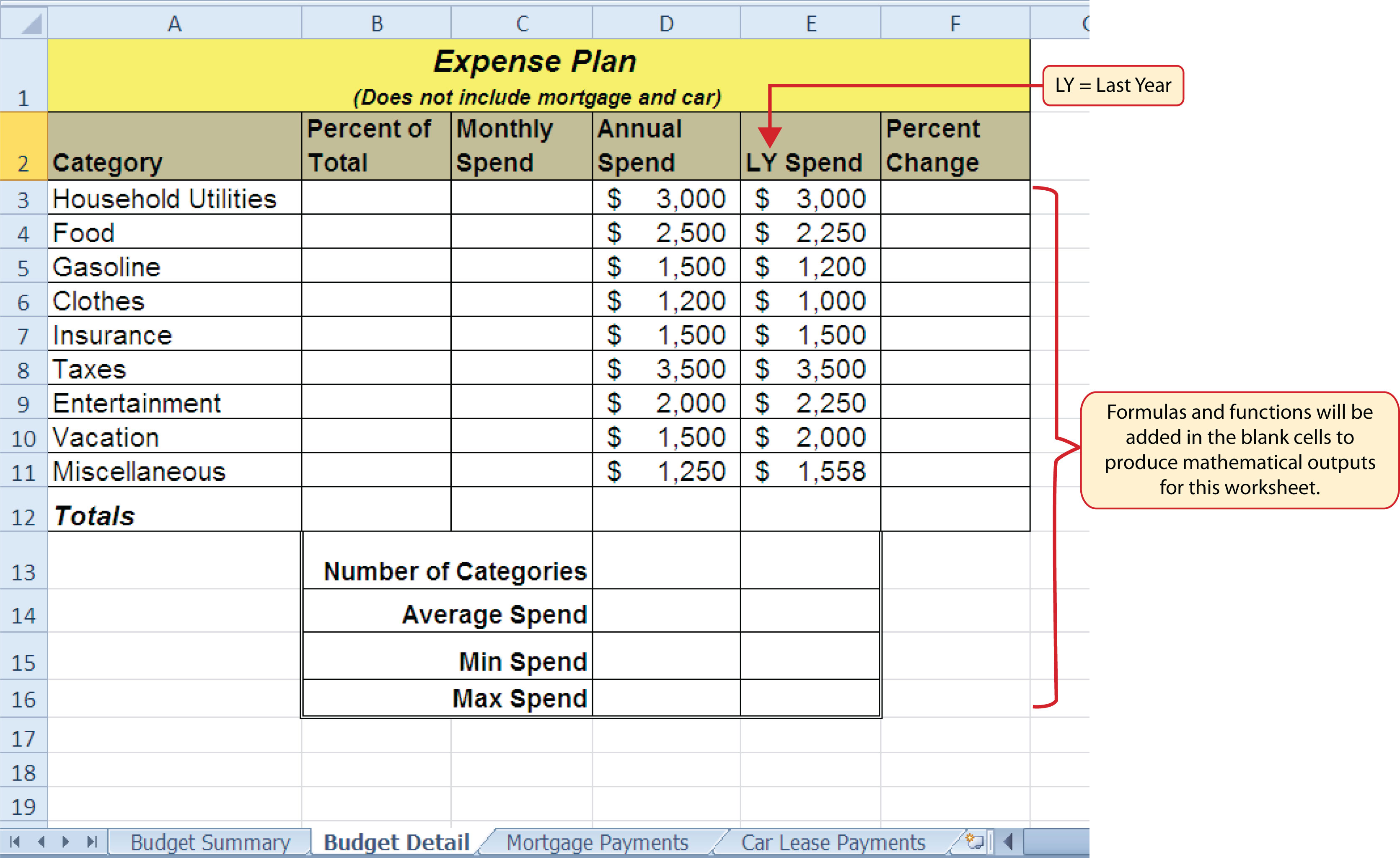Table 2.1 Spend Category Definitions

Category Definition
Household Utilities Money spent on electricity, heat, and water and on cable, phone, and Internet access
Food Money spent on groceries, toiletries, and related items
Gasoline Money spent on fuel for automobiles
Clothes Money spent on clothes, shoes, and accessories
Insurance Money spent on homeowner’s or automobile insurance
Taxes Money spent on school and property taxes (this example of the personal budget assumes that we own property).
Entertainment Money spent on entertainment, including dining out, movie and theater tickets, parties, and so on
Vacation Money spent on vacations
Miscellaneous Includes any other spending categories, such as textbooks, software, journals, school or work supplies, and so on

The first formula that we will add to the Budget Detail worksheet will calculate the Monthly Spend values. The formula will be constructed so that it takes the values in the Annual Spend column and divides them by 12. This will show how much money will be spent per month for each of the categories listed in Column A. The following explains how this formula is created:

1. Click the Budget Detail worksheet tab to open the worksheet.
2. Click cell C3.
3. Type an equal sign (=). When the first character entered into a cell location is an equal sign, it signals Excel to perform a calculation or produce a logical output.
4. Type D3. This adds D3 to the formula, which is now a cell reference. Excel will use whatever value is entered into cell D3 to produce an output.
5. Type the slash symbol (/). This is the symbol for division in Excel. As shown in Table 2.2 "Excel Mathematical Operators", the mathematical operators in Excel are slightly different from those found on a typical calculator.
6. Type the number 12. This divides the value in cell D3 by 12. In this formula, a number, or constantNumerical value used in the construction of formulas. Constants should have some universal quality that does not change, such as days in a week or minutes in an hour., is used instead of a cell reference because it will not change. In other words, there will always be 12 months in a year.
7. Press the ENTER key.

Table 2.2 Excel Mathematical Operators

Symbol Operation
Subtraction
/ Division
* Multiplication
^ Power/Exponent

### Why?

Use Cell References

Cell references enable Excel to dynamically produce new outputs when one or more inputs in the referenced cells are changed. Cell references also allow you to trace how outputs are being calculated in a formula. As a result, you should never use a calculator to determine a mathematical output and type it into the cell location of a worksheet. Doing so eliminates Excel’s cell-referencing benefits as well as your ability to trace a formula to determine how outputs are being produced.

Figure 2.3 "Adding a Formula to a Worksheet" shows how the formula appears in cell C3 before you press the ENTER key. Figure 2.4 "Formula Output for Monthly Spend" shows the output of the formula after you press the ENTER key. The monthly spend for Household Utilities is \$250 because the formula is taking the Annual Spend in cell D3 and dividing it by 12. If the value in cell D3 is changed, the formula automatically produces a new output. We are calculating the spend per month for each category because people often get paid and are billed for these items on a monthly basis. This formula allows you to compare your monthly income to your monthly bills to determine whether you have enough income to pay these expenses.

Figure 2.3 Adding a Formula to a Worksheet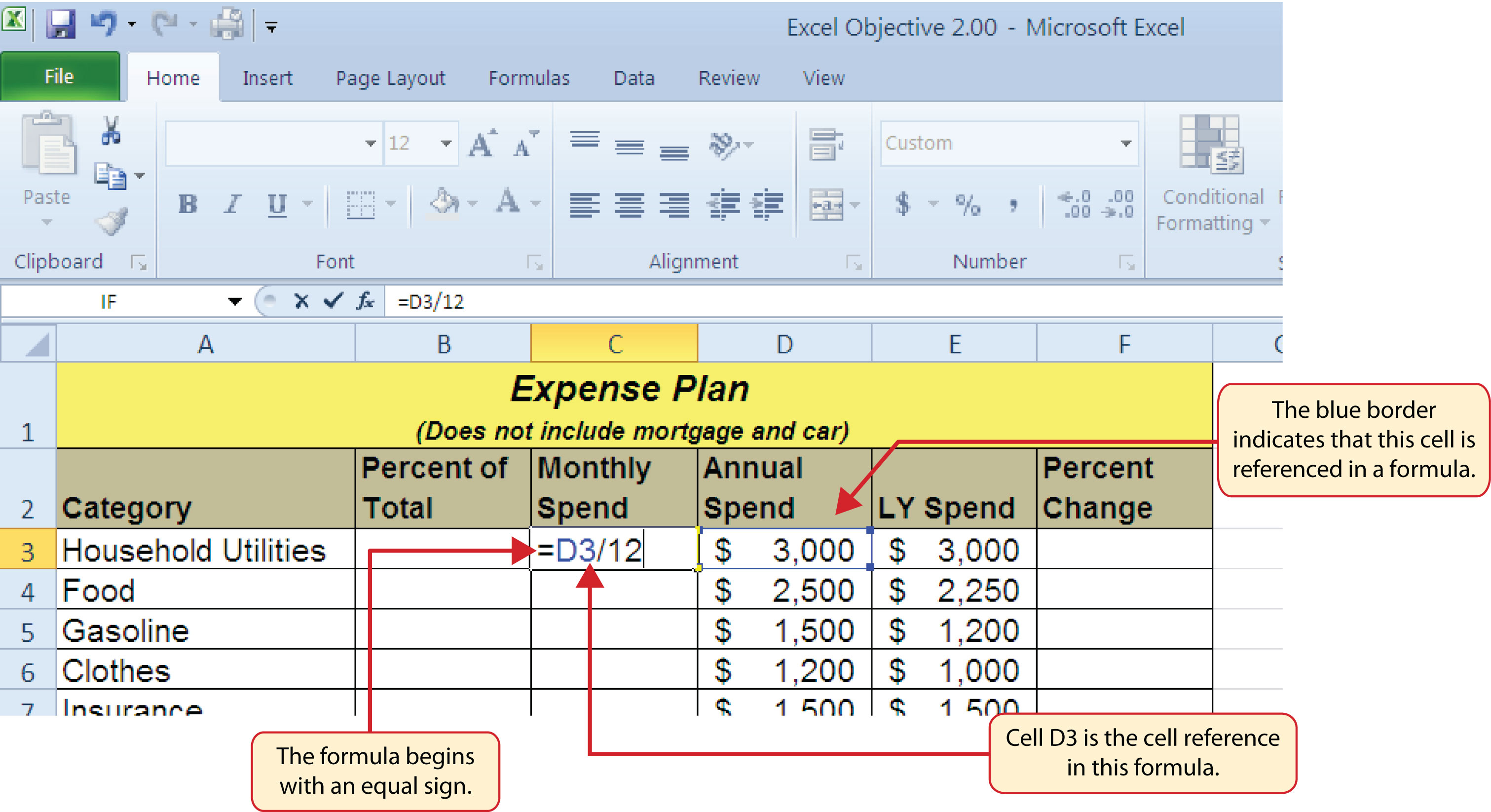Figure 2.4 Formula Output for Monthly Spend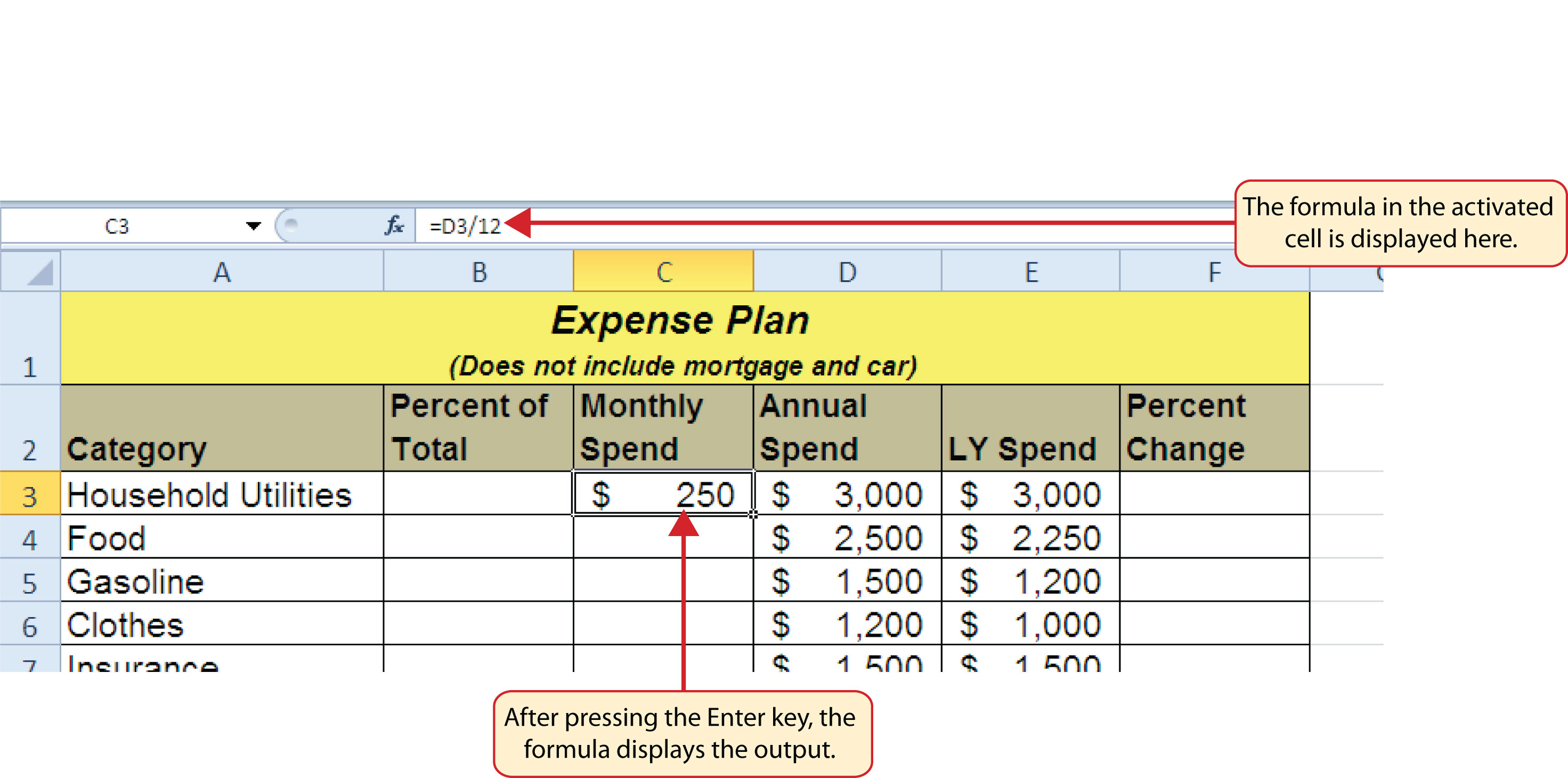### Why?

Use Universal Constants

If you are using constants, or numerical values, in an Excel formula, they should be universal constants that do not change, such as the number of days in a week, weeks in a year, and so on. Do not type the values that exist in cell locations into an Excel formula. This will eliminate Excel’s cell-referencing benefits, which means if the value in the cell location you are using in a formula is changed, Excel will not be able to produce a new output.

## Relative References (Copying and Pasting Formulas)

Follow-along file: Continue with Excel Objective 2.00. (Use file Excel Objective 2.01 if starting here.)

Once a formula is typed into a worksheet, it can be copied and pasted to other cell locations. For example, Figure 2.4 "Formula Output for Monthly Spend" shows the output of the formula that was entered into cell C3. However, this calculation needs to be performed for the rest of the cell locations in Column C. Since we used the D3 cell reference in the formula, Excel automatically adjusts that cell reference when the formula is copied and pasted into the rest of the cell locations in the column. This is called relative referencingExcel automatically adjusts a cell reference used in a formula or function relative to its original location when it is pasted into new cell locations. and is demonstrated as follows:

1. Click cell C3.
2. Click the Copy button in the Home tab of the Ribbon.
3. Highlight the range C4:C11.
4. Click the Paste button in the Home tab of the Ribbon.
5. Double click cell C6. Notice that the cell reference in the formula is automatically changed to D6.
6. Press the ENTER key.

Figure 2.5 "Relative Reference Example" shows the outputs added to the rest of the cell locations in the Monthly Spend column. For each row, the formula takes the value in the Annual Spend column and divides it by 12. You will also see that cell D6 has been double clicked to show the formula. Notice that Excel automatically changed the original cell reference of D3 to D6. This is the result of relative referencing, which means Excel automatically adjusts a cell reference relative to its original location when it is pasted into new cell locations. In this example, the formula was pasted into eight cell locations below the original cell location. As a result, Excel increased the row number of the original cell reference by a value of one for each row it was pasted into.

Figure 2.5 Relative Reference Example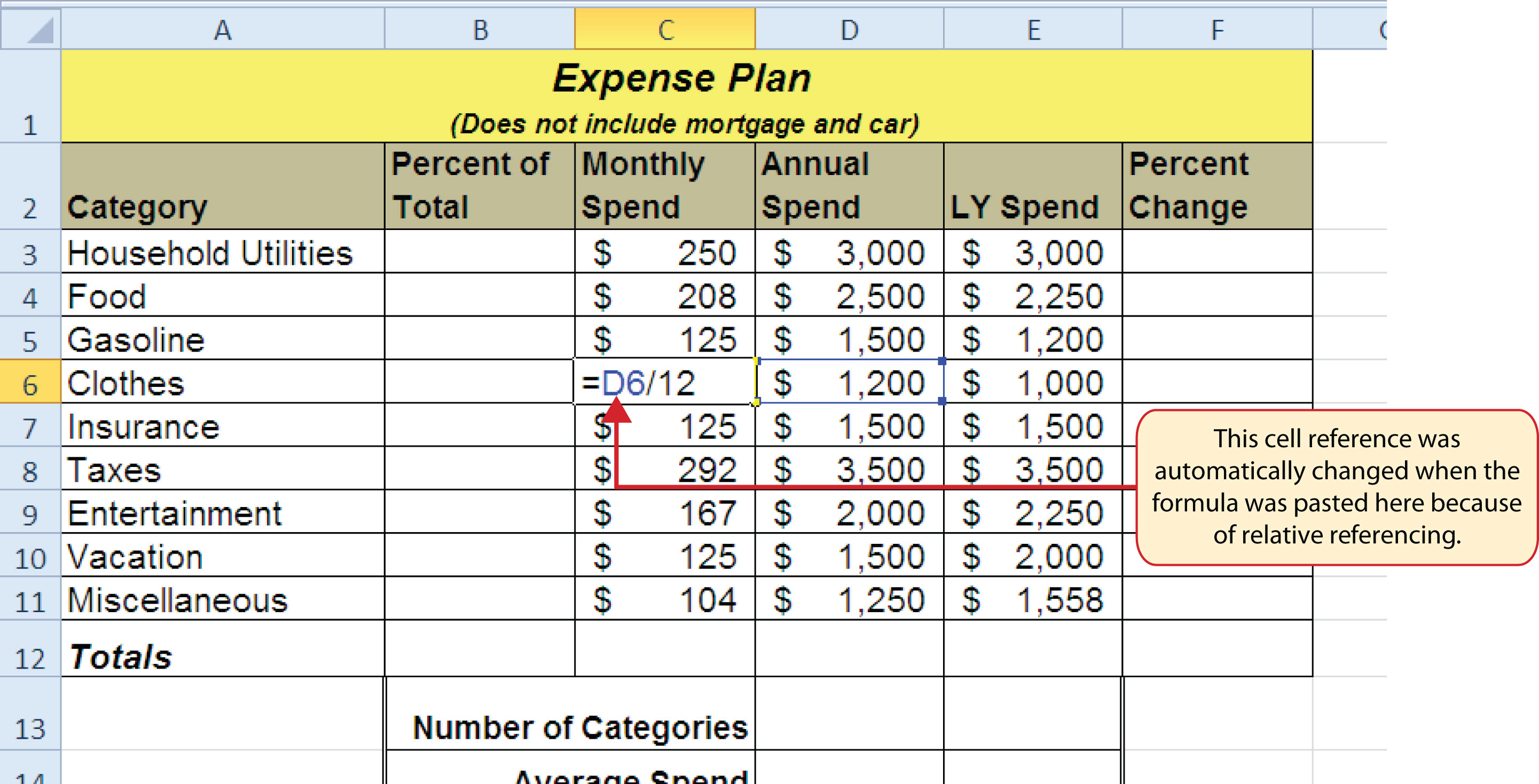### Why?

Use Relative Referencing

Relative referencing is a convenient feature in Excel. When you use cell references in a formula, Excel automatically adjusts the cell references when the formula is pasted into new cell locations. If this feature were not available, you would have to manually retype the formula when you want the same calculation applied to other cell locations in a column or row.

## Creating Complex Formulas (Controlling the Order of Operations)

Follow-along file: Continue with Excel Objective 2.00. (Use file Excel Objective 2.02 if starting here.)

The next formula to be added to the Personal Budget workbook is the percent change over last year. This formula determines the difference between the values in the LY (Last Year) Spend column and shows the difference in terms of a percentage. This requires that the order of mathematical operations be controlled to get an accurate result. Table 2.3 "Standard Order of Mathematical Operations" shows the standard order of operations for a typical formula. To change the order of operations shown in the table, we use parentheses to process certain mathematical calculations first. This formula is added to the worksheet as follows:

1. Click cell F3 in the Budget Detail worksheet.
2. Type an equal sign (=).
3. Type an open parenthesis (().
4. Click cell D3. This will add a cell reference to cell D3 to the formula. When building formulas, you can click cell locations instead of typing them.
5. Type a minus sign ().
6. Click cell E3 to add this cell reference to the formula.
7. Type a closing parenthesis ()).
8. Type the slash (/) symbol for division.
9. Click cell E3. This completes the formula that will calculate the percent change of last year’s actual spent dollars vs. this year’s budgeted spend dollars (see Figure 2.6 "Adding the Percent Change Formula").
10. Press the ENTER key.
11. Click cell F3 to activate it.
12. Place the mouse pointer over the Auto Fill Handle.
13. When the mouse pointer turns from a white block plus sign to a black plus sign, click and drag down to cell F11. This pastes the formula into the range F4:F11.

Table 2.3 Standard Order of Mathematical Operations

Symbol Order
^ First: Excel executes any exponential computations first.
* or / Second: Excel performs any multiplication or division computations second. When there are multiple instances of these computations in a formula, they are executed in order from left to right.
+ or − Third: Excel performs any addition or subtraction computations third. When there are multiple instances of these computations in a formula, they are executed in order from left to right.
( ) Override Standard Order: Any mathematical computations placed in parentheses are performed first and override the standard order of operations. If there are layers of parentheses used in a formula, Excel computes the innermost parentheses first and the outermost parentheses last.

Figure 2.6 "Adding the Percent Change Formula" shows the formula that was added to the Budget Detail worksheet to calculate the percent change in spending. The parentheses were added to this formula to control the order of operations. Any mathematical computations placed in parentheses are executed first before the standard order of mathematical operations (see Table 2.3 "Standard Order of Mathematical Operations"). In this case, if parentheses were not used, Excel would produce an erroneous result for this worksheet.

Figure 2.6 Adding the Percent Change FormulaFigure 2.7 "Removing the Parentheses from the Percent Change Formula" shows the result of the percent change formula if the parentheses are removed. The formula produces a result of a 299900% increase. Since there is no change between the LY spend and the budget Annual Spend, the result should be 0%. However, without the parentheses, Excel is following the standard order of operations. This means the value in cell E3 will be divided by E3 first (3,000/3,000), which is 1. Then, the value of 1 will be subtracted from the value in cell D3 (3,000−1), which is 2,999. Since cell F3 is formatted as a percentage, Excel expresses the output as an increase of 299900%.

Figure 2.7 Removing the Parentheses from the Percent Change Formula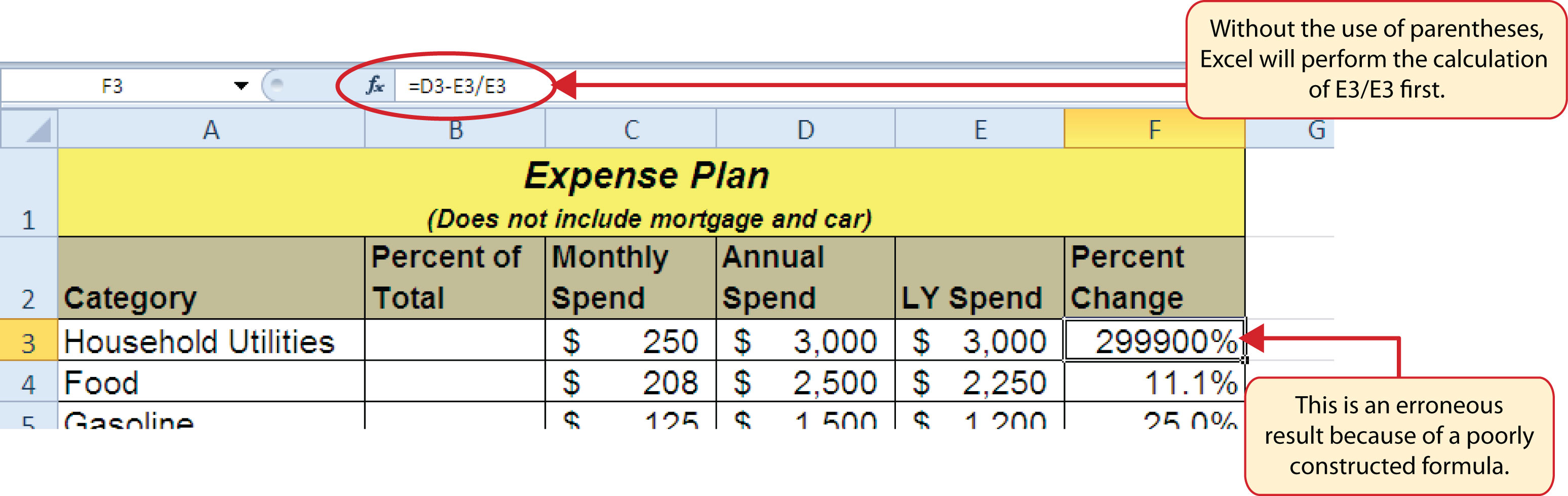### Integrity Check

Does the Output of Your Formula Make Sense?

It is important to note that the accuracy of the output produced by a formula depends on how it is constructed. Therefore, always check the result of your formula to see whether it makes sense with data in your worksheet. As shown in Figure 2.7 "Removing the Parentheses from the Percent Change Formula", a poorly constructed formula can give you an inaccurate result. In other words, you can see that there is no change between the Annual Spend and LY Spend for Household Utilities. Therefore, the result of the formula should be 0%. However, since the parentheses were removed in this case, the formula is clearly producing an erroneous result.

### Skill Refresher: Formulas

1. Type an equal sign (=).
2. Click or type a cell location. If using constants, type a number.
3. Type a mathematical operator.
4. Click or type a cell location. If using constants, type a number.
5. Use parentheses where necessary to control the order of operations.
6. Press the ENTER key.

## Auditing Formulas

Follow-along file: Continue with Excel Objective 2.00. (Use file Excel Objective 2.03 if starting here.)

Excel provides a few tools that you can use to review the formulas entered into a worksheet. For example, instead of showing the outputs for the formulas used in a worksheet, you can have Excel show the formula as it was entered in the cell locations. This is demonstrated as follows:

1. With the Budget Detail worksheet open, click the Formulas tab of the Ribbon.
2. Click the Show Formulas button in the Formula Auditing group of commands. This displays the formulas in the worksheet instead of showing the mathematical outputs.
3. Click the Show Formulas button again. The worksheet returns to showing the output of the formulas.

Figure 2.8 "Show Formulas Command" shows the Budget Detail worksheet after activating the Show Values command in the Formulas tab of the Ribbon. As shown in the figure, this command allows you to view and check all the formulas in a worksheet without having to click each cell individually. After activating this command, the column widths in your worksheet increase significantly. The column widths were adjusted for the worksheet shown in Figure 2.8 "Show Formulas Command" so all columns can be seen. The column widths return to their previous width when the Show Formulas command is deactivated.

Figure 2.8 Show Formulas Command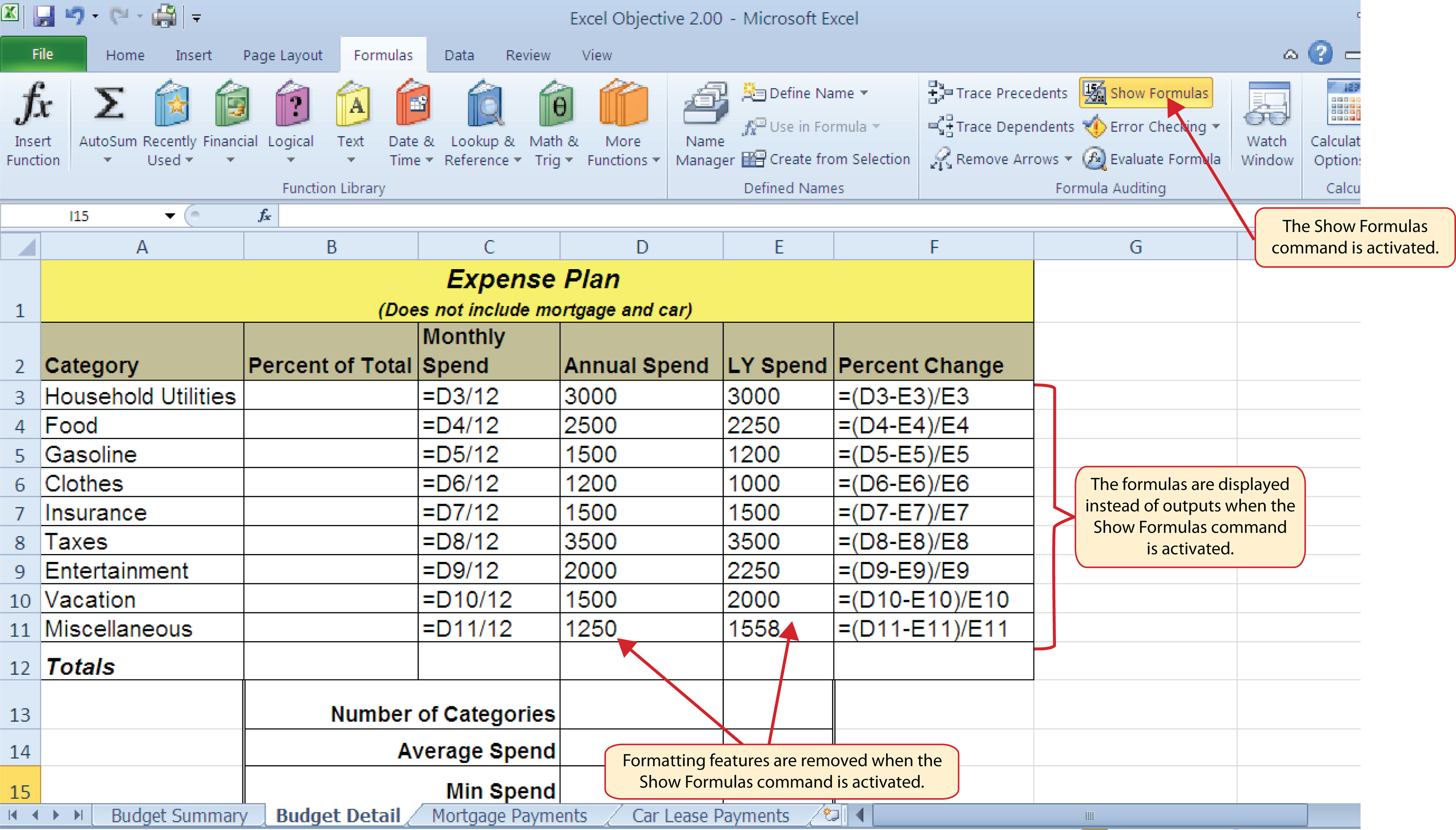### Skill Refresher: Show Formulas

1. Click the Formulas tab on the Ribbon.
2. Click the Show Formulas button in the Formula Auditing group of commands.
3. Click the Show Formulas button again to show formula outputs.

### Mouseless Commands

Show Formulas

• Hold down the CTRL key while pressing the accent symbol (`).

Two other tools in the Formula Auditing group of commands are the Trace Precedents and Trace Dependents commands. These commands are used to trace the cell references used in a formula. The Trace Dependents command shows where any given cell is referenced in a formula. The Trace Precedents command shows what cells have been referenced in a formula that exists in an activated cell. The following is a demonstration of these commands:

1. Click cell D3 in the Budget Detail worksheet.
2. Click the Trace Dependents button in the Formula Auditing group of commands in the Formulas tab of the Ribbon. A double blue arrow appears, pointing to cell locations C3 and F3 (see Figure 2.9 "Trace Dependents Example"). This indicates that cell D3 is referenced in formulas that are entered in cells C3 and F3.
3. Click the Remove Arrows command in the Formula Auditing group of commands in the Formulas tab of the Ribbon. This removes the Trace Dependents arrow.
4. Click cell F3 in the Budget Detail worksheet.
5. Click the Trace Precedents button in the Formula Auditing group of commands in the Formulas tab of the Ribbon. A blue arrow running through cells D3 and E3 and pointing to cell F3 appears (see Figure 2.10 "Trace Precedents Example"). This indicates that cells D3 and E3 are references in a formula entered in cell F3.
6. Click the Remove Arrows command in the Formula Auditing group of commands in the Formulas tab of the Ribbon. This removes the Trace Precedents arrow.

Figure 2.9 "Trace Dependents Example" shows the Trace Dependents arrow on the Budget Detail worksheet. The blue dot represents the activated cell. The arrows indicate where the cell is referenced in formulas.

Figure 2.9 Trace Dependents Example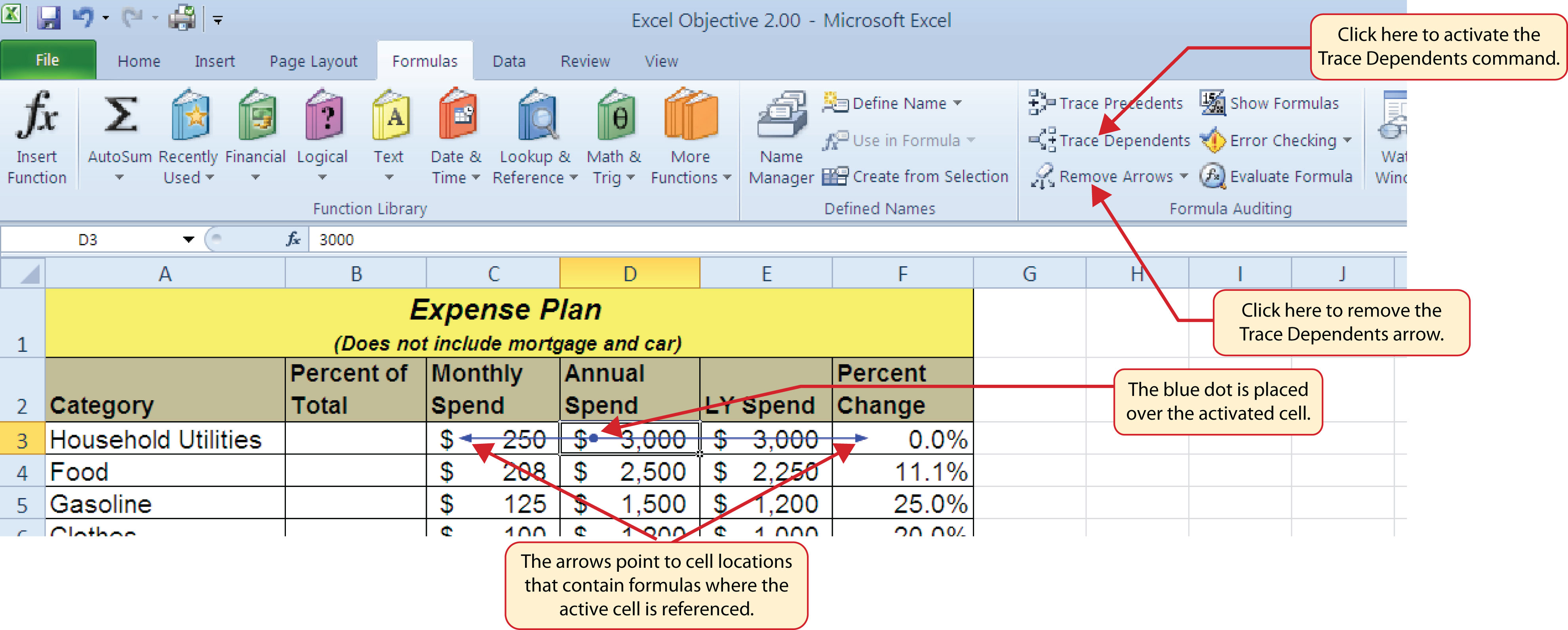Figure 2.10 "Trace Precedents Example" shows the Trace Precedents arrow on the Budget Detail worksheet. The blue dots on this arrow indicate the cells that are referenced in the formula contained in the activated cell. The arrow is pointing to the activated cell location that contains the formula.

Figure 2.10 Trace Precedents Example### Skill Refresher: Trace Dependents

1. Click a cell location that contains a number or formula.
2. Click the Formulas tab on the Ribbon.
3. Click the Trace Dependents button in the Formula Auditing group of commands.
4. Use the arrow(s) to determine where the cell is referenced in formulas and functions.
5. Click the Remove Arrows button to remove the arrows from the worksheet.

### Skill Refresher: Trace Precedents

1. Click a cell location that contains a formula or function.
2. Click the Formulas tab on the Ribbon.
3. Click the Trace Precedents button in the Formula Auditing group of commands.
4. Use the dot(s) along the line to determine what cells are referenced in the formula or function.
5. Click the Remove Arrows button to remove the line with the dots.

### Key Takeaways

• Mathematical computations are conducted through formulas and functions.
• An equal sign (=) precedes all formulas and functions.
• Formulas and functions must be created with cell references to conduct what-if scenarios where mathematical outputs are recalculated when one or more inputs are changed.
• Mathematical operators on a typical calculator are different from those used in Excel. Table 2.2 "Excel Mathematical Operators" lists Excel mathematical operators.
• When using numerical values in formulas and functions, only use universal constants that do not change, such as days in a week, months in a year, and so on.
• Relative referencing automatically adjusts the cell references in formulas and functions when they are pasted into new locations on a worksheet. This eliminates the need to retype formulas and functions when they are needed in multiple rows or columns on a worksheet.
• Parentheses must be used to control the order of operations when necessary for complex formulas.
• Formula auditing tools such as Trace Dependents, Trace Precedents, and Show Formulas should be used to check the integrity of formulas that have been entered into a worksheet.

### Exercises

1. Which of the following terms best describes how Excel is able to change the outputs of formulas and functions when one or more inputs are changed?

1. absolute references
2. cell references
3. relative references
4. dynamic output referencing
2. Which of the following best describes the proper use of numbers when constructing formulas in Excel?

1. Numbers cannot be used in the construction of formulas. You can use only cell locations.
2. You cannot combine numbers and cell locations in a formula. This will produce an error.
3. Numbers should always be used when creating formulas in Excel.
4. Numbers should be used when constructing formulas with constants that do not change, such as the days in a week, months in a year, and so on.
3. Which of the following will be calculated first in the formula =((C10−D2)*A9)+B5*C5?

1. D2*A9
2. B5*C5
3. C10−D2
4. A9 * the result of (C10−D2)
4. Which of the following formula auditing features would you use if you wanted to see where a specific cell location was referenced in formulas entered into a worksheet?

1. Show Formulas
2. Trace Precedents
3. Trace Dependents
4. Show Cell Reference Mapping

## 2.2 Statistical Functions

### Learning Objectives

1. Use the SUM function to calculate totals.
2. Use absolute references to calculate percent of totals.
3. Use the COUNT function to count cell locations with numerical values.
4. Use the AVERAGE function to calculate the arithmetic mean.
5. Use the MAX and MIN functions to find the highest and lowest values in a range of cells.
6. Learn how to copy and paste formulas without formats applied to a cell location.
7. Learn how to set a multiple level sort sequence for data sets that have duplicate values or outputs.

In addition to formulas, another way to conduct mathematical computations in Excel is through functions. Statistical functions apply a mathematical process to a group of cells in a worksheet. For example, the SUM function is used to add the values contained in a range of cells. A list of commonly used statistical functions is shown in Table 2.4 "Commonly Used Statistical Functions". Functions are more efficient than formulas when you are applying a mathematical process to a group of cells. If you use a formula to add the values in a range of cells, you would have to add each cell location to the formula one at a time. This can be very time-consuming if you have to add the values in a few hundred cell locations. However, when you use a function, you can highlight all the cells that contain values you wish to sum in just one step. This section demonstrates a variety of statistical functions that we will add to the Personal Budget workbook. In addition to demonstrating functions, this section also reviews percent of total calculations and the use of absolute references.

Table 2.4 Commonly Used Statistical Functions

Function Output
ABS The absolute value of a number
AVERAGE The average or arithmetic mean for a group of numbers
COUNT The number of cell locations in a range that contain a numeric character
COUNTA The number of cell locations in a range that contain a text or numeric character
MAX The highest numeric value in a group of numbers
MEDIAN The middle number in a group of numbers (half the numbers in the group are higher than the median and half the numbers in the group are lower than the median)
MIN The lowest numeric value in a group of numbers
MODE The number that appears most frequently in a group of numbers
PRODUCT The result of multiplying all the values in a range of cell locations
SQRT The positive square root of a number
STDEV.S The standard deviation for a group of numbers based on a sample
SUM The total of all numeric values in a group

## The SUM Function

Follow-along file: Continue with Excel Objective 2.00. (Use file Excel Objective 2.03 if starting here.)

The SUM function is used when you need to calculate totals for a range of cells or a group of selected cells on a worksheet. With regard to the Budget Detail worksheet, we will use the SUM function to calculate the totals in row 12. It is important to note that there are several methods for adding a function to a worksheet, which will be demonstrated throughout the remainder of this chapter. The following illustrates how a function can be added to a worksheet by typing it into a cell location:

1. Click the Budget Detail worksheet tab to open the worksheet.
2. Click cell C12.
3. Type an equal sign (=).
4. Type the function name SUM.
5. Type an open parenthesis (().
6. Click cell C3 and drag down to cell C11. This places the range C3:C11 into the function.
7. Type a closing parenthesis ()).
8. Press the ENTER key. The function calculates the total for the Monthly Spend column, which is \$1,496.

Figure 2.11 "Adding the SUM Function to the Budget Detail Worksheet" shows the appearance of the SUM function added to the Budget Detail worksheet before pressing the ENTER key.

Figure 2.11 Adding the SUM Function to the Budget Detail Worksheet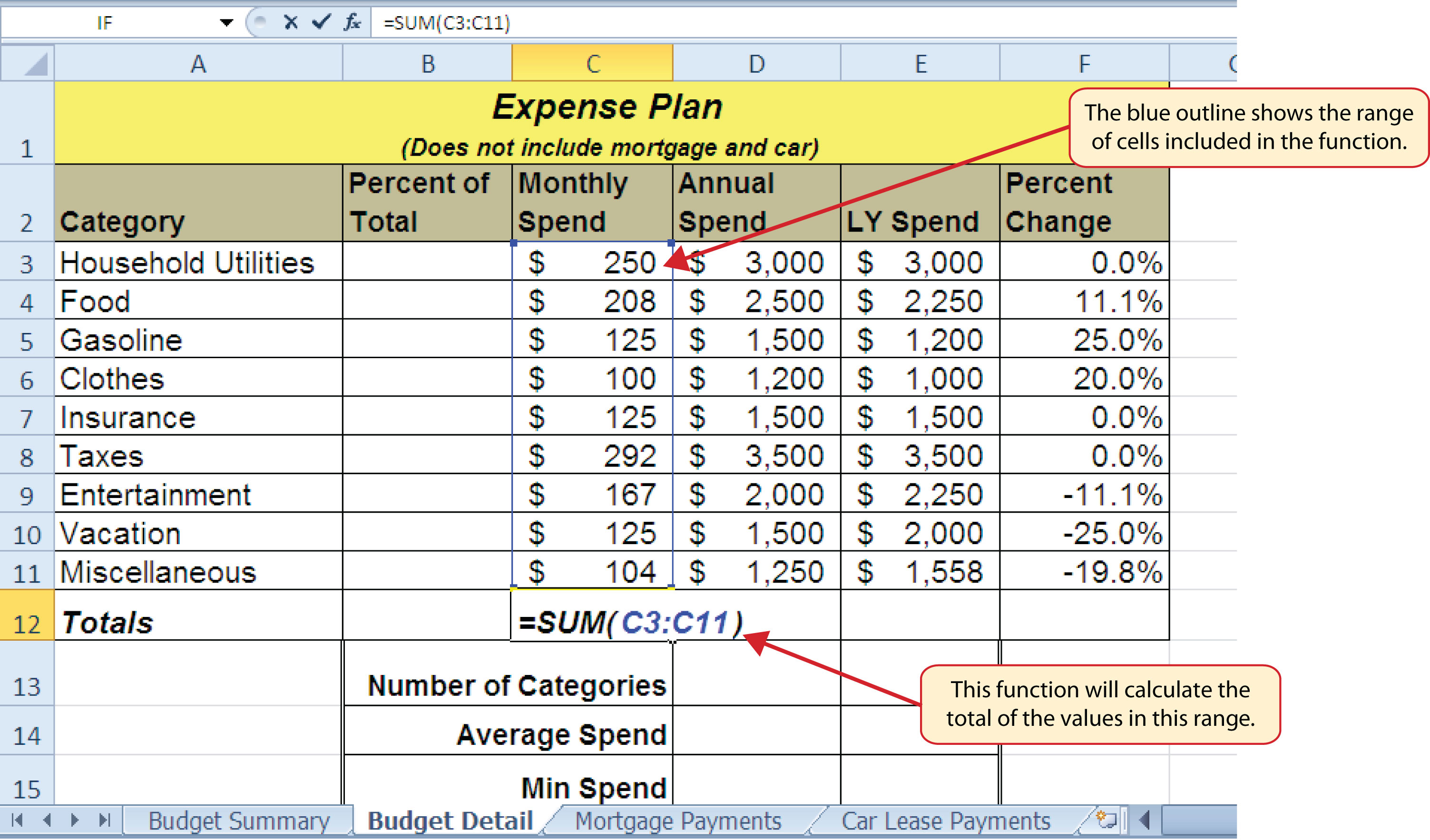As shown in Figure 2.11 "Adding the SUM Function to the Budget Detail Worksheet", the SUM function was added to cell C12. However, this function is also needed to calculate the totals in the Annual Spend and LY Spend columns. The function can be copied and pasted into these cell locations because of relative referencing. Relative referencing serves the same purpose for functions as it does for formulas. The following demonstrates how the total row is completed:

1. Click cell C12 in the Budget Detail worksheet.
2. Click the Copy button in the Home tab of the Ribbon.
3. Highlight cells D12 and E12.
4. Click the Paste button in the Home tab of the Ribbon. This pastes the SUM function into cells D12 and E12 and calculates the totals for these columns.
5. Click cell F11.
6. Click the Copy button in the Home tab of the Ribbon.
7. Click cell F12, then click the Paste button in the Home tab of the Ribbon. Since we now have totals in row 12, we can paste the percent change formula into this row.

Figure 2.12 "Results of the SUM Function in the Budget Detail Worksheet" shows the output of the SUM function that was added to cells C12, D12, and E12. In addition, the percent change formula was copied and pasted into cell F12. Notice that this version of the budget is planning a 1.7% decrease in spending compared to last year.

Figure 2.12 Results of the SUM Function in the Budget Detail Worksheet### Integrity Check

Cell Ranges in Statistical Functions

When you intend to use a statistical function on a range of cells in a worksheet, make sure there are two cell locations separated by a colon and not a comma. If you enter two cell locations separated by a comma, the function will produce an output but it will be applied to only two cell locations instead of a range of cells. For example, the SUM function shown in Figure 2.13 "SUM Function Adding Two Cell Locations" will add only the values in cells C3 and C11, not the range C3:C11.

Figure 2.13 SUM Function Adding Two Cell Locations## Absolute References (Calculating Percent of Totals)

Follow-along file: Continue with Excel Objective 2.00. (Use file Excel Objective 2.04 if starting here.)

Since totals were added to row 12 of the Budget Detail worksheet, a percent of total calculation can be added to Column B beginning in cell B3. The percent of total calculation shows the percentage for each value in the Annual Spend column with respect to the total in cell D12. However, after the formula is created, it will be necessary to turn off Excel’s relative referencing feature before copying and pasting the formula to the rest of the cell locations in the column. Turning off Excel’s relative referencing feature is accomplished through an absolute reference. The following steps explain how this is done:

1. Click cell B3 in the Budget Detail worksheet.
2. Type an equal sign (=).
3. Click cell D3.
4. Type a forward slash (/).
5. Click cell D12.
6. Press the ENTER key. You will see that Household Utilities represents 16.7% of the Annual Spend budget (see Figure 2.14 "Adding a Formula to Calculate the Percent of Total").

Figure 2.14 Adding a Formula to Calculate the Percent of Total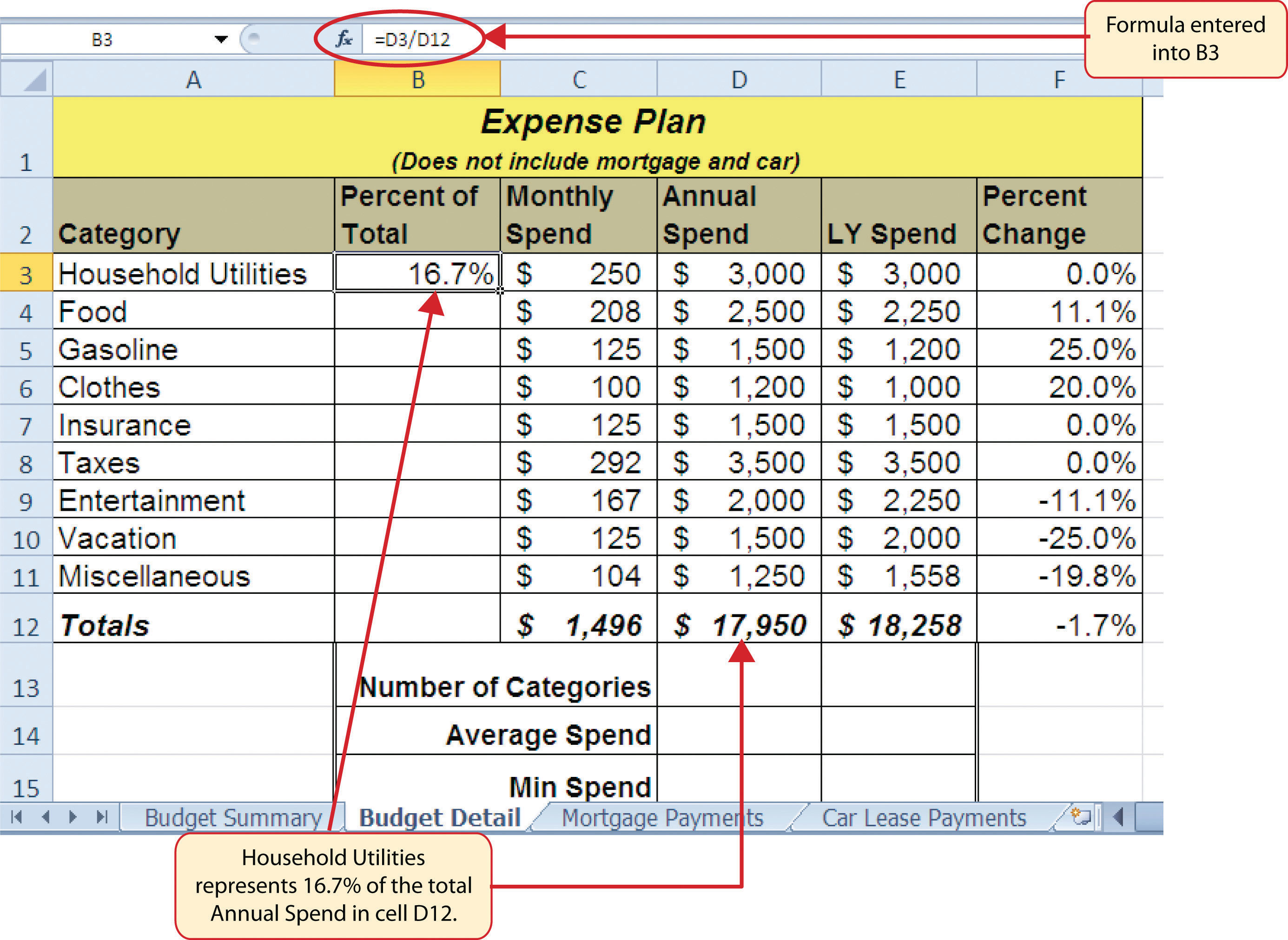Figure 2.14 "Adding a Formula to Calculate the Percent of Total" shows the completed formula that is calculating the percentage that Household Utilities Annual Spend represents to the total Annual Spend for the budget (see cell B3). Normally, we would copy this formula and paste it into the range B4:B11. However, because of relative referencing, both cell references will increase by one row as the formula is pasted into the cells below B3. This is fine for the first cell reference in the formula (D3) but not for the second cell reference (D12). Figure 2.15 "#DIV/0 Error from Relative Referencing" illustrates what happens if we paste the formula into the range B4:B12 in its current state. Notice that Excel produces the #DIV/0Error code displayed in a cell containing a formula or function that is trying to divide by zero. error code. This means that Excel is trying to divide a number by zero, which is impossible. Looking at the formula in cell B4, you see that the first cell reference was changed from D3 to D4. This is fine because we now want to divide the Annual Spend for Insurance by the total Annual Spend in cell D12. However, Excel has also changed the D12 cell reference to D13. Because cell location D13 is blank, the formula produces the #DIV/0 error code.

Figure 2.15 #DIV/0 Error from Relative ReferencingTo eliminate the divide-by-zero error shown in Figure 2.15 "#DIV/0 Error from Relative Referencing", we must add an absolute reference to cell D12 in the formula. An absolute referencePrevents Excel from using relative referencing to change a cell reference used in a formula or function; an absolute reference is applied to a cell reference by typing a dollar sign (\$) in front of the column letter and row number. prevents relative referencing from changing a cell reference in a formula. This is also referred to as locking a cell. The following explains how this is accomplished:

1. Double click cell B3.
2. Place the mouse pointer in front of D12 and click. The blinking cursor should be in front of the D in the cell reference D12.
3. Press the F4 key. You will see a dollar sign (\$) added in front of the column letter D and the row number 12. You can also type the dollar signs in front of the column letter and row number.
4. Press the ENTER key.
5. Click cell B3.
6. Click the Copy button in the Home tab of the Ribbon.
7. Highlight the range B4:B11.
8. Click the Paste button in the Home tab of the Ribbon.

Figure 2.16 "Adding an Absolute Reference to a Cell Reference in a Formula" shows the percent of total formula with an absolute reference added to D12. Notice that in cell B4, the cell reference remains D12 instead of changing to D13 as shown in Figure 2.15 "#DIV/0 Error from Relative Referencing". Also, you will see that the percentages are being calculated in the rest of the cells in the column, and the divide-by-zero error is now eliminated.

Figure 2.16 Adding an Absolute Reference to a Cell Reference in a Formula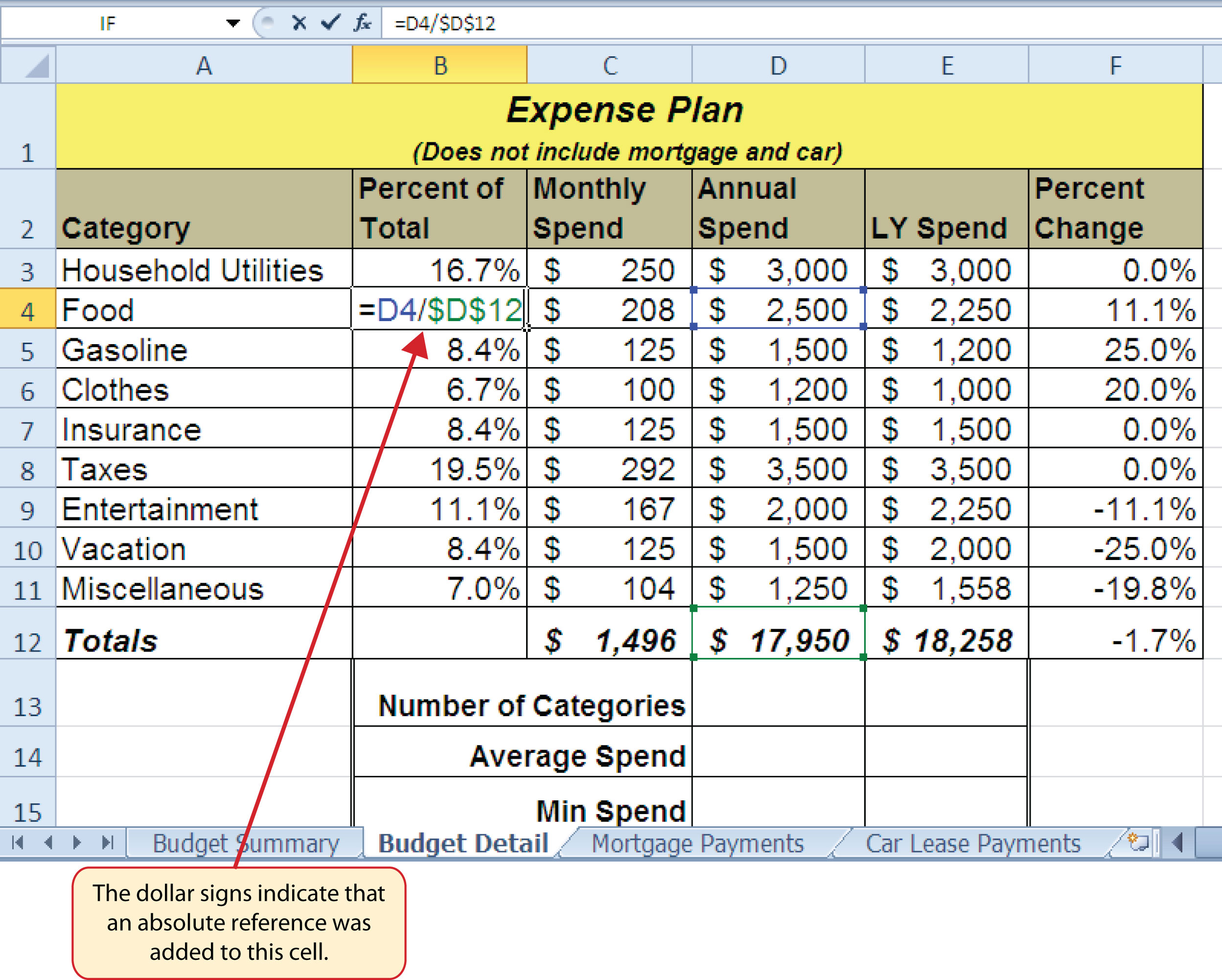### Skill Refresher: Absolute References

1. Click in front of the column letter of a cell reference in a formula or function that you do not want altered when the formula or function is pasted into a new cell location.
2. Press the F4 key or type a dollar sign (\$) in front of the column letter and row number of the cell reference.

## The COUNT Function

Follow-along file: Continue with Excel Objective 2.00. (Use file Excel Objective 2.05 if starting here.)

The next function that we will add to the Budget Detail worksheet is the COUNT function. The COUNT function is used to determine how many cells in a range contain a numeric entry. For the Budget Detail worksheet, we will use the COUNT function to count the number of items that are planned in the Annual Spend column (Column D). The following explains how the COUNT function is added to the worksheet by using the function list:

1. Click cell D13 in the Budget Detail worksheet.
2. Type an equal sign (=).
3. Type the letter C.
4. Click the down arrow on the scroll bar of the function list (see Figure 2.17 "Using the Function List to Add the COUNT Function") and find the word COUNT.
5. Double click the word COUNT from the function list.
6. Highlight the range D3:D11.
7. Type a closing parenthesis ()).
8. Press the ENTER key. The function produces an output of 9 since there are 9 items planned on the worksheet.

Figure 2.17 "Using the Function List to Add the COUNT Function" shows the function list box that appears after completing steps 2 and 3 for the COUNT function. The function list provides an alternative method for adding a function to a worksheet.

Figure 2.17 Using the Function List to Add the COUNT Function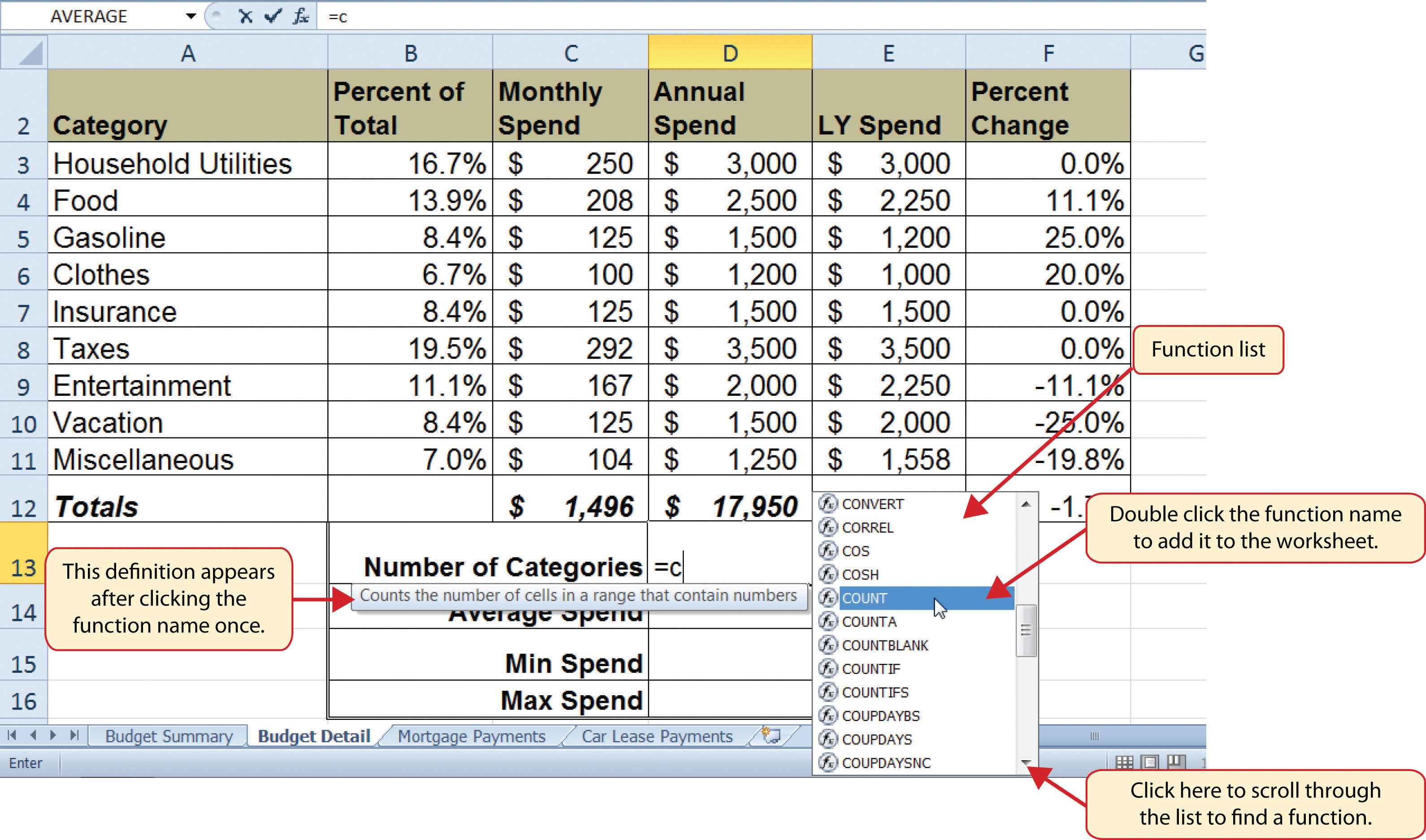Figure 2.18 "Completed COUNT Function in the Budget Detail Worksheet" shows the output of the COUNT function after pressing the ENTER key. The function counts the number of cells in the range D3:D11 that contain a numeric value. The result of 9 indicates that there are 9 categories planned for this budget.

Figure 2.18 Completed COUNT Function in the Budget Detail Worksheet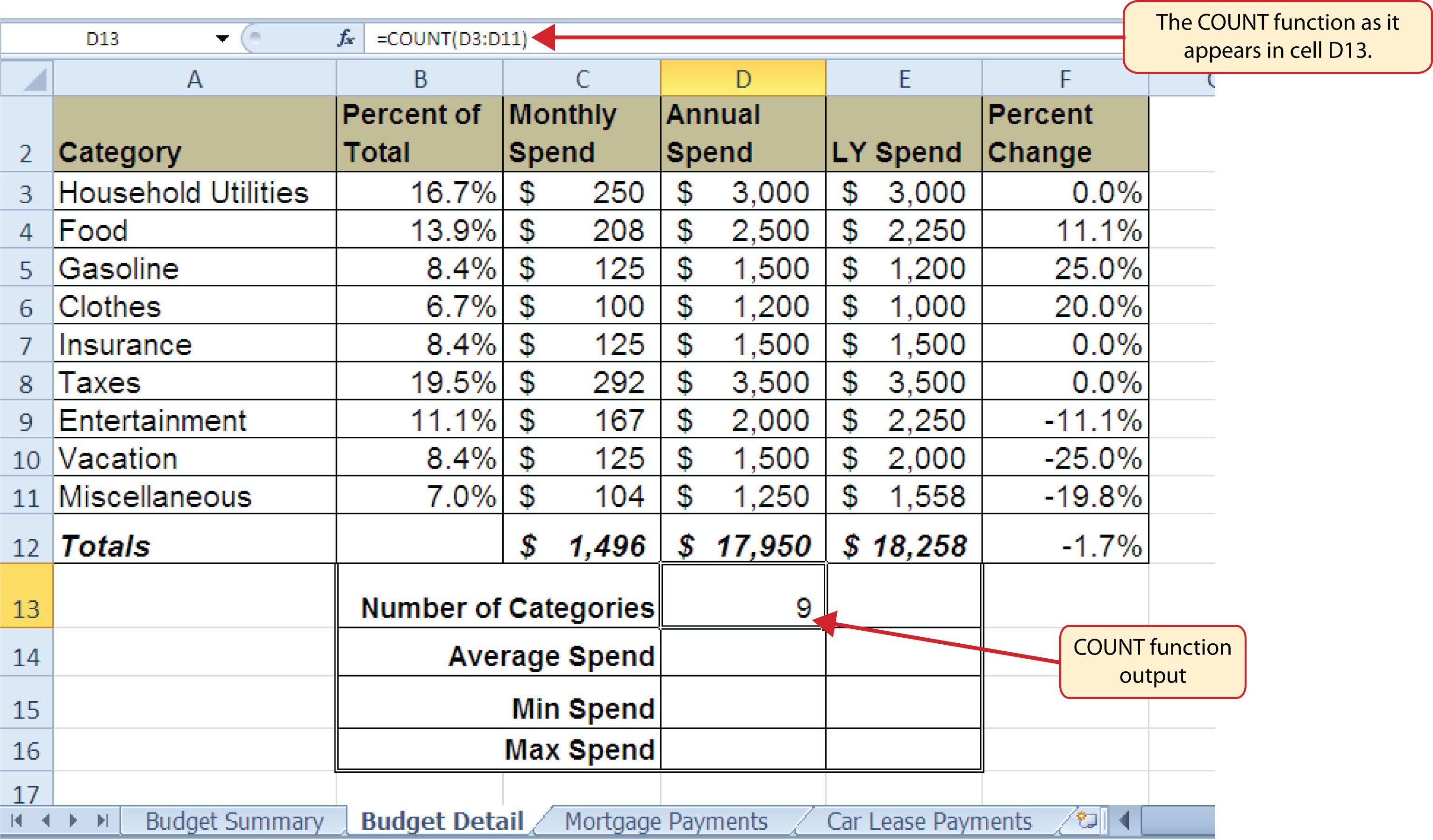## The AVERAGE Function

Follow-along file: Continue with Excel Objective 2.00. (Use file Excel Objective 2.06 if starting here.)

The next function we will add to the Budget Detail worksheet is the AVERAGE function. This function is used to calculate the arithmetic mean for a group of numbers. For the Budget Detail worksheet, we will use the function to calculate the average of the values in the Annual Spend column. We will add this to the worksheet by using the Function Library. The following steps explain how this is accomplished:

1. Click cell D14 in the Budget Detail worksheet.
2. Click the Formulas tab on the Ribbon.
3. Click the More Functions button in the Function Library group of commands.
4. Place the mouse pointer over the Statistical option from the drop-down list of options.
5. Click the AVERAGE function name from the list of functions that appear in the menu (see Figure 2.19 "Selecting the AVERAGE Function from the Function Library"). This opens the Function Arguments dialog box.
6. Click the Collapse Dialog button in the Function Arguments dialog box (see Figure 2.20 "Function Arguments Dialog Box").
7. Highlight the range D3:D11.
8. Click the Expand Dialog button in the Function Arguments dialog box (see Figure 2.21 "Selecting a Range from the Function Arguments Dialog Box"). You can also press the ENTER key to get the same result.
9. Click the OK button on the Function Arguments dialog box. This adds the AVERAGE function to the worksheet.

Figure 2.19 "Selecting the AVERAGE Function from the Function Library" illustrates how a function is selected from the Function Library in the Formulas tab of the Ribbon.

Figure 2.19 Selecting the AVERAGE Function from the Function Library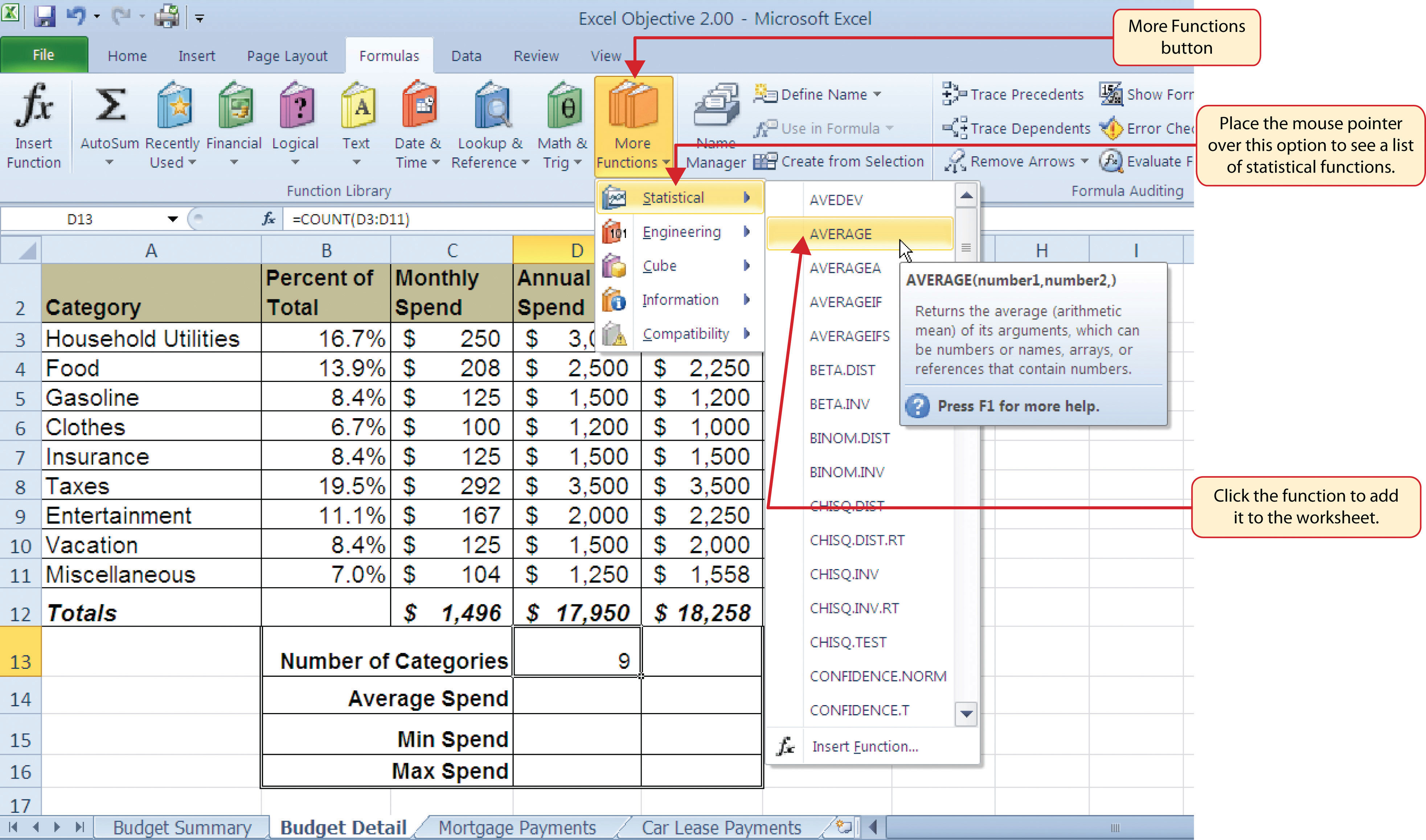Figure 2.20 "Function Arguments Dialog Box" shows the Function Arguments dialog box. This appears after a function is selected from the Function Library. The Collapse Dialog button is used to hide the dialog box so a range of cells can be highlighted on the worksheet and then added to the function.

Figure 2.20 Function Arguments Dialog Box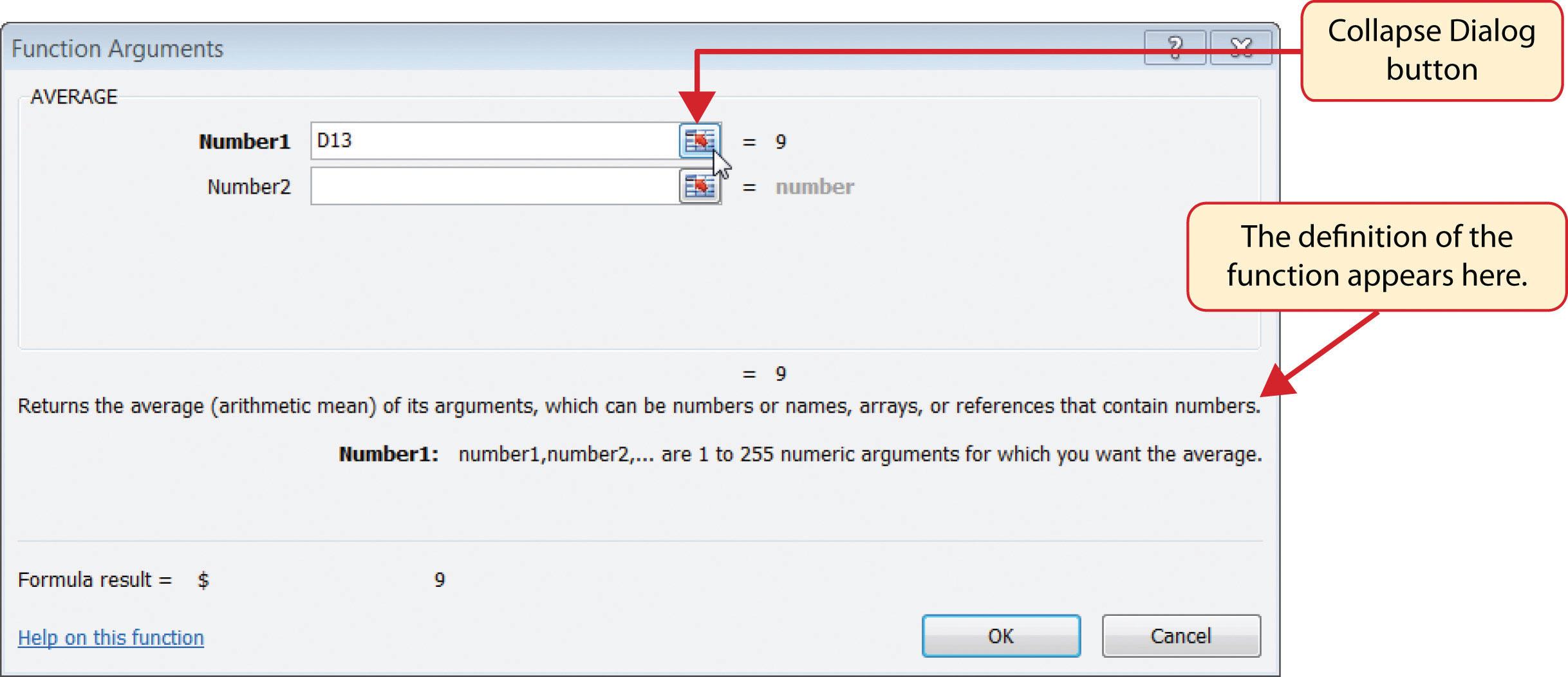Figure 2.21 "Selecting a Range from the Function Arguments Dialog Box" shows how a range of cells can be selected from the Function Arguments dialog box once it has been collapsed.

Figure 2.21 Selecting a Range from the Function Arguments Dialog Box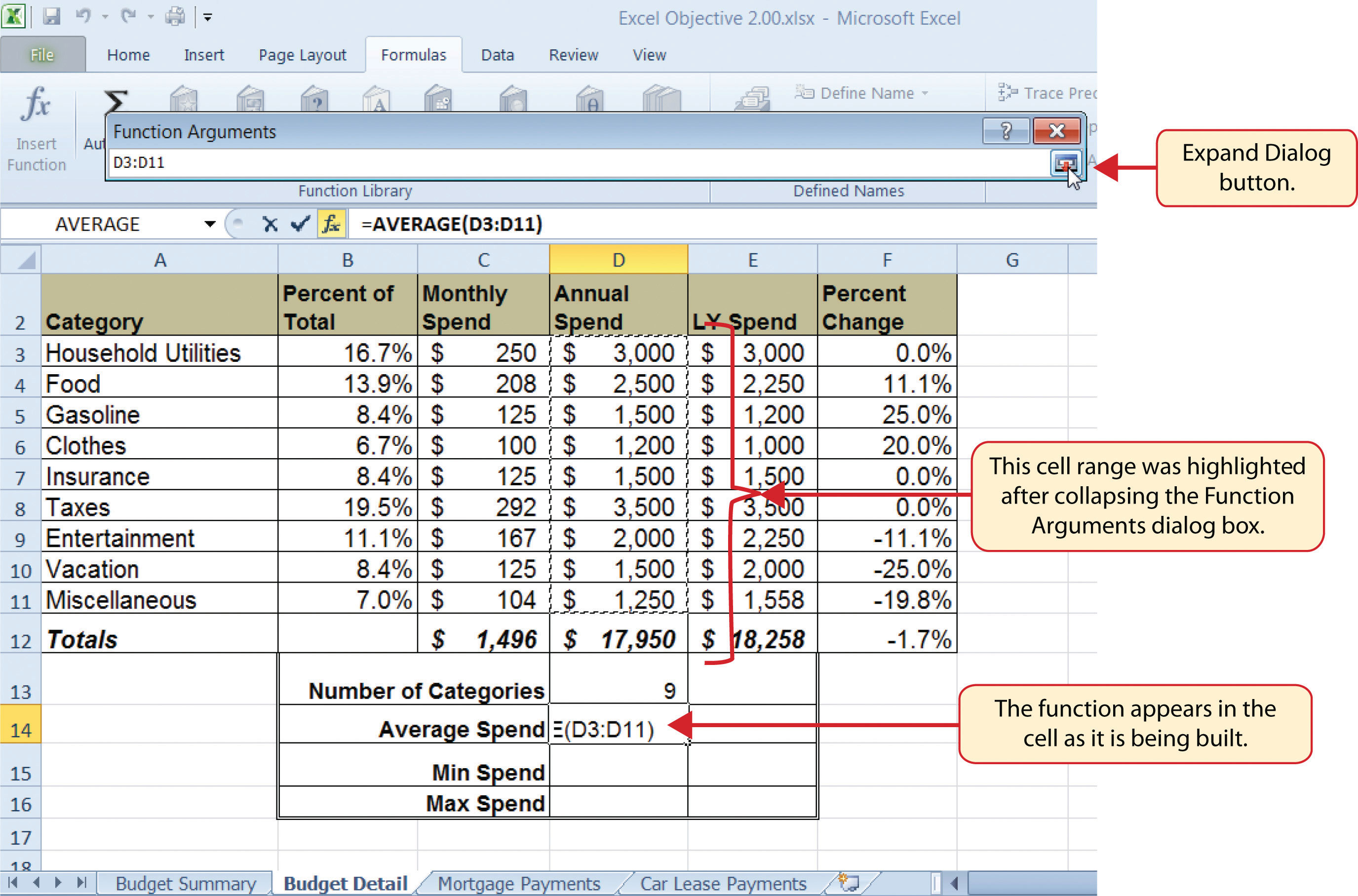Figure 2.22 "Function Arguments Dialog Box after a Cell Range Is Defined for a Function" shows the Function Arguments dialog box after the cell range is defined for the AVERAGE function. The dialog box shows the result of the function before it is added to the cell location. This allows you to assess the function output to determine whether it makes sense before adding it to the worksheet.

Figure 2.22 Function Arguments Dialog Box after a Cell Range Is Defined for a Function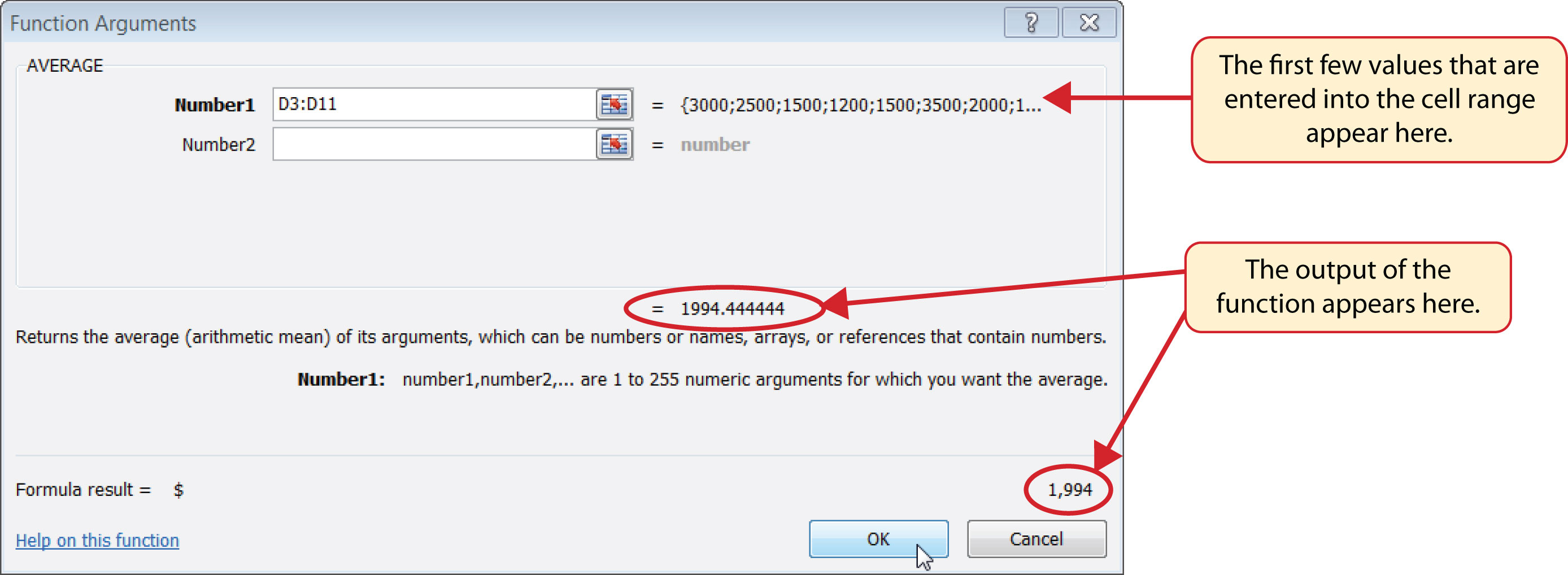Figure 2.23 "Completed AVERAGE Function" shows the completed AVERAGE function in the Budget Detail worksheet. The output of the function shows that on average we expect to spend \$1,994 for each of the categories listed in Column A of the budget. This average spend calculation per category can be used as an indicator to determine which categories are costing more or less than the average budgeted spend dollars.

Figure 2.23 Completed AVERAGE Function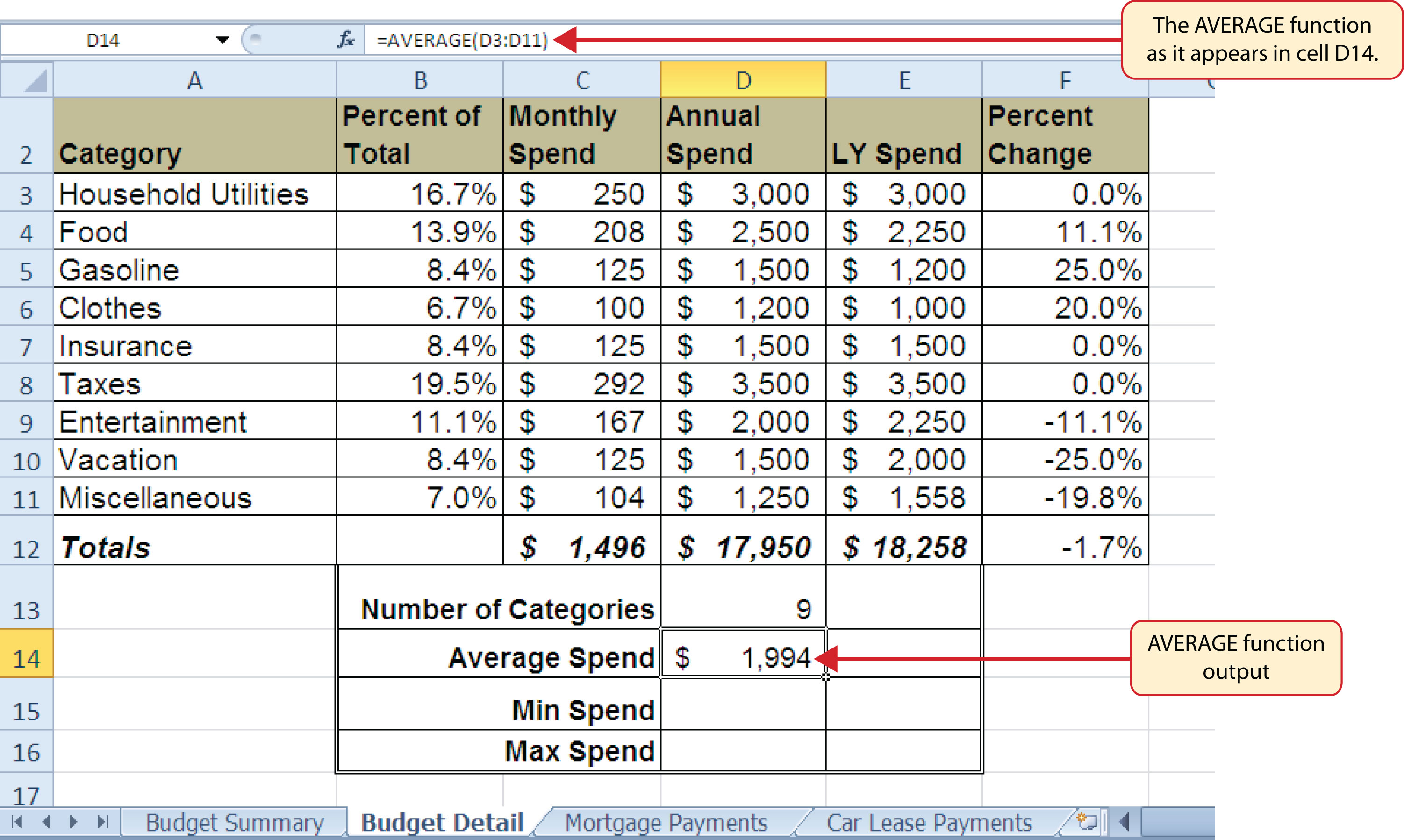## The MAX and MIN Functions

Follow-along file: Continue with Excel Objective 2.00. (Use file Excel Objective 2.07 if starting here.)

The final two statistical functions that we will add to the Budget Detail worksheet are the MAX and MIN functions. These functions identify the highest and lowest values in a range of cells. The following steps explain how to add these functions to the Budget Detail worksheet:

1. Click cell D15 in the Budget Detail worksheet.
2. Type an equal sign (=).
3. Type the word MIN.
4. Type an open parenthesis (().
5. Highlight the range D3:D11.
6. Type a closing parenthesis ()).
7. Press the ENTER key. The MIN function produces an output of \$1,200, which is the lowest value in the Annual Spend column (see Figure 2.24 "MIN Function Added to the Budget Detail Worksheet").
8. Click cell D16.
9. Type an equal sign (=).
10. Type the word MAX.
11. Type an open parenthesis (().
12. Highlight the range D3:D11.
13. Type a closing parenthesis ()).
14. Press the ENTER key. The MAX function produces an output of \$3,500. This is the highest value in the Annual Spend column (see Figure 2.25 "MAX Function Added to the Budget Detail Worksheet").

Figure 2.24 MIN Function Added to the Budget Detail Worksheet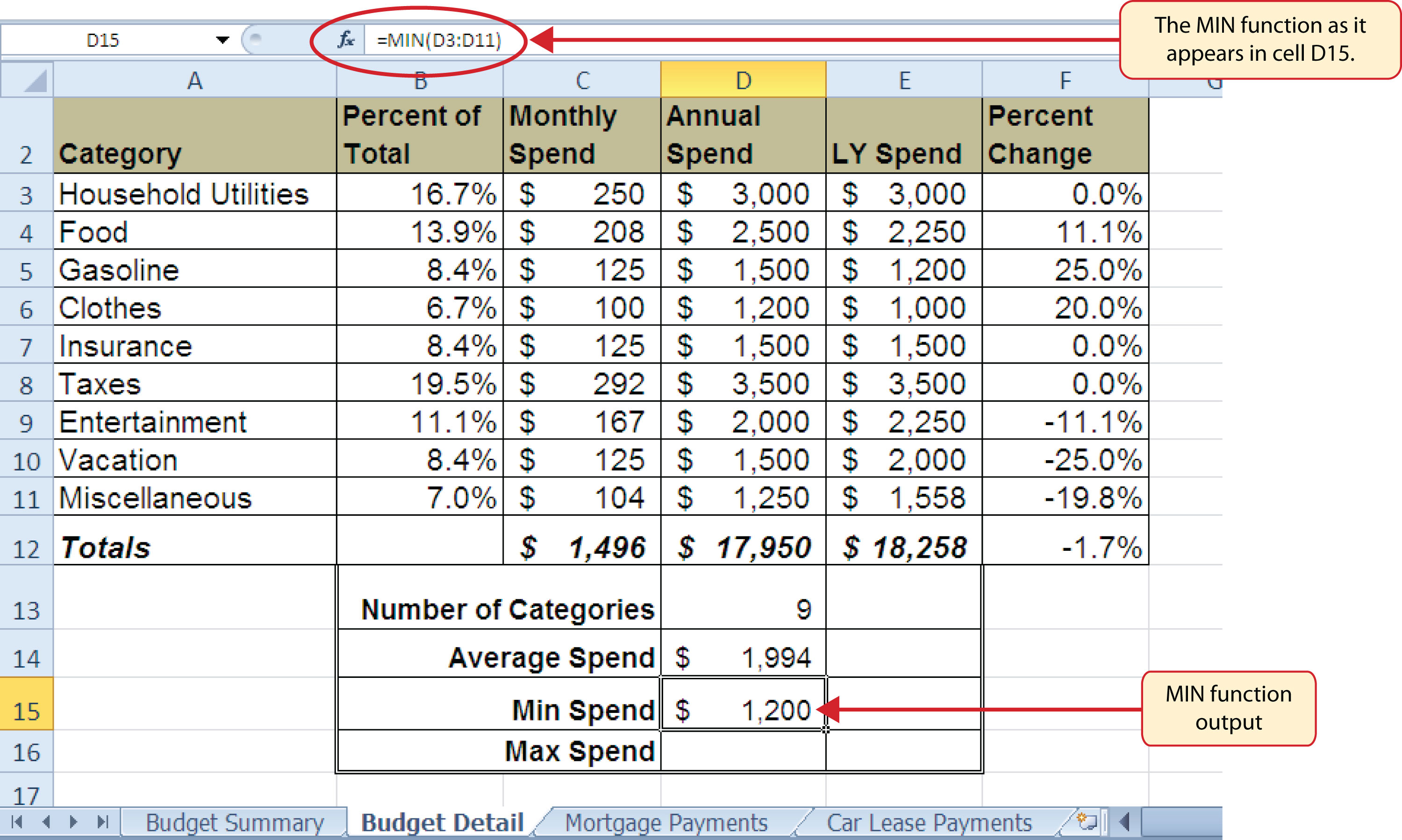Figure 2.25 MAX Function Added to the Budget Detail Worksheet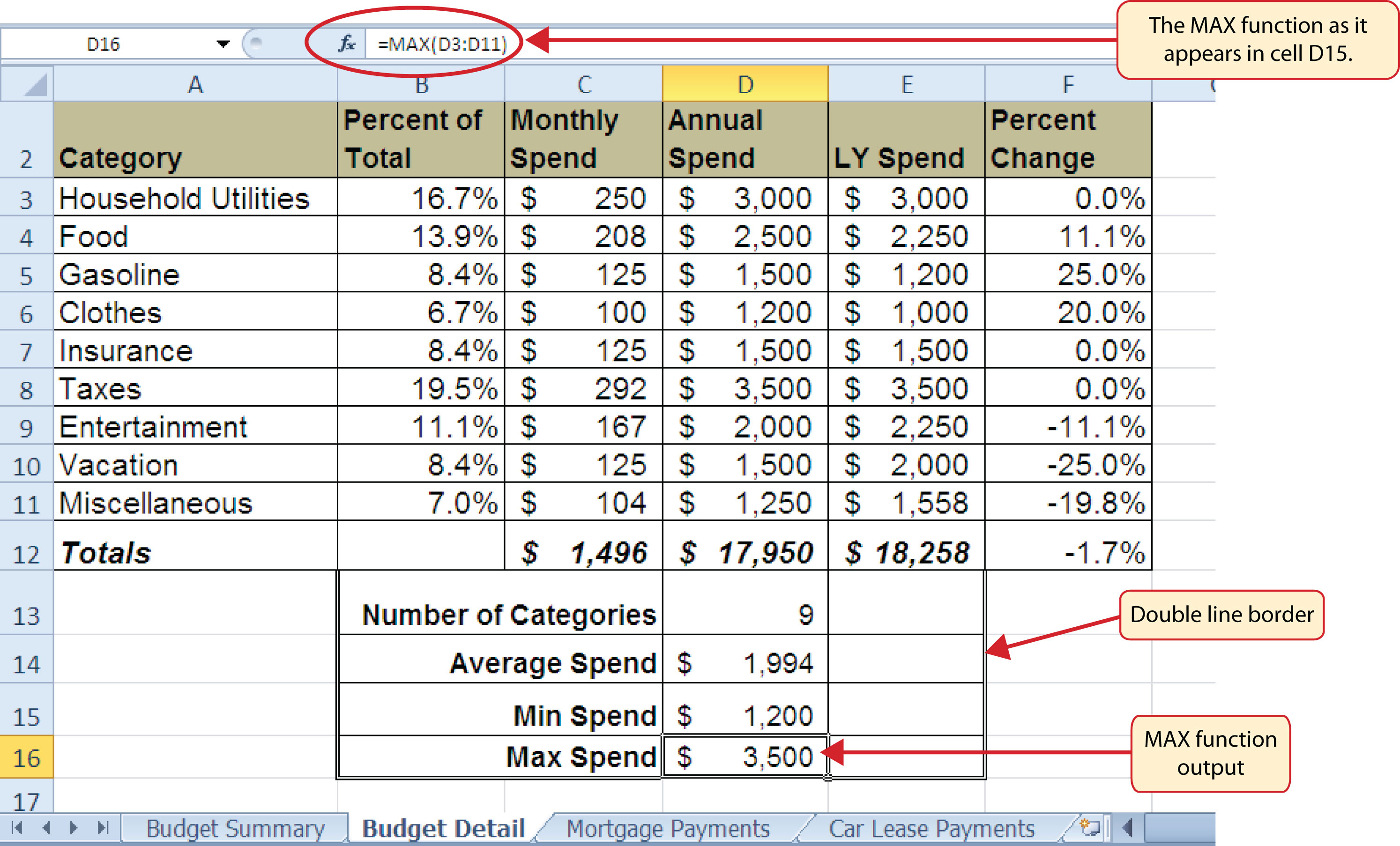### Skill Refresher: Statistical Functions

1. Type an equal sign (=).
2. Type the function name followed by an open parenthesis (() or double click the function name from the function list.
3. Highlight a range on a worksheet or click individual cell locations followed by commas.
4. Type a closing parenthesis ()).
5. Press the ENTER key.

## Copy and Paste Formulas (Pasting without Formats)

Follow-along file: Continue with Excel Objective 2.00. (Use file Excel Objective 2.08 if starting here.)

As shown in Figure 2.25 "MAX Function Added to the Budget Detail Worksheet", the COUNT, AVERAGE, MIN, and MAX functions are summarizing the data in the Annual Spend column. You will also notice that there is space to copy and paste these functions under the LY Spend column. This allows us to compare what we spent last year and what we are planning to spend this year. Normally, we would simply copy and paste these functions into the range E13:E16. However, you may have noticed the double-line style border that was used around the perimeter of the range B13:E16. If we used the regular Paste command, the double line on the right side of the range E13:E16 would be replaced with a single line. Therefore, we are going to use one of the Paste Special commands to paste only the functions without any of the formatting treatments. This is accomplished through the following steps:

1. Highlight the range D13:D16 in the Budget Detail worksheet.
2. Click the Copy button in the Home tab of the Ribbon.
3. Click cell E13.
4. Click the down arrow below the Paste button in the Home tab of the Ribbon.
5. Click the Formulas option from the drop-down list of buttons (see Figure 2.26 "Paste Formulas Option").

Figure 2.26 "Paste Formulas Option" shows the list of buttons that appear when you click the down arrow below the Paste button in the Home tab of the Ribbon. One thing to note about these options is that you can preview them before you make a selection by dragging the mouse pointer over the options. As shown in the figure, when the mouse pointer is placed over the Formulas button, you can see how the functions will appear before making a selection. Notice that the double-line border does not change when this option is previewed. That is why this selection is made instead of the regular Paste option.

Figure 2.26 Paste Formulas Option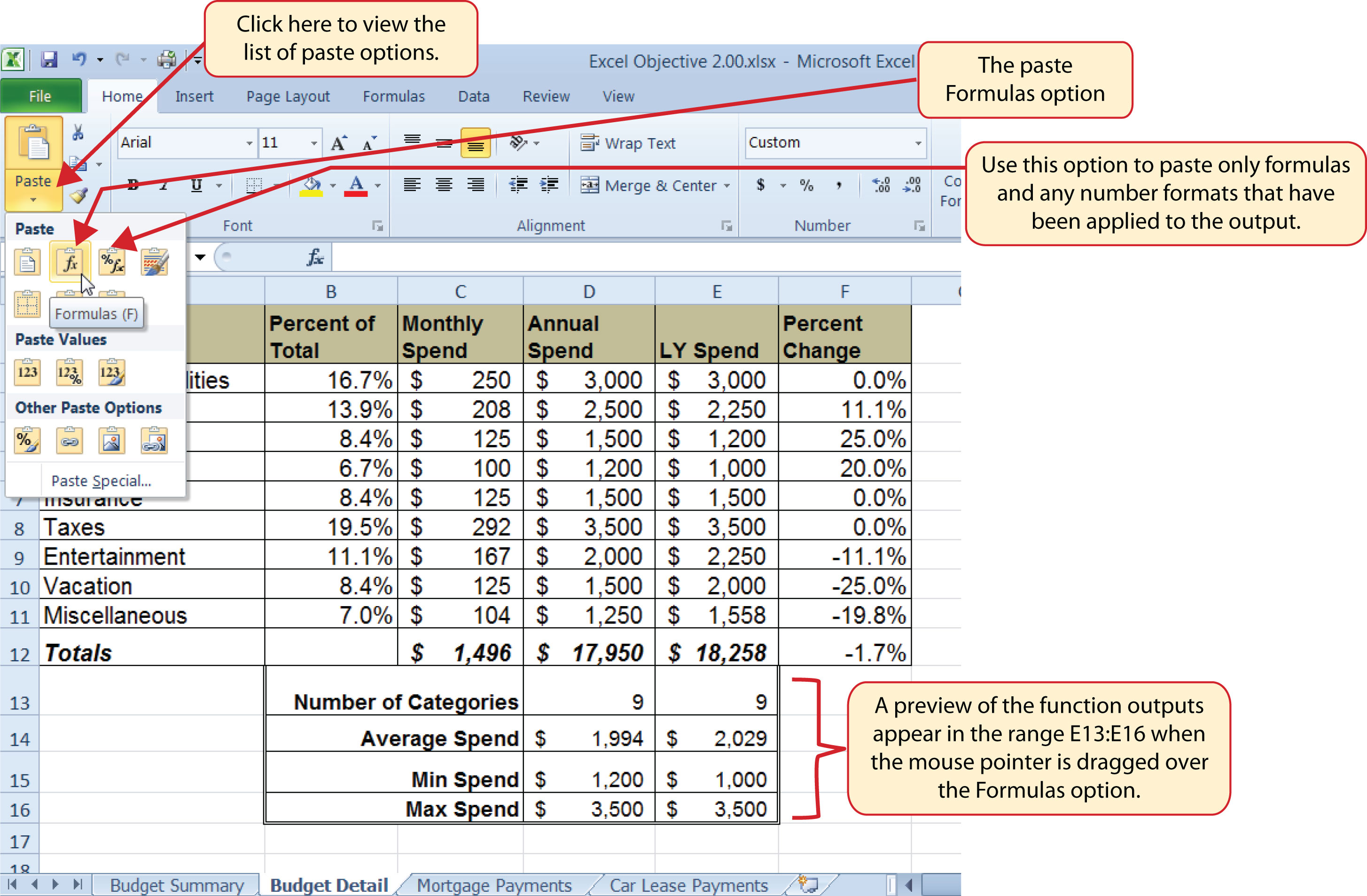### Skill Refresher: Paste Formulas

1. Click a cell location containing a formula or function.
2. Click the Copy button in the Home tab of the Ribbon.
3. Click the cell location or cell range where the formula or function will be pasted.
4. Click the down arrow below the Paste button in the Home tab of the Ribbon.
5. Click the Formulas button under the Paste group of buttons.

## Sorting Data (Multiple Levels)

Follow-along file: Continue with Excel Objective 2.00. (Use file Excel Objective 2.09 if starting here.)

The Budget Detail worksheet shown in Figure 2.26 "Paste Formulas Option" is now producing several mathematical outputs through formulas and functions. The outputs allow you to analyze the details and identify trends as to how money is being budgeted and spent. Before we draw some conclusions from this worksheet, we will sort the data based on the Percent of Total column. As mentioned in Chapter 1 "Fundamental Skills", sorting is a powerful tool that enables you to analyze key trends in any data set. We demonstrated the process of executing a single-level sort in Chapter 1 "Fundamental Skills". For the purposes of the Budget Detail worksheet, we need to set multiple levels for the sort order. This is accomplished through the following steps:

1. Highlight the range A2:F11 in the Budget Detail worksheet.
2. Click the Data tab in the Ribbon.
3. Click the Sort button in the Sort & Filter group of commands. This opens the Sort dialog box, as shown in Figure 2.27 "Sort Dialog Box".

Figure 2.27 Sort Dialog Box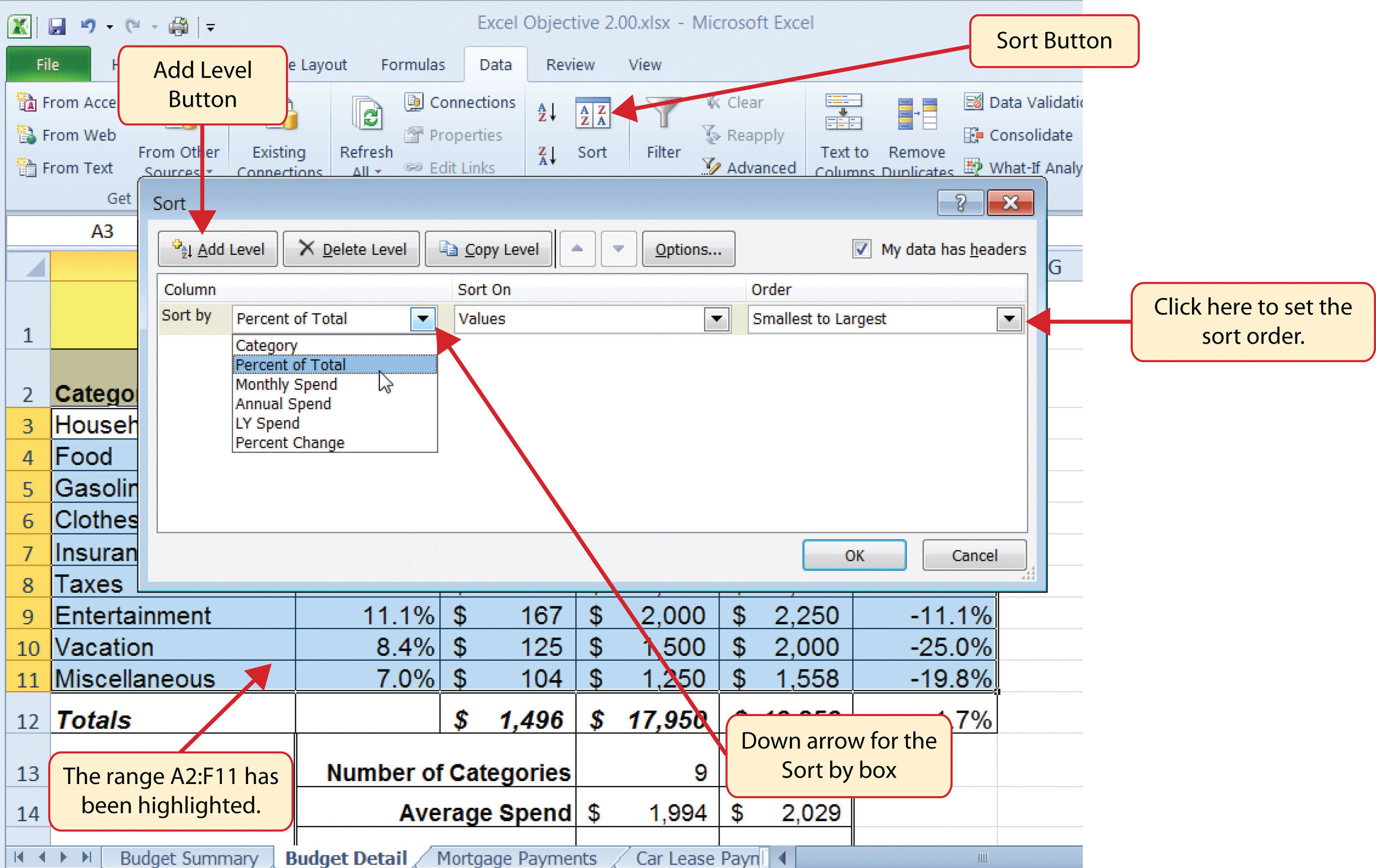4. Click the down arrow next to the “Sort by” box.
5. Click the Percent of Total option from the drop-down list.
6. Click the down arrow next to the sort Order box.
7. Click the Largest to Smallest option.
8. Click the Add Level button. This allows you to set a second level for any duplicate values in the Percent of Total column.
9. Click the down arrow next to the “Then by” box.
10. Select the LY Spend option.
11. Click the OK button at the bottom of the Sort dialog box.

Figure 2.28 "Budget Detail Worksheet after Sorting" shows the Budget Detail worksheet after it has been sorted. Notice that there are three identical values in the Percent of Total column. This is why a second sort level had to be created for this worksheet. The second sort level arranges the values of 8.4% based on the values in the LY Spend column in ascending order. Excel gives you the option to set as many sort levels as necessary for the data contained in a worksheet.

Figure 2.28 Budget Detail Worksheet after Sorting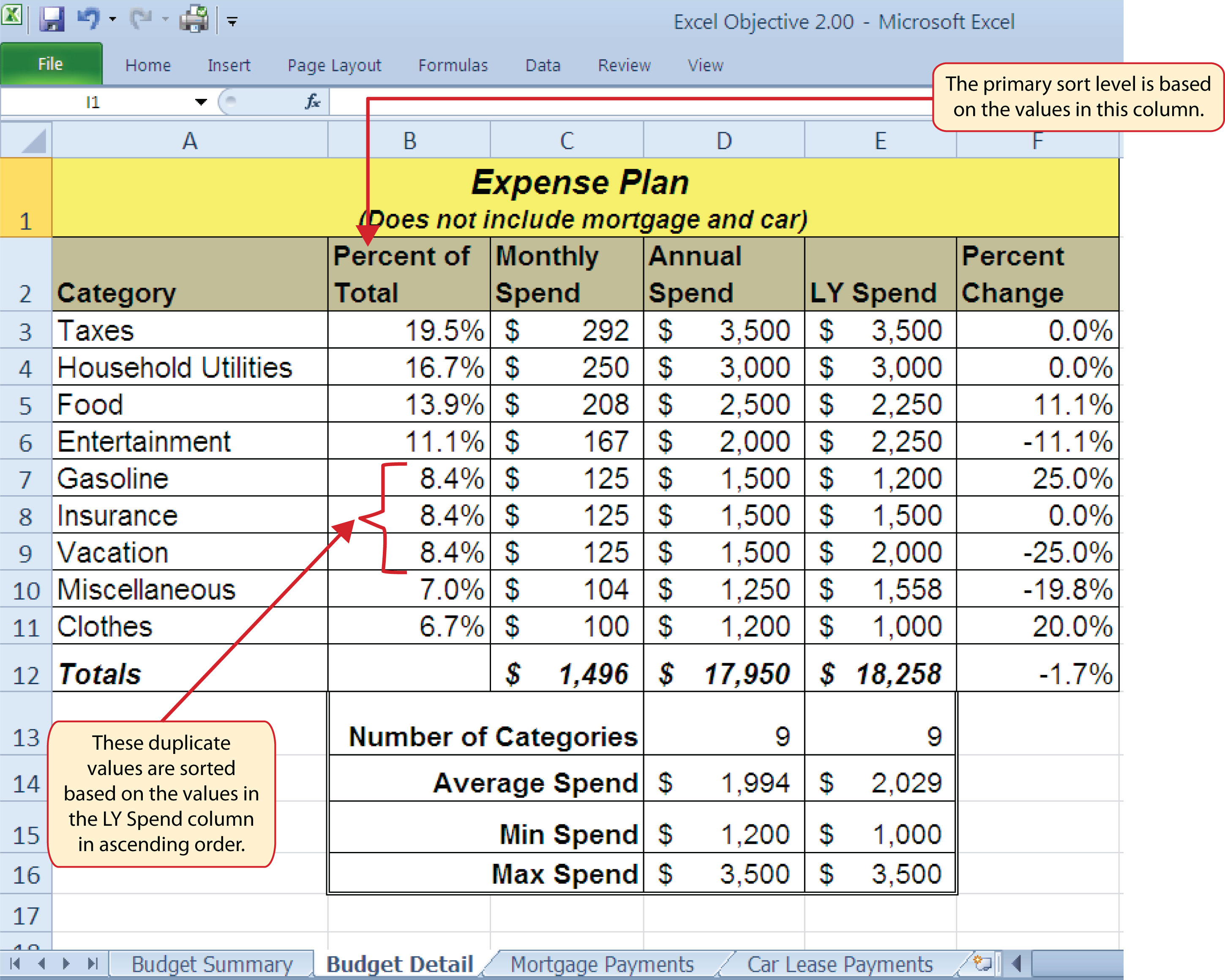### Skill Refresher: Sorting Data (Multiple Levels)

1. Highlight a range of cells to be sorted.
2. Click the Data tab of the Ribbon.
3. Click the Sort button in the Sort & Filter group.
4. Select a column from the “Sort by” drop-down list in the Sort dialog box.
5. Select a sort order from the Order drop-down list in the Sort dialog box.
6. Click the Add button in the Sort dialog box.
7. Repeat Steps 4 and 5.
8. Click the OK button on the Sort dialog box.

Now that the Budget Detail worksheet is sorted, a few key trends can be easily identified. The worksheet clearly shows that the top three categories as a percentage of total budgeted spending for the year are Taxes, Household Utilities, and Food. All three categories are necessities (or realities) of life and typically require a significant amount of income for most households. Looking at the Percent Change column, we can see how our planned spending is expected to change from last year. This is perhaps the most import column on the worksheet because it allows you to assess whether your plan is realistic. You will see that there are no changes planned for Taxes and Household Utilities. While Taxes can change from year to year, it is not too difficult to predict what they will be. In this case, we are assuming that there are no changes to the tax costs for our budget. We are also planning no change in Household Utilities. These costs can fluctuate from year to year as well. However, you can take measures to reduce costs, such as using less electricity, turning off heat when no one is in the house, keeping track of your wireless minutes so you do not go over the maximum allowed in your plan, and so on. As a result, there is no change in planned spending for Household Utilities because we will assume that any rate increases will be offset with a decrease in usage. The third item that is planned not to change is Insurance. Insurance policies for cars and homes can change, but as is true for taxes, the changes are predictable. Therefore, we are assuming no changes in our insurance policy.

The first big change that is noticeable in the worksheet is the Food and Entertainment categories in rows 5 and 6 (see definitions in Table 2.1 "Spend Category Definitions"). The Percent Change column indicates that there is an 11.1% decrease in Entertainment spending and an 11.1% increase in Food spending. This is logical because if you plan to eat in restaurants less frequently, you will be eating at home more frequently. Although this makes sense in theory, it may be hard to do in practice. Dinners and parties with friends may be tough to turn down. However, the entire process of maintaining a budget is based on discipline, and it certainly takes a significant amount of discipline to plan targets for yourself and stick to them.

A few other points to note are the changes in the Gasoline and Vacation categories. If you commute to school or work, the price of gas can have a significant impact on your budget. It is important to be realistic if gas prices are increasing, and you should reflect these increases in your budget. To compensate for the increased spending for gas, the spending plan for vacations has been reduced by 25%. Budgeting often requires a certain degree of creativity. Although the Vacation budget has been reduced, there is still money you can set aside to make plans for spring break or winter break.

Finally, the budget shows a decrease in Miscellaneous spending of 19.8%. This was defined as a group containing several expenses, such as textbooks, school supplies, software updates, and so on (see Table 2.1 "Spend Category Definitions"). You may be able to reduce your spending in this category if you can use items such as online textbooks. This reduction in spending can free up funds for Clothes, a spend category that has increased by 20%. We will continue to develop the Personal Budget workbook further in Section 2.3 "Functions for Personal Finance".

### Key Takeaways

• Statistical functions are used when a mathematical process is required for a range of cells, such as summing the values in several cell locations. For these computations, functions are preferable to formulas because adding many cell locations one at a time to a formula can be very time-consuming.
• Statistical functions can be created using cell ranges or selected cell locations separated by commas. Make sure you use a cell range (two cell locations separated by a colon) when applying a statistical function to a contiguous range of cells.
• To prevent Excel from changing the cell references in a formula or function when they are pasted to a new cell location, you must use an absolute reference. You can do this by placing a dollar sign (\$) in front of the column letter and row number of a cell reference.
• The #DIV/0 error appears if you create a formula that attempts to divide a constant or the value in a cell reference by zero.
• The Paste Formulas option is used when you need to paste formulas without any formatting treatments into cell locations that have already been formatted.
• You need to set multiple levels, or columns, in the Sort dialog box when sorting data that contains several duplicate values.

### Exercises

1. In the formula =C2/\$C\$24, the dollar signs used in the C24 cell reference indicate:

1. Relative referencing has been turned off for this cell reference.
2. Any value entered into cell C24 will be formatted with US currency.
3. The output of the formula will be formatted with US currency.
4. The value currently in C24 cannot be changed.
2. Which statement best explains how the following function will produce a result =AVERAGE(B1,B10)?

1. The function will calculate the average for the values in B1 and B10.
2. The function will calculate the average for the values in all cell locations in the range B1 through and including B10.
3. Commas cannot be used in statistical functions. The function cannot produce an output and will display the #DIV/0 error code.
4. The function will take the average of cell B1 based on the value that is entered into cell B10.
3. Which of the following best explains the purpose of the Paste Formulas command?

1. You cannot use the regular Paste command for formulas and functions. You need to use the Paste Formulas command when you copy and paste a formula or function to additional cell locations on a worksheet.
2. You would use the Paste Formulas command when you want to show the formula or function in a cell location and not the calculated output.
3. You would use the Paste Formulas command when you want to paste only the formula or function to a new cell location without the formatting treatments that were applied to the copied cell location.
4. The Paste Formulas command is required if you want to paste a formula into multiple cell locations on a worksheet.
4. Which of the following explains how data in a worksheet can be sorted if the primary column of data contains several duplicate values?

1. You can only sort data based on the values in one column. Excel will take the duplicate values and sort them in the order in which they were entered into the worksheet.
2. Excel will sort any duplicate values in the primary column based on the values one column to the right in ascending order.
3. You can click the Add button in the Sort dialog box and designate which column Excel should use to sort any duplicate values in the primary column.
4. You would sort each column in the worksheet one at a time.

## 2.3 Functions for Personal Finance

### Learning Objectives

1. Understand the fundamentals of loans and leases.
2. Use the PMT function to calculate monthly mortgage payments on a house.
3. Use the PMT function to calculate monthly lease payments for an automobile.
4. Learn how to summarize data in a workbook by using worksheet links to create a summary worksheet.
5. Understand the concept of the time value of money.
6. Use the FV function to calculate the future value of personal investments.
7. Use Goal Seek to conduct what-if scenarios.

In this section, we continue to develop the Personal Budget workbook. Notable items that are missing from the Budget Detail worksheet are the payments you might make for a car or a home. In addition, you may want to set and track a savings goal. This section demonstrates Excel functions used to calculate lease payments for a car, to calculate mortgage payments for a house, and to project future savings based on regular contributions and an average rate of return. This section also discusses the scenario capabilities of Excel once the Personal Budget workbook is complete.

## The Fundamentals of Loans and Leases

Follow-along file: Continue with Excel Objective 2.00. (Use file Excel Objective 2.10 if starting here.)

One of the functions we will add to the Personal Budget workbook is the PMT function. This function calculates the payments required for a loan or a lease. However, before demonstrating this function, it is important to cover a few fundamental concepts on loans and leases.

A loanA contractual agreement in which money is borrowed from a lender and paid back over a specific period of time. is a contractual agreement in which money is borrowed from a lender and paid back over a specific period of time. The amount of money that is borrowed from the lender is called the principalThe amount of money borrowed from a lender. of the loan. The borrower is usually required to pay the principal of the loan plus interest. When you borrow money to buy a house, the loan is referred to as a mortgageA loan used to purchase a home or property.. This is because the house being purchased also serves as collateral to ensure payment. In other words, the bank can take possession of your house if you fail to make loan payments. As shown in Table 2.5 "Key Terms for Loans and Leases", there are several key terms related to loans and leases.

Table 2.5 Key Terms for Loans and Leases

Term Definition
Collateral Any item of value that is used to secure a loan to ensure payments to the lender
Down Payment The amount of cash paid toward the purchase of a house. If you are paying 20% down, you are paying 20% of the cost of the house in cash and are borrowing the rest from a lender.
Interest Rate The interest that is charged to the borrower as a cost for borrowing money
Mortgage A loan where property is put up for collateral
Principal The amount of money that has been borrowed
Residual Value The estimated selling price of a vehicle at a future point in time
Terms The amount of time you have to repay a loan

Figure 2.29 "Example of an Amortization Table" shows an example of an amortization tableA schedule of payments broken down by interest and principal for a loan. By law, a lender is required to provide an amortization table to a borrower. for a loan. A lender is required by law to provide borrowers with an amortization table when a loan contract is offered. The table in the figure shows how the payments of a loan would work if you borrowed \$100,000 from a lender and agreed to pay it back over 10 years at an interest rate of 5%. You will notice that each time you make a payment, you are paying the bank an interest fee plus some of the loan principal. Each year the amount of interest paid to the bank decreases and the amount of money used to pay off the principal increases. This is because the bank is charging you interest on the amount of principal that has not been paid. As you pay off the principal, the interest rate is applied to a lower number, which reduces your interest charges. Finally, the figure shows that the sum of the values in the Interest Payment column is \$29,505. This is how much it costs you to borrow this money over 10 years. Indeed, borrowing money is not free. It is important to note that to simplify this example, the payments were calculated on an annual basis. However, most loan payments are made on a monthly basis.

Figure 2.29 Example of an Amortization TableA leaseA contract in which the lessee uses an asset such as a car or a piece of equipment and agrees to make regular payments to the owner or the lessor. The lessee is often required to return the leased asset to the lessor at the conclusion of the lease contract. is a contract in which you, the lessee, use an asset such as a car or a piece of equipment and you agree to make regular payments to the owner or the lessor. When you lease a car, the manufacturer or a leasing company retains ownership of the vehicle and you agree to make regular payments for a specific period of time. The amount of money you pay depends on the price of the car, the terms of the lease contract, and the car’s expected residual value at the end of the lease. The calculation of lease payments is similar to the calculation of loan payments. However, when you lease a car, you pay only the value of the car that is used. For example, suppose you are leasing a car that is priced at \$25,000. The lease contract is for 4 years at an interest rate of 5%. The residual value of the car is \$10,000. This means the car will lose \$15,000 of its value over 4 years. Another way to state this is that the car will depreciate \$15,000. A lease will be structured so that you pay this \$15,000 in depreciation. However, the interest charges will be based on the purchase price of \$25,000. We will look at a demonstration of leasing a car as well as buying a home in the next section.

## The PMT (Payment) Function for Loans

Follow-along file: Continue with Excel Objective 2.00. (Use file Excel Objective 2.10 if starting here.)

If you own a home, your mortgage payments are a major component of your household budget. If you are planning to buy a home, having a clear understanding of your monthly payments is critical for maintaining strong financial health. In Excel, mortgage payments are conveniently calculated through the PMT (payment) function. This function is more complex than the statistical functions covered in Section 2.2 "Statistical Functions". With statistical functions, you are required to add only a range of cells or selected cells within the parentheses of the function. With the PMT function, you must accurately define a series of arguments in order for the function to produce a reliable output. Table 2.6 "Arguments for the PMT Function" lists the arguments for the PMT function. It is helpful to review the key loan and lease terms in Table 2.5 "Key Terms for Loans and Leases" before reviewing the PMT function arguments.

Table 2.6 Arguments for the PMT Function

Argument Definition
Rate This is the interest rate the lender is charging the borrower. The interest rate is usually quoted in annual terms, so you have to divide this rate by 12 if you are calculating monthly payments.
Nper The argument letters stand for number of periods. This is the term of the loan, which is the amount of time you have to repay the bank. This is usually quoted in years, so you have to multiply the years by 12 if you are calculating monthly payments.
Pv The argument letters stand for present value. This is the principal of the loan or the amount of money that is borrowed. When defining this argument, a minus sign must precede the cell location or value. For leases, this argument is used for the price of the item being leased.
[Fv] The argument letters stand for future value. The brackets around the argument indicate that it is not always necessary to define it. It is used if there is a lump-sum payment that will be made at the end of the loan terms. This is also used for the residual value of a lease. If it is not defined, Excel will assume that it is zero.
[Type] This argument can be defined with either a 1 or a 0. The number 1 is used if payments are made at the beginning of each period. A 0 is used if payments are made at the end of each period. The argument is in brackets because it does not have to be defined if payments are made at the end of each period. Excel assumes that this argument is 0 if it is not defined.

We will use the PMT function in the Personal Budget workbook to calculate the monthly mortgage payments for a house. These calculations will be made in the Mortgage Payments worksheet and then displayed in the Budget Summary worksheet through a cell reference link. So far we have demonstrated several methods for adding functions to a worksheet. The following steps explain a new method using the Insert Function command for adding the PMT function:

1. Click the Mortgage Payments worksheet tab.
2. Click cell B5.
3. Click the Formulas tab on the Ribbon.
4. Click the Insert Function button (see Figure 2.30 "Mortgage Payments Worksheet"). This opens the Insert Function dialog box, which can be used for searching all functions in Excel.

Figure 2.30 Mortgage Payments Worksheet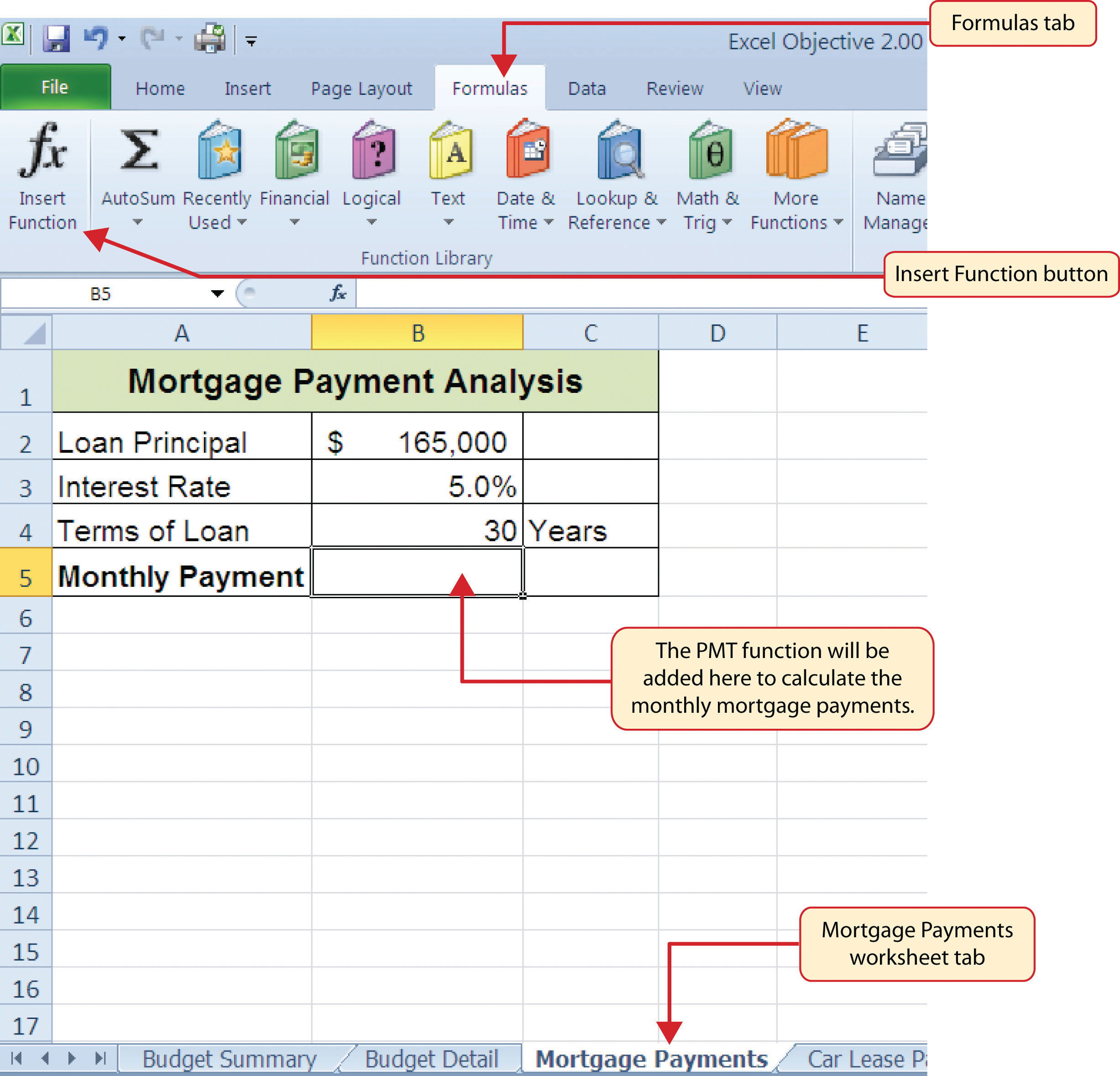5. In the “Search for a function:” input box at the top of the Insert Function dialog box, type mortgage payments (see Figure 2.31 "Insert Function Dialog Box"). Note that the current description in the “Search for a function:” input box will already be highlighted. You can begin typing and the description will be replaced with your entry.
6. Click the Go button in the upper right side of the Insert Function dialog box. This adds all the Excel functions that match your description in the “Select a function:” box in the lower half of the Insert Function dialog box (see Figure 2.31 "Insert Function Dialog Box").
7. Click the PMT option in the “Select a function:” box in the lower half of the Insert Function dialog box.
8. Click the OK button at the lower right side of the Insert Function dialog box. This will open the Function Arguments dialog box.

Figure 2.31 Insert Function Dialog Box### Mouseless Commands

Insert Function

• Hold the SHIFT key while pressing the F3 key.
9. Click the Collapse Dialog button next to the Rate argument in the Function Arguments dialog box. This will be the first argument defined for the function.
10. Click cell B3 on the worksheet. This is the rate being charged on the loan.
11. Type a forward slash (/) for division.
12. Type the number 12. Since our goal is to calculate the monthly payments for the loan, we need to divide the rate, which is stated in annual terms, by 12. This converts the annual rate to a monthly rate.
13. Press the ENTER key on your keyboard. This returns the Function Arguments dialog box to its expanded form. You will also see that the Rate argument is now defined.
14. Click the Collapse Dialog button next to the Nper argument in the Function Arguments dialog box. This is the second argument we define in the function.
15. Click cell B4 on the worksheet. This is the term or the amount of time we have to repay the loan.
16. Type an asterisk (*) for multiplication.
17. Type the number 12. Since our goal is to calculate the monthly payments for the loan, we need to multiply the terms of the loan by 12. This converts the terms of the loan from years to months.
18. Press the ENTER key on your keyboard. This returns the Function Arguments dialog box to its expanded form. You will also see that the Nper argument is now defined.
19. Click the Collapse Dialog button next to the Pv argument in the Function Arguments dialog box. This is the third argument we will define in the function.
20. Type a minus sign (). When defining the Pv argument of the PMT function, any cell location or value must be preceded with a minus sign.
21. Click cell B2 on the worksheet. This is the principal of the loan.
22. Press the ENTER key on your keyboard. You will now see the Rate, Nper, and Pv arguments defined for the function.
23. Click the OK button at the bottom of the Function Arguments dialog box. The function will now be placed into the worksheet. Since we are not paying any lump sums of money at the end of the loan, there is no need to define the Fv argument. Also, we will assume that the monthly mortgage payments will be made at the end of each month. Therefore, there is no need to define the Type argument.

### Mouseless Commands

Function Arguments Dialog Box

• After the equal sign (=) and function name are typed into cell a location, hold down the CTRL key and press the letter A on your keyboard.

Figure 2.32 "Function Arguments Dialog Box for the PMT Function" shows the completed Function Arguments dialog box for the PMT function. Notice that the dialog box shows the values for the Rate and Nper arguments. The Rate is divided by 12 to convert the annual interest rate to a monthly interest rate. The Nper argument is multiplied by 12 to convert the terms of the loan from years to months. Finally, the dialog box provides you with a definition for each argument. The definition appears when you click in the input box for the argument.

Figure 2.32 Function Arguments Dialog Box for the PMT Function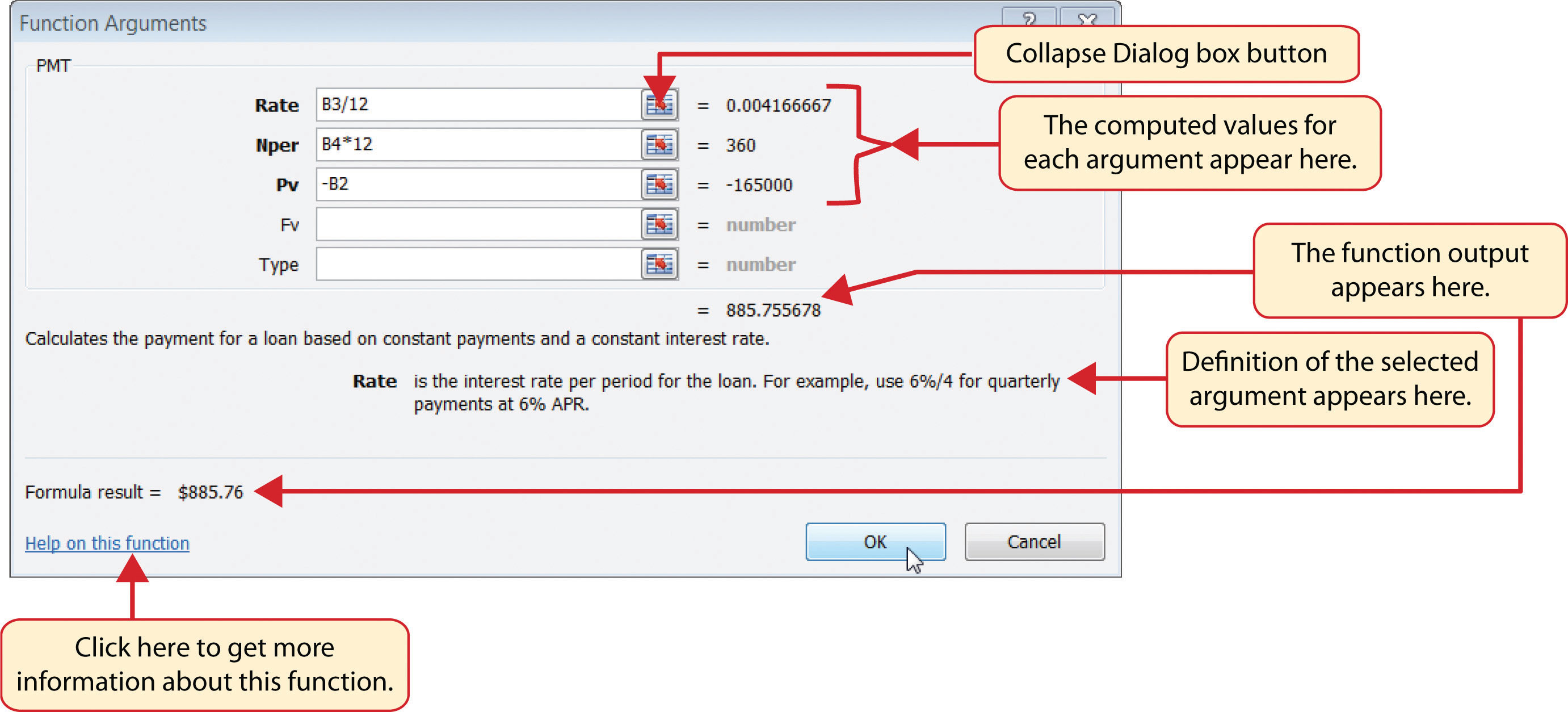### Integrity Check

Comparable Arguments for PMT and FV Functions

When using functions such as PMT or FV, make sure the arguments are defined in comparable terms. For example, if you are calculating the monthly payments of a loan, make sure both the Rate and Nper argument are expressed in terms of months. The function will produce an erroneous result if one argument is expressed in years while the other is expressed in months.

Figure 2.33 "Mortgage Payments Worksheet with the PMT Function" shows the final appearance of the Mortgage Payments worksheet after the PMT function is added. The result of the function in cell B5 will be displayed in the Budget Summary worksheet.

Figure 2.33 Mortgage Payments Worksheet with the PMT Function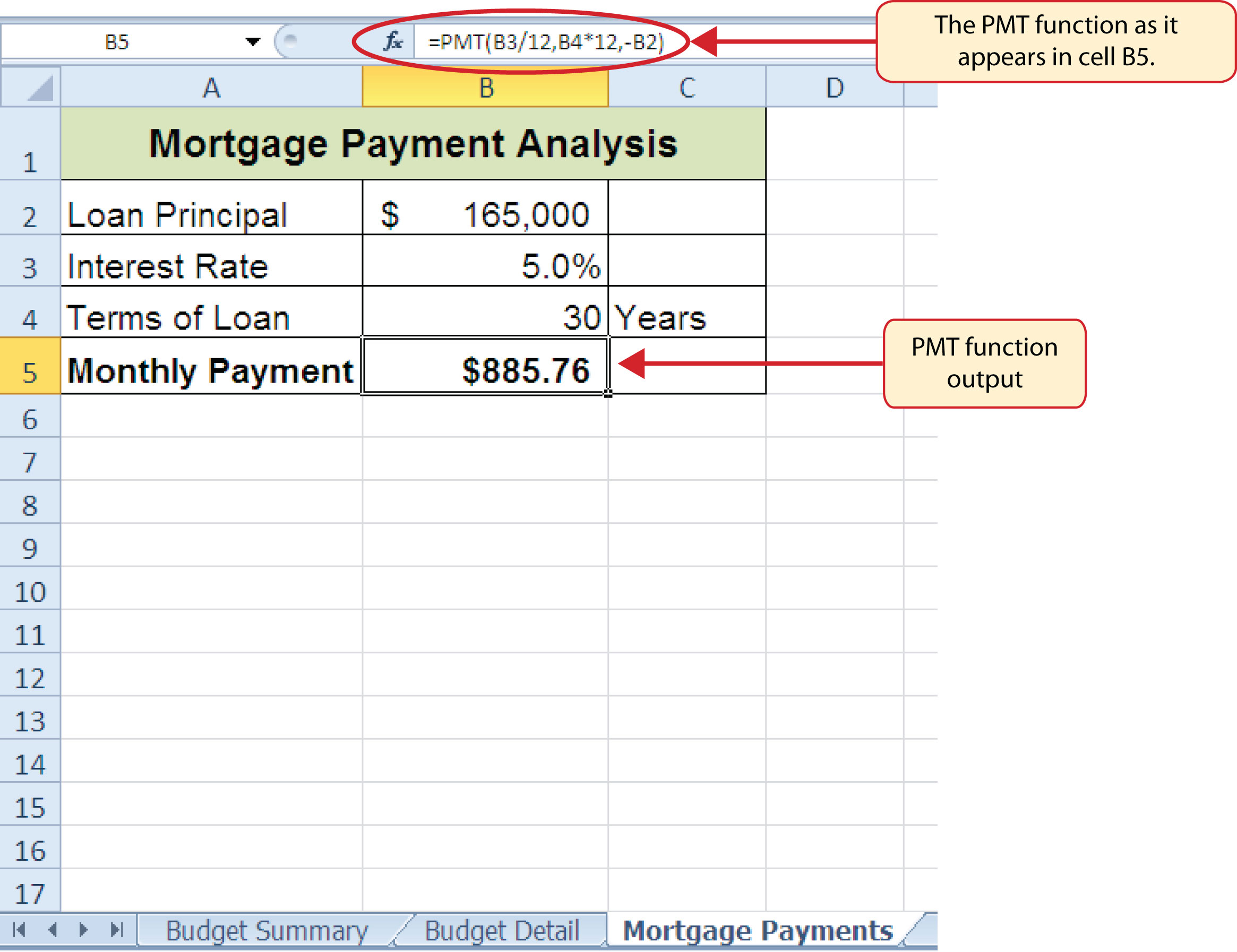## The PMT (Payment) Function for Leases

Follow-along file: Continue with Excel Objective 2.00. (Use file Excel Objective 2.11 if starting here.)

In addition to calculating the mortgage payments for a home, the PMT function will be used in the Personal Budget workbook to calculate the lease payments for a car. The details for the lease payments are found in the Car Lease Payments worksheet. Similar to the statistical functions, we can type the PMT function directly into a cell. However, you must know the definitions for each argument of the function and understand how these arguments need to be defined based on your objective. The terms for loans and leases are in Table 2.5 "Key Terms for Loans and Leases", and the definitions for the arguments of the PMT function are in Table 2.6 "Arguments for the PMT Function". The following steps explain how the PMT function is added to the Personal Budget workbook to calculate the lease payments for a car:

1. Click cell B6 in the Car Lease Payments worksheet.
2. Type an equal sign (=).
3. Type the letters PMT.
4. Type an open parenthesis (().Excel then provides a tip box showing the arguments of the function.
5. Click cell B4. This is the interest rate being charged for the lease.
6. Type the forward slash (/) for division.
7. Type the number 12. Since our goal is to calculate the monthly lease payments, we divide the interest rate by 12 to convert the annual rate to a monthly rate.
8. Type a comma. When you type a function containing arguments, you must separate each argument with a comma. This signals to Excel that one argument has been defined and you are ready to define the next argument in the function.
9. Click cell B5. This is the term or the length of time for the lease contract. Since the term is already expressed in months, we can just reference cell B5 and move to the next argument.
10. Type a comma. This advances the function to the Pv argument.
11. Type a minus sign (). Remember that cell locations or values used to define the Pv argument must be preceded with a minus sign.
12. Click cell B2 on the worksheet, which is the price of the car.
13. Type a comma. This advances the function to the [Fv] argument.
14. Click cell B3 on the worksheet. This is the residual value of the car. Note that cell location and values used to define the [Fv] argument are NOT preceded by a minus sign.
15. Type a comma. This advances the function to the [Type] argument.
16. Type the number 1. We will assume that the lease payments will be due at the beginning of each month.
17. Type a closing parenthesis ()).
18. Press the ENTER key.

Figure 2.34 "PMT Function Constructed to Calculate Lease Payments" shows how the PMT function should appear before pressing the ENTER key. Notice the commas that separate each argument of the function. Also, the tip box will show the current argument being defined in bold font.

Figure 2.34 PMT Function Constructed to Calculate Lease Payments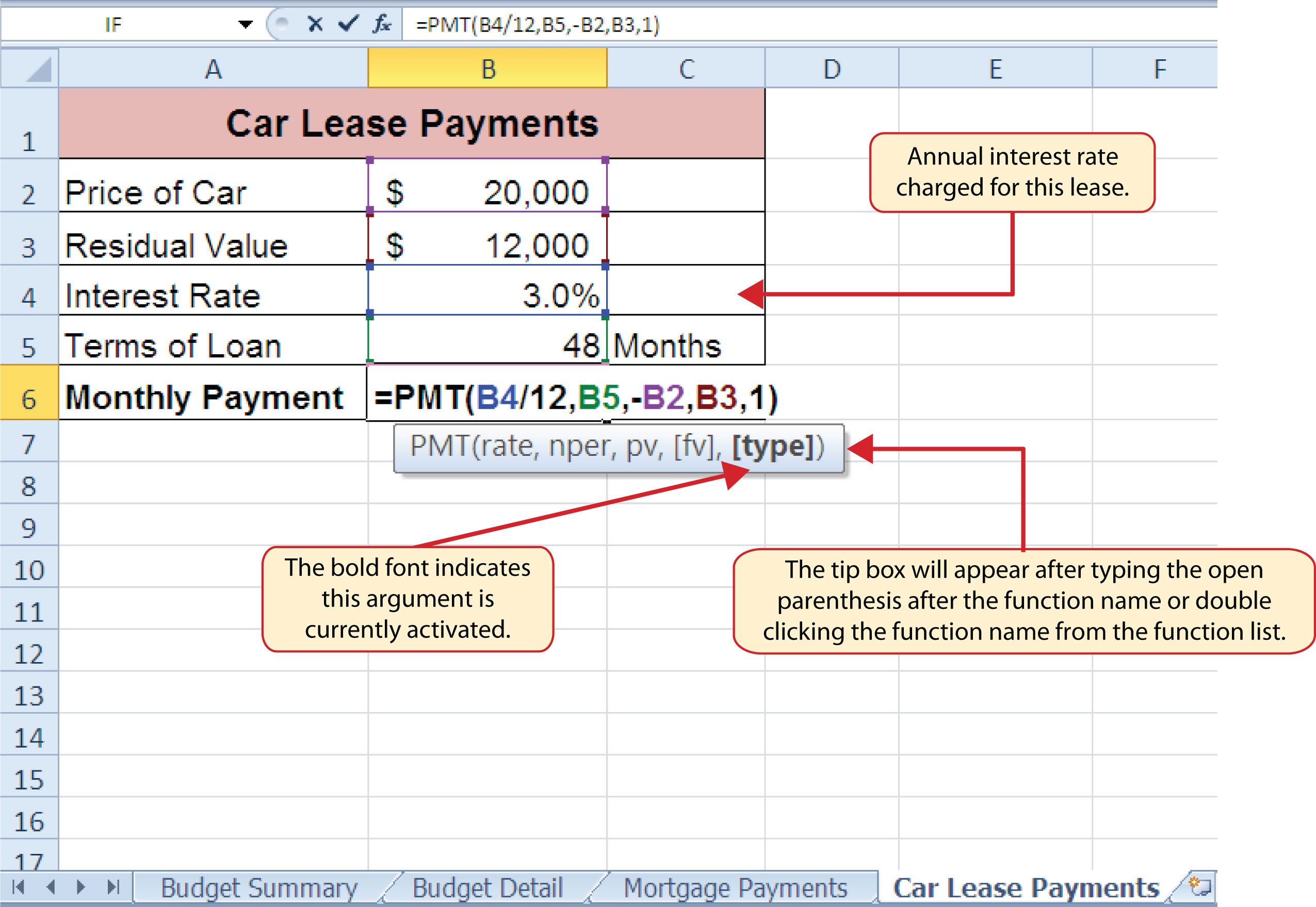Figure 2.35 "Results of the PMT Function in the Car Lease Payments Worksheet" shows the result of the PMT function. The monthly payments for this lease are \$206.56. This monthly payment will be displayed in the Budget Summary worksheet.

Figure 2.35 Results of the PMT Function in the Car Lease Payments Worksheet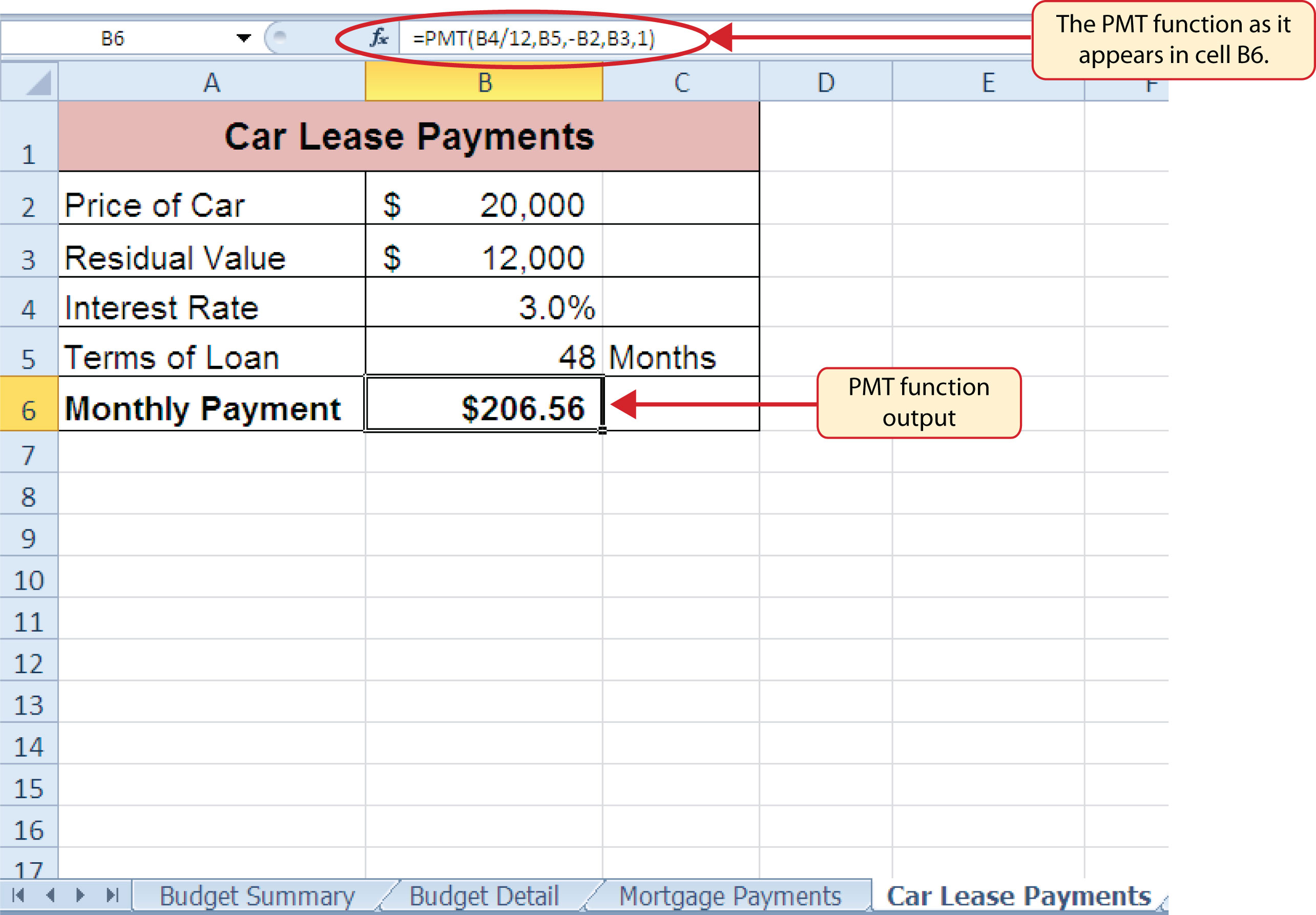### Skill Refresher: PMT Function

1. Type an equal sign (=).
2. Type the letters PMT followed by an open parenthesis, or double click the function name from the function list.
3. Define the Rate argument with a cell location that contains the rate being charged by the lender for the loan or lease.
4. Define the Nper argument with a cell location that contains the amount of time to repay the loan or lease.
5. Define the Pv argument with a cell location that contains the principal of the loan or the price of the item being leased. Cell locations or values used for this argument must be preceded by a minus sign.
6. Define the [Fv] argument with a cell location that contains the residual value of the item being leased or the lump sum payment for a loan.
7. Define the [Type] argument with a 1 if payments are made at the beginning of each period or 0 if payments are made at the end of each period.
8. Type a closing parenthesis ()).
9. Press the ENTER key.

## Linking Worksheets (Creating a Summary Worksheet)

Follow-along file: Continue with Excel Objective 2.00. (Use file Excel Objective 2.12 if starting here.)

So far we have used cell references in formulas and functions, which allow Excel to produce new outputs when the values in the cell references are changed. Cell references can also be used to display values or the outputs of formulas and functions in cell locations on other worksheets. This is how data will be displayed on the Budget Summary worksheet in the Personal Budget workbook. Outputs from the formulas and functions that were entered into the Budget Detail, Mortgage Payments, and Car Lease Payments worksheets will be displayed on the Budget Summary worksheet through the use of cell references. The following steps explain how this is accomplished:

1. Click cell C3 in the Budget Summary worksheet.
2. Type an equal sign (=).
3. Click the Budget Detail worksheet tab.
4. Click cell D12 on the Budget Detail worksheet.
5. Press the ENTER key on your keyboard. The output of the SUM function in cell D12 on the Budget Detail worksheet will be displayed in cell C3 on the Budget Summary worksheet.

Figure 2.36 "Cell Reference Showing the Total Expenses in the Budget Summary Worksheet" shows how the cell reference appears in the Budget Summary worksheet. Notice that the cell reference D12 is preceded by the Budget Detail worksheet name enclosed in apostrophes followed by an exclamation point (‘Budget Detail’!) This indicates that the value displayed in the cell is referencing a cell location in the Budget Detail worksheet.

Figure 2.36 Cell Reference Showing the Total Expenses in the Budget Summary WorksheetAs shown in Figure 2.36 "Cell Reference Showing the Total Expenses in the Budget Summary Worksheet", the Budget Summary worksheet is designed to show the expense budget for the mortgage payments and the auto lease payments. However, you will recall that we used the PMT function to calculate the monthly payments. In the Budget Summary worksheet, we need to show the total annual payments. As a result, we will create a formula that references cell locations in the Mortgage Payments and Car Lease Payments worksheets. The following steps explain how this is accomplished:

1. Click cell C4 in the Budget Summary worksheet.
2. Type an equal sign (=).
3. Click the Mortgage Payments worksheet tab.
4. Click cell B5 in the Mortgage Payments worksheet.
5. Type an asterisk (*) for multiplication.
6. Type the number 12. This multiplies the monthly payments by 12 to calculate the total payments required for the year.
7. Press the ENTER key on your keyboard. The value of multiplying the monthly mortgage payments by 12 is now displayed on the Budget Summary worksheet.
8. Click cell C5 on the Budget Summary worksheet.
9. Type an equal sign (=).
10. Click the Car Lease Payments worksheet tab.
11. Click cell B6 in the Car Lease Payments worksheet.
12. Type an asterisk (*) for multiplication.
13. Type the number 12. This multiplies the monthly lease payments by 12 to calculate the total payments required for the year.
14. Press the ENTER key on your keyboard. The value of multiplying the monthly lease payments by 12 is now displayed on the Budget Summary worksheet.

Figure 2.37 "Formulas Referencing Cells in Mortgage Payments and Car Lease Payments Worksheets" shows the results of creating formulas that reference cell locations in the Mortgage Payments and Car Lease Payments worksheets.

Figure 2.37 Formulas Referencing Cells in Mortgage Payments and Car Lease Payments Worksheets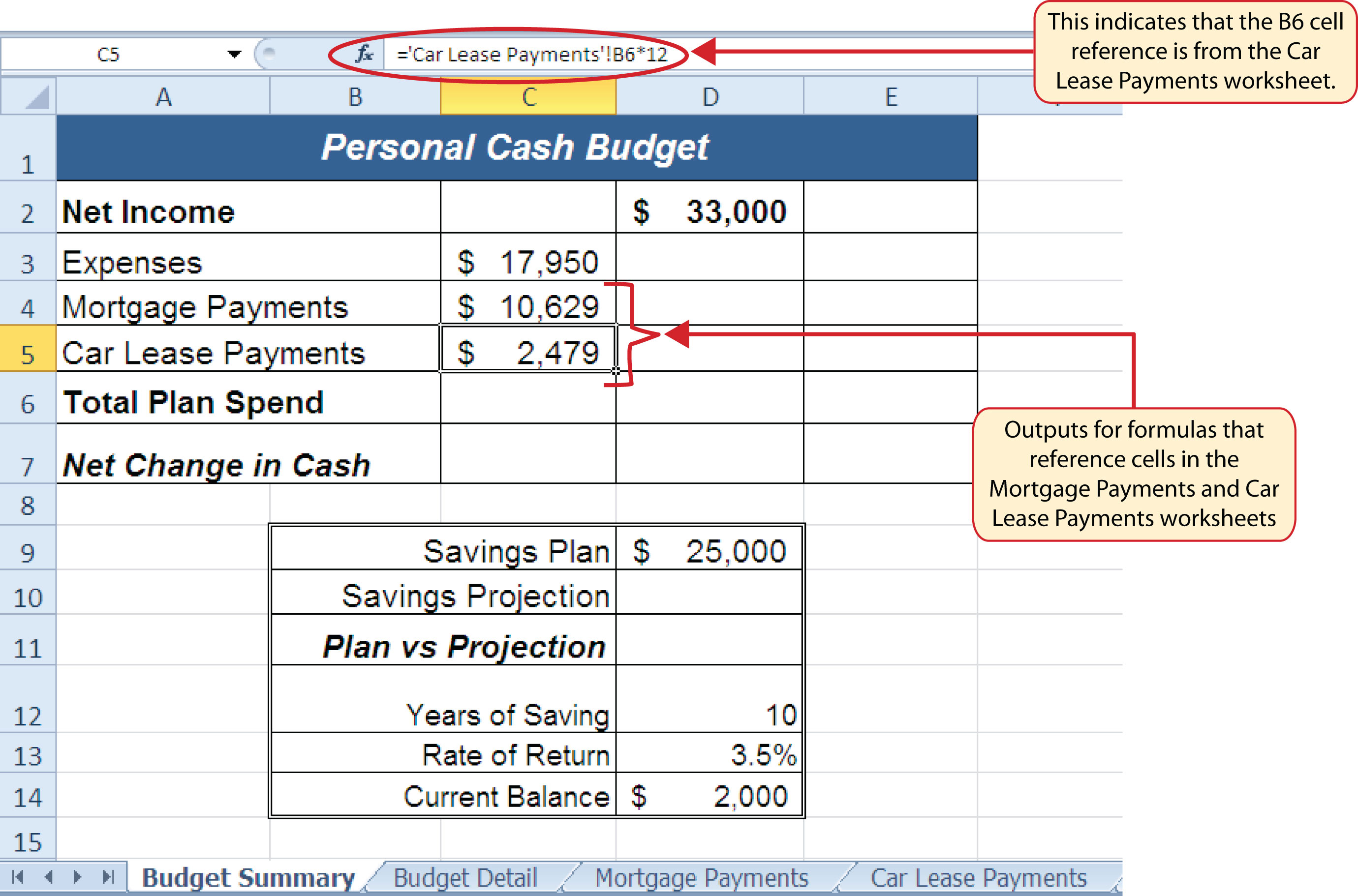We can now add other formulas and functions to the Budget Summary worksheet that can calculate the difference between the total spend dollars vs. the total net income in cell D2. The following steps explain how this is accomplished:

1. Click cell D6 in the Budget Summary worksheet.
2. Type an equal sign (=).
3. Type the function name SUM followed by an open parenthesis (().
4. Highlight the range C3:C5.
5. Type a closing parenthesis ()) and press the ENTER key on your keyboard. The total for all annual expenses now appears on the worksheet.
6. Click cell D7 on the Budget Summary worksheet.
7. Type an equal sign (=).
8. Click cell D2.
9. Type a minus sign () and then click cell D6.
10. Press the ENTER key on your keyboard. This formula produces an output of \$1,942, indicating our income is greater than our total expenses.

Figure 2.38 "Formulas Added to Show Income Is Greater Than Expenses" shows the results of the formulas that were added to the Budget Summary worksheet. The output for the formula in cell D7 shows that the net income exceeds total planned expenses by \$1,942. Overall, having your income exceed your total expenses is a good thing because it allows you to save money for future spending needs or unexpected events.

Figure 2.38 Formulas Added to Show Income Is Greater Than Expenses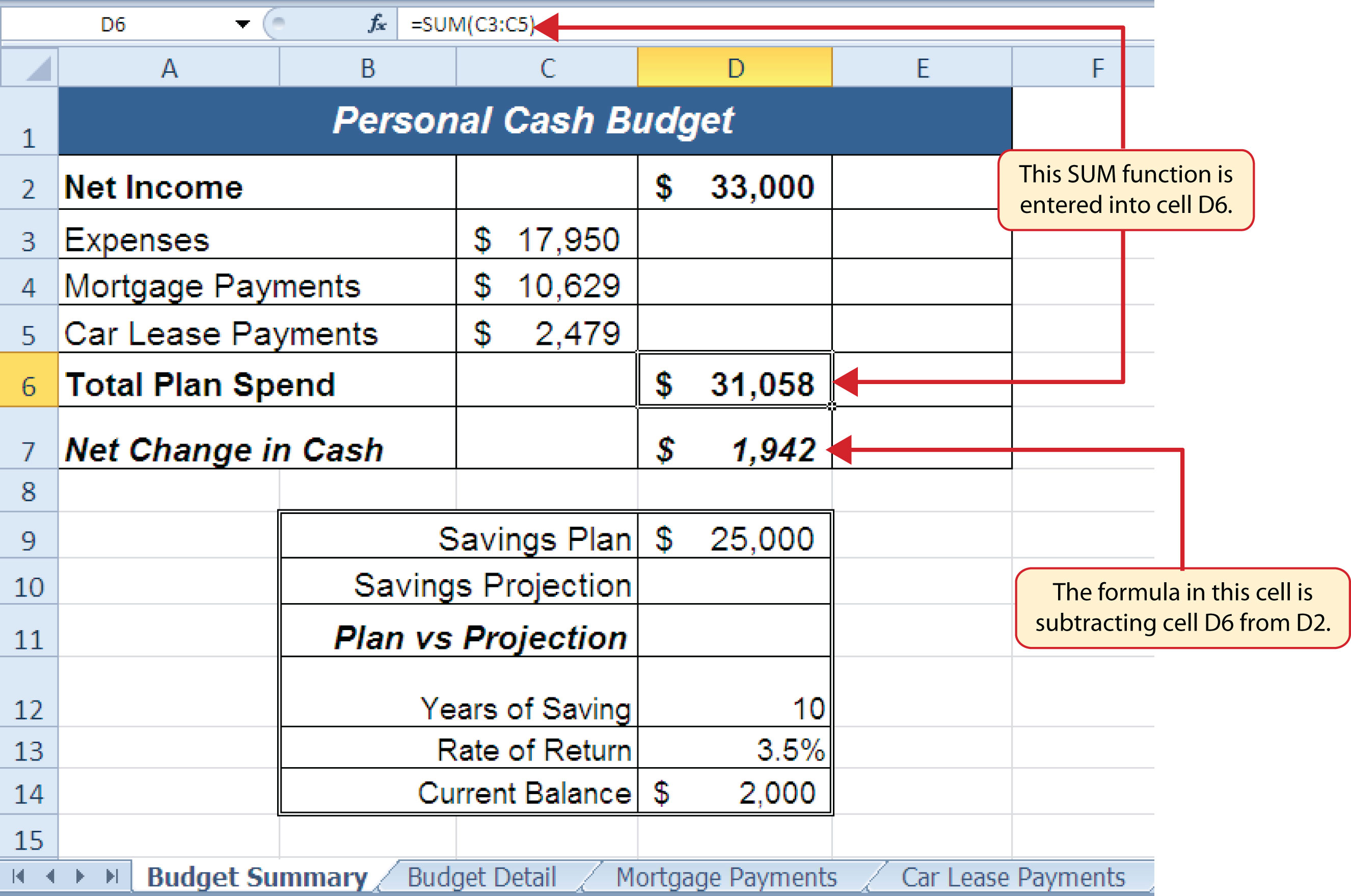We can now add a few formulas that calculate both the spending rate and the savings rate as a percentage of net income. These formulas require the use of absolute references, which we covered earlier in this chapter. The following steps explain how to add these formulas:

1. Click cell E6 in the Budget Summary worksheet.
2. Type an equal sign (=).
3. Click cell D6.
4. Type a forward slash (/) for division and then click D2.
5. Press the F4 key on your keyboard. This adds an absolute reference to cell D2.
6. Press the ENTER key. The result of the formula shows that total expenses consume 94.1% of our net income.
7. Click cell E6.
8. Place the mouse pointer over the Auto Fill Handle.
9. When the mouse pointer turns to a black plus sign, left click and drag down to cell E7. This copies and pastes the formula into cell E7.

Figure 2.39 "Calculating the Savings Rate" shows the output of the formulas calculating the spending rate and savings rate as a percentage of net income. The absolute reference shown for cell D2 prevents the cell from changing when the formula is copied from cell E6 and pasted into cell E7. The results of the formula show that our current budget allows for a savings rate of 5.9%. This is a fairly good savings rate. In the next section we will discuss how these savings can grow over time by exploring the time value of money concepts.

Figure 2.39 Calculating the Savings Rate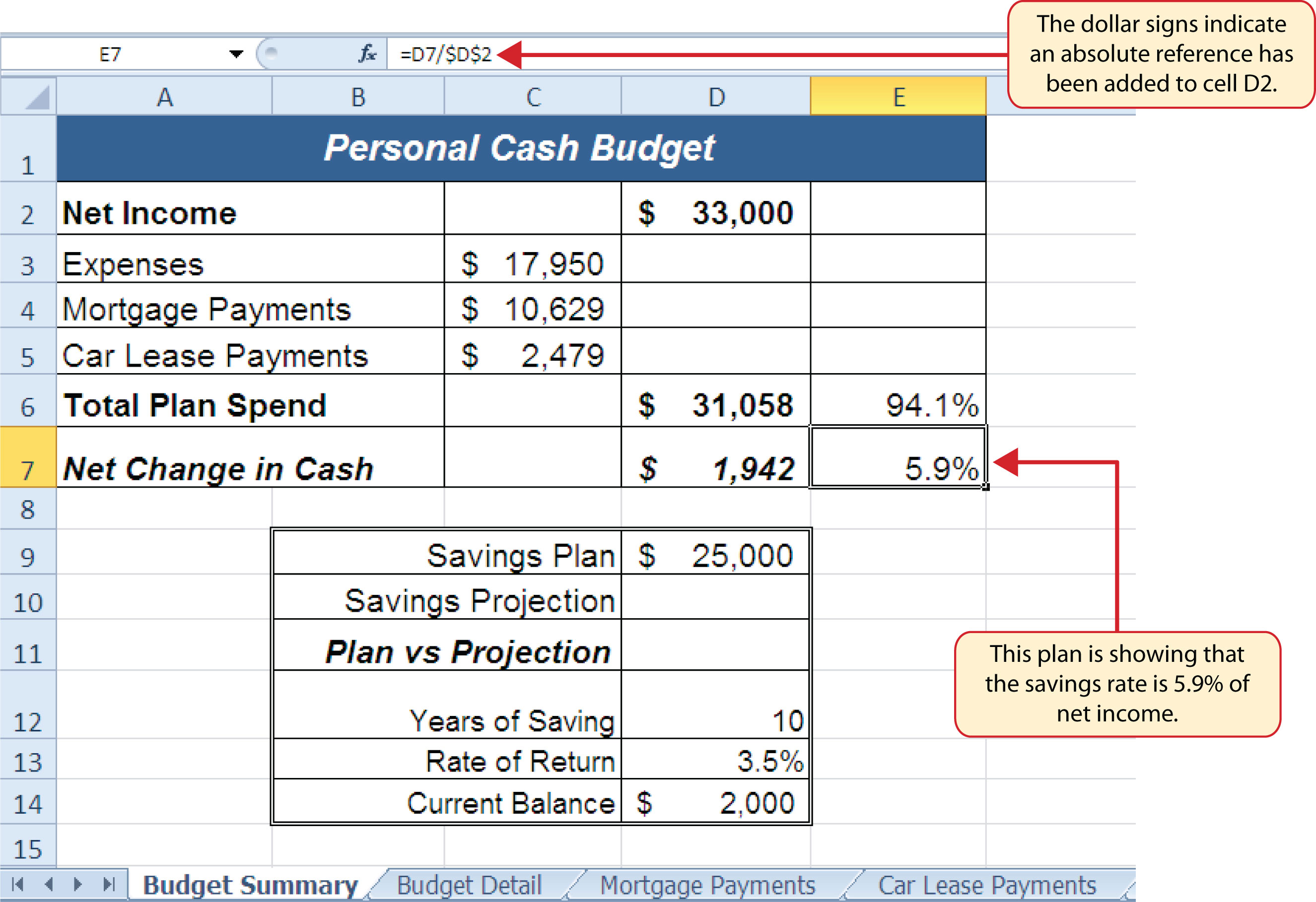## Time Value of Money Concepts

Follow-along file: Continue with Excel Objective 2.00. (Use file Excel Objective 2.13 if starting here.)

In reviewing the Budget Summary worksheet in Figure 2.39 "Calculating the Savings Rate", you will notice that the range B9:D14 contains data that can be used to assess a savings plan. We can project how much money can be saved over a specific period of time given set contributions and a rate of return. This calculation is accomplished through the future value, or FV, function. We will use the FV function in cell D10 of the Budget Summary worksheet to calculate our savings plan projection. However, before we use the FV function, it is important to review a few basic concepts regarding the time value of money, as shown in Table 2.7 "Key Terms for Time Value of Money Concepts".

Table 2.7 Key Terms for Time Value of Money Concepts

Argument Definition
Annuity An investment that is made in regular payments over a period of time. For example, depositing \$100 a month into an interest-bearing bank account or mutual fund is considered an annuity.
Bonds An investment in which you lend money to a company or government entity. The borrower agrees to pay you interest over a specific period time. At the end of the bond agreement, the amount of money that was borrowed, or your initial investment, is returned to you. Most bonds are considered a lower risk investment but offer a lower rate of return than stocks offer.
Mutual Funds A collection of similar investments managed by a financial professional called a fund manager. Mutual funds allow you to invest in several stocks or bonds without having to make many individual investments. They also allow you to reduce your risk and take advantage of the investment expertise of a professional.
Rate of Return The percentage gained or lost on an investment. Investments that offer a high predicted rate of return often carry a higher risk of losing money. Investments that offer a lower predicted rate of return often carry a lower risk of losing money.
Stocks An investment in which you own a portion of a company. The value of this investment increases as the company produces higher profits. Most stocks are expected to generate a higher rate of return than bonds generate. However, the risk of losing money on a stock investment is much greater than the risk for bonds.

Table 2.7 "Key Terms for Time Value of Money Concepts" provides definitions for several terms used when addressing the time value of money concepts. The time value of moneyThe opportunity to increase the value of money over time through investments that provide a constant or average positive rate of return. is the opportunity to grow your money over time given a constant or average rate of return. For example, consider the data shown in Figure 2.40 "Time Value of Money Example for a One-Time Investment". This data assumes that a person makes a one-time investment of \$100 in a bond mutual fund that returns 5% interest per year. Notice that the interest paid in Column E increases every year. This is because the interest is reinvested in the mutual fund, which increases the total value of the investment. For example, the interest earned in year 1 is based on a \$100 investment. Therefore, the interest paid is \$5.00, or 5% of \$100. However, in year 2, when the \$5.00 interest payment is reinvested, the total investment increases to \$105. Therefore, in year 2 the interest paid increases to \$5.25, or 5% of \$105. The value of the investment at the end of 5 years is \$127.63. This is the value that can be calculated using the FV function.

Figure 2.40 Time Value of Money Example for a One-Time Investment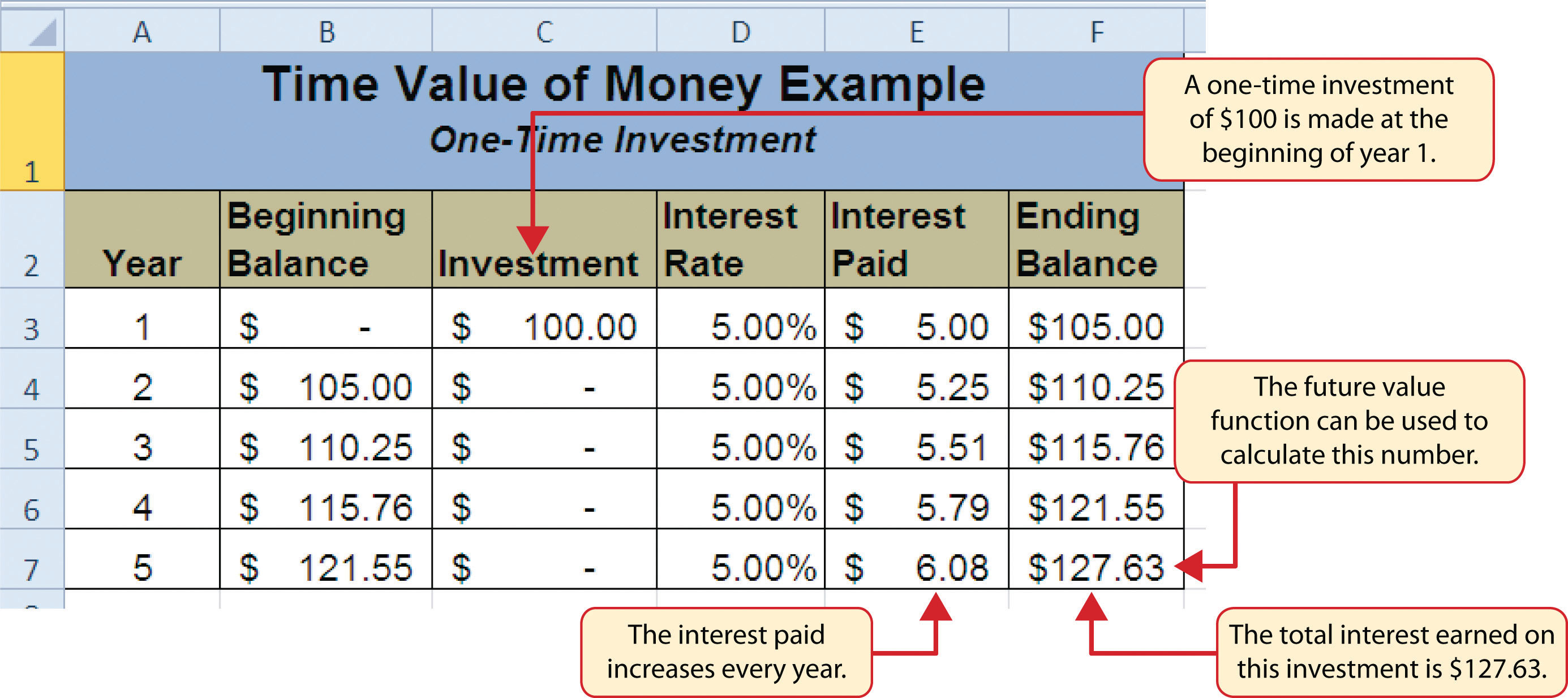Figure 2.41 "Time Value of Money Example for an Annuity Investment" shows another example demonstrating the time value of money concept. Instead of making a one-time investment, we will assume that a person invests \$100 at the beginning of every year in the same bond mutual fund. This is referred to as an annuityAn investment made in regular payments over a period of time. because the person is making reoccurring investments over a specific period of time. Notice that the value of this investment after 5 years is \$580.19. Also, the total interest earned on this investment is \$80.19 as opposed to the \$27.63 earned on the one-time investment in Figure 2.40 "Time Value of Money Example for a One-Time Investment".

Figure 2.41 Time Value of Money Example for an Annuity Investment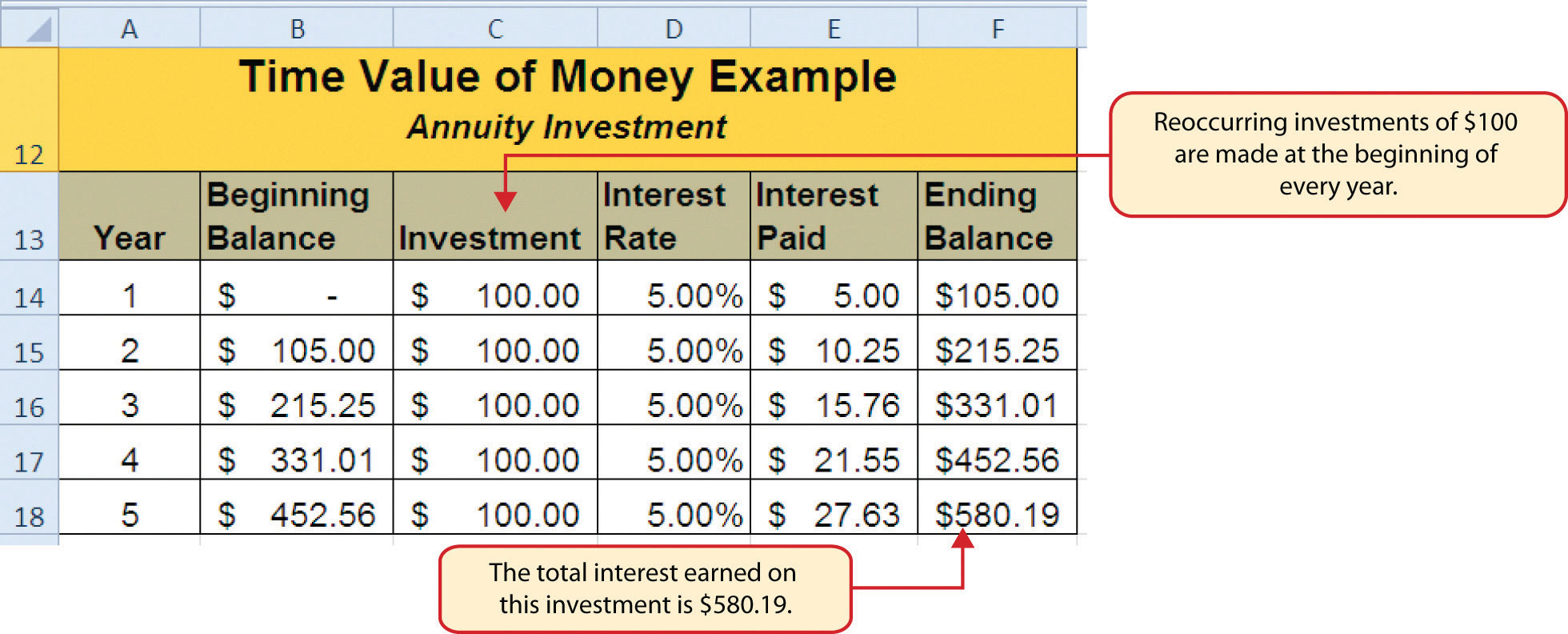## The FV (Future Value) Function

Follow-along file: Continue with Excel Objective 2.00. (Use file Excel Objective 2.13 if starting here.)

Establishing a personal savings plan is one of the most important financial exercises you can do. For example, a savings plan is critical for establishing financial security for your retirement years. Many people mistakenly believe that saving for retirement is something you do when you get older. However, the greatest financial gains for your retirement can be achieved if you start saving in the earliest years of your career. Now that you have an understanding of the time value of money, you can see that the more years you can earn interest on your investments and reinvest those earnings, the more money you will have when you retire. Savings plans are also important for other key life events, such as going to college or buying a home. The FV function is a convenient tool that can help you establish savings goals and project the value of your investments over time. Similar to the PMT function, the FV function requires you to accurately define specific arguments in order to produce a reliable result. Table 2.8 "Arguments for the FV Function" provides definitions for each of the arguments in the FV function. It is helpful to review the time value of money terms in Table 2.7 "Key Terms for Time Value of Money Concepts" before using the FV function.

Table 2.8 Arguments for the FV Function

Argument Definition
Rate This is the rate of return you expect to earn on an investment over time. This rate is usually quoted in annual terms, so you have to divide by 12 if you are calculating the value of an annuity making investments on a monthly basis.
Nper The argument letters stand for number of periods. This is the amount of time you are using to measure the value of an investment. The amount of time used to define this argument must be comparable to the Rate argument. For example, if the rate is stated in terms of months, the amount of time used to define this argument must be in months.
Pmt The argument letters stand for payment. This argument is used if you are measuring the value of an annuity investment. The argument is defined with the value of the investment that is made for each measure of time used to define the Nper argument. For example, if the Nper argument is expressed in terms of months, you must define this argument with the investment value that is made every month.
[Pv] The argument letters stand for present value. The brackets around the argument indicate that it is not always necessary to define it. Excel assumes zero if the argument is not defined. The argument is used when measuring the value of a one-time investment. Both this argument and the Pmt argument will be defined if an annuity investment has a beginning balance or includes a beginning one-time lump-sum investment.
[Type] This argument can be defined with either a 1 or a 0. The number 1 is used if investments are made at the beginning of each period used to define the Nper argument. A 0 is used if the investments are made at the end of each period. The argument is in brackets because it does not have to be defined if your investments are made at the end of each period. Excel assumes that this argument is 0 if it is not defined.

With respect to the Personal Budget workbook, we will use the FV function to project the value of the savings plan in 10 years. We will type the function directly into the Personal Budget worksheet for this demonstration. However, you can use any of the methods demonstrated in this chapter for future use. The following steps explain how this function is added to the worksheet:

1. Click cell D10 in the Budget Summary worksheet.
2. Type an equal sign (=).
3. Type the letters FV followed by an open parenthesis (().
4. Click cell D13. This is the expected rate of return for the investments.
5. Type a comma.
6. Click cell D12. This is the amount of time the investments are expected to grow.
7. Type a comma.
8. Type a minus sign (). All values or cell locations used to define the Pmt argument must be preceded by a minus sign.
9. Click cell D7. This is the change in cash that was calculated by subtracting the total expenses from the net income. We are expecting to save this amount of money for the 10-year period this investment is being measured.
10. Type a comma.
11. Type a minus sign (). All values and cell locations used to define the Pv argument must be preceded by a minus sign.
12. Click cell D14. Since the savings plan has a current balance, we use this to define the Pv argument of the function. This is equivalent to starting with a lump-sum investment.
13. Type a closing parenthesis ()). There is no need to define the last argument of the function because we will assume that the savings in cash achieved in our budget will be invested at the end of each year of the savings plan.
14. Press the ENTER key. Check that cell D11 is activated.
15. Type an equal sign (=).
16. Click cell D10.
17. Type a minus sign () and then click cell D9. This subtracts the savings plan from the current savings plan projection.
18. Press the ENTER key.

### Integrity Check

PMT and FV Functions Produce Negative Results

If the results of the PMT function or FV function are negative, check the Pv or Pmt arguments. Remember that these arguments must be preceded by a minus sign. If the minus sign is omitted, the functions produce a negative output.

Figure 2.42 "Results of the Savings Plan Projections" shows the results of the FV function. Notice that the current savings plan projection is \$25,606. This is \$606 higher than the target of \$25,000 entered into cell D9, which shows that the current budget is working to achieve the goals of this savings plan. In other words, given the current net income, we are saving enough money to achieve our savings plan goals.

There are two important factors to notice with regard to this plan. The first factor is that our spending plan allows us to save enough money so that it can be invested to achieve our target of \$25,000. The second factor is that the expected rate of return is 3.5%. This is a relatively low expected rate of return and could be achieved by investing in relatively low-risk investments such as bonds as opposed to stocks. This rate can be considered good because we can achieve our savings goals without having to make high-risk investments that could result in a significant loss of our savings.

Figure 2.42 Results of the Savings Plan Projections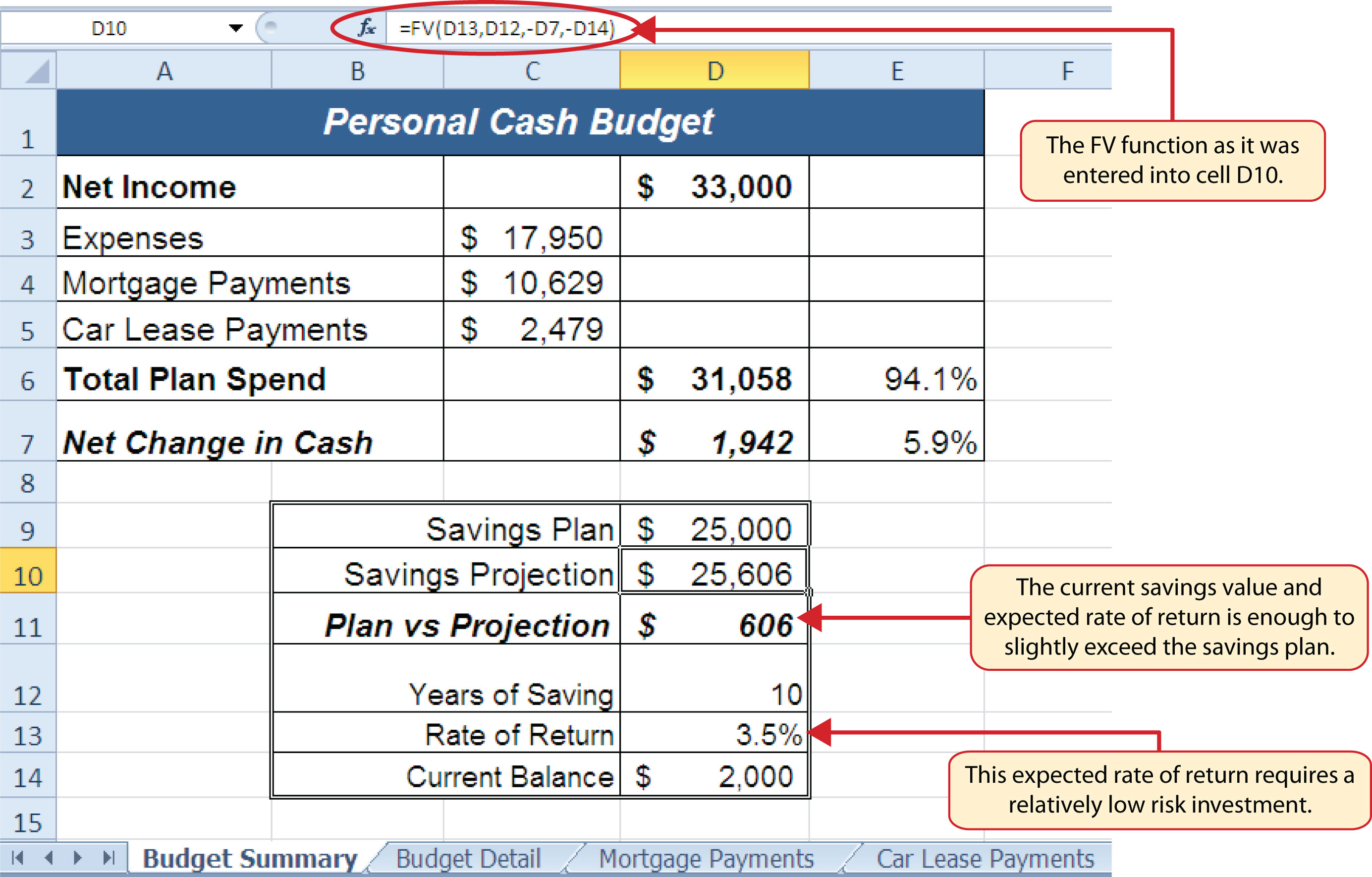### Skill Refresher: FV Function

1. Type an equal sign (=).
2. Type the letters FV followed by an open parenthesis, or double click the function name from the function list.
3. Define the Rate argument with a cell location that contains the expected rate of return for your investment.
4. Define the Nper argument with a cell location that contains the amount of time you are measuring the growth of your investment.
5. Define the Pmt argument with a cell location that contains the value of regular investments for an annuity. Cell locations or values used for this argument must be preceded by a minus sign.
6. Define the [Pv] argument with a cell location that contains the value of a one-time lump-sum investment. Cell locations or values used for this argument must be preceded by a minus sign.
7. Define the [Type] argument with a 1 if annuity investments are made at the beginning of each period or a 0 if investments are made at the end of each period.
8. Type a closing parenthesis ()).
9. Press the ENTER key.

## Goal Seek (What-If Scenarios)

Follow-along file: Continue with Excel Objective 2.00. (Use file Excel Objective 2.14 if starting here.)

We used several formulas and functions to complete the Personal Budget workbook shown in Figure 2.42 "Results of the Savings Plan Projections". All the formulas and functions entered contain cell references that allow for a variety of what-if scenarios. Goal Seek is a tool that can be used in the process of conducting these what-if scenarios. Goal Seek maximizes the benefits of Excel’s cell-referencing capabilities by changing inputs to precise values to achieve specific outputs produced by formulas or functions. We will begin by changing one of the inputs in the Personal Budget workbook through the following steps:

1. Click the Budget Detail worksheet tab.
2. Click cell D9.
3. Type the number 2000. Instead of planning a decrease in our vacation spending, we will see what happens to our budget if we spend the same amount as last year, which was \$2,000.
4. Press the ENTER key.

Figure 2.43 "Budget Detail Worksheet " and Figure 2.44 "Budget Detail Worksheet " show the Budget Detail worksheet before and after the change in the annual vacation budget. By comparing these two figures you can see that by changing just one input, many of the outputs produced by the formulas and functions in the worksheet changed. The following is a list of the changes that occurred in the worksheet:

• The formula output in cell F12 now shows that we are planning a 1.1% increase in our total spending as opposed to a −1.7% decrease.
• The formula output in cell F9 changes from −25% to 0%.
• The SUM function in cell D12 changes from \$17,950 to \$18,450.
• The SUM function in cell C12 changes from \$1,496 to \$1,538.
• The AVERAGE function in cell D14 changes from \$1,994 to \$2,050.

Figure 2.43 Budget Detail Worksheet before Changing the Annual Vacation Budget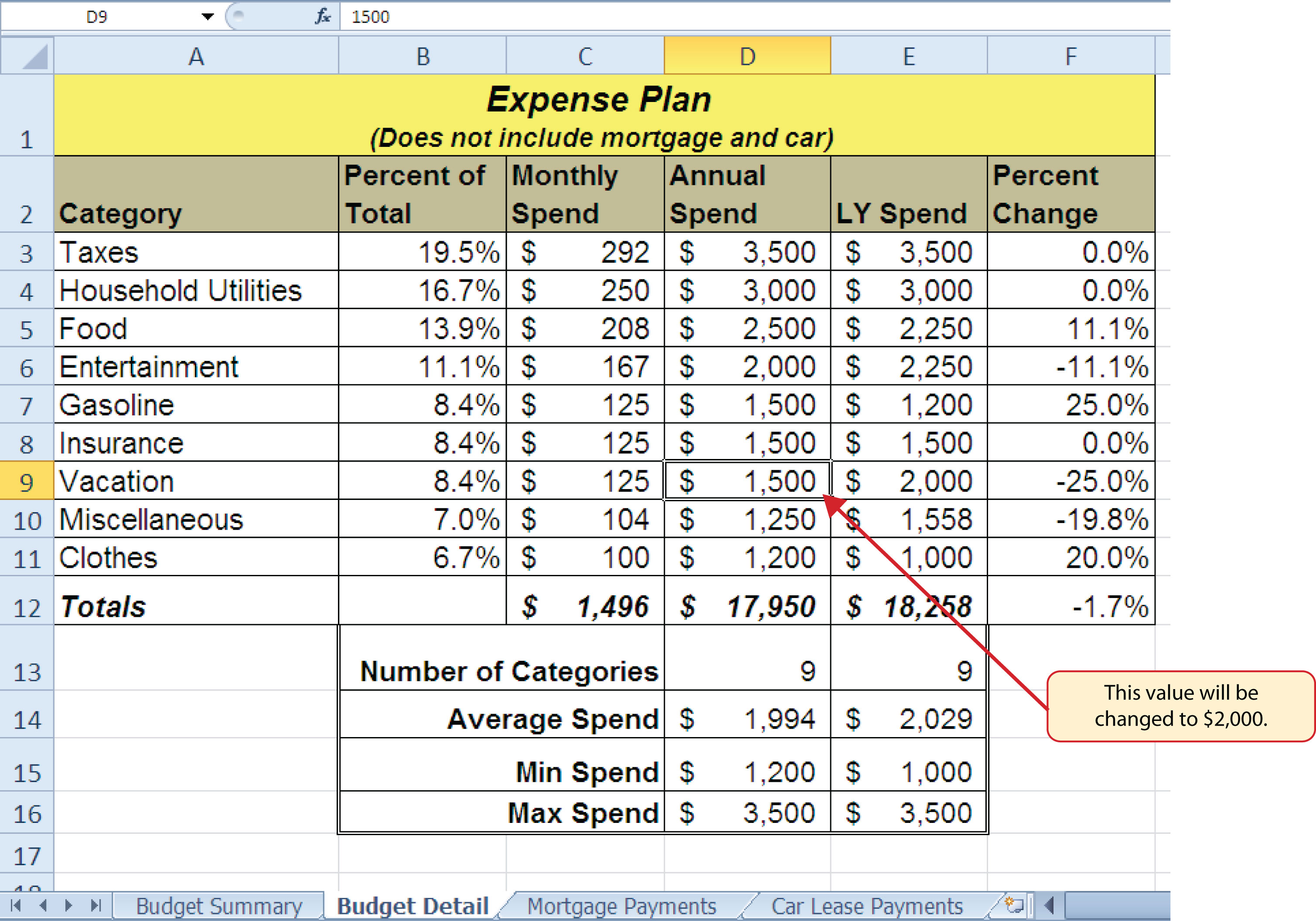Figure 2.44 Budget Detail Worksheet after Changing the Annual Vacation Budget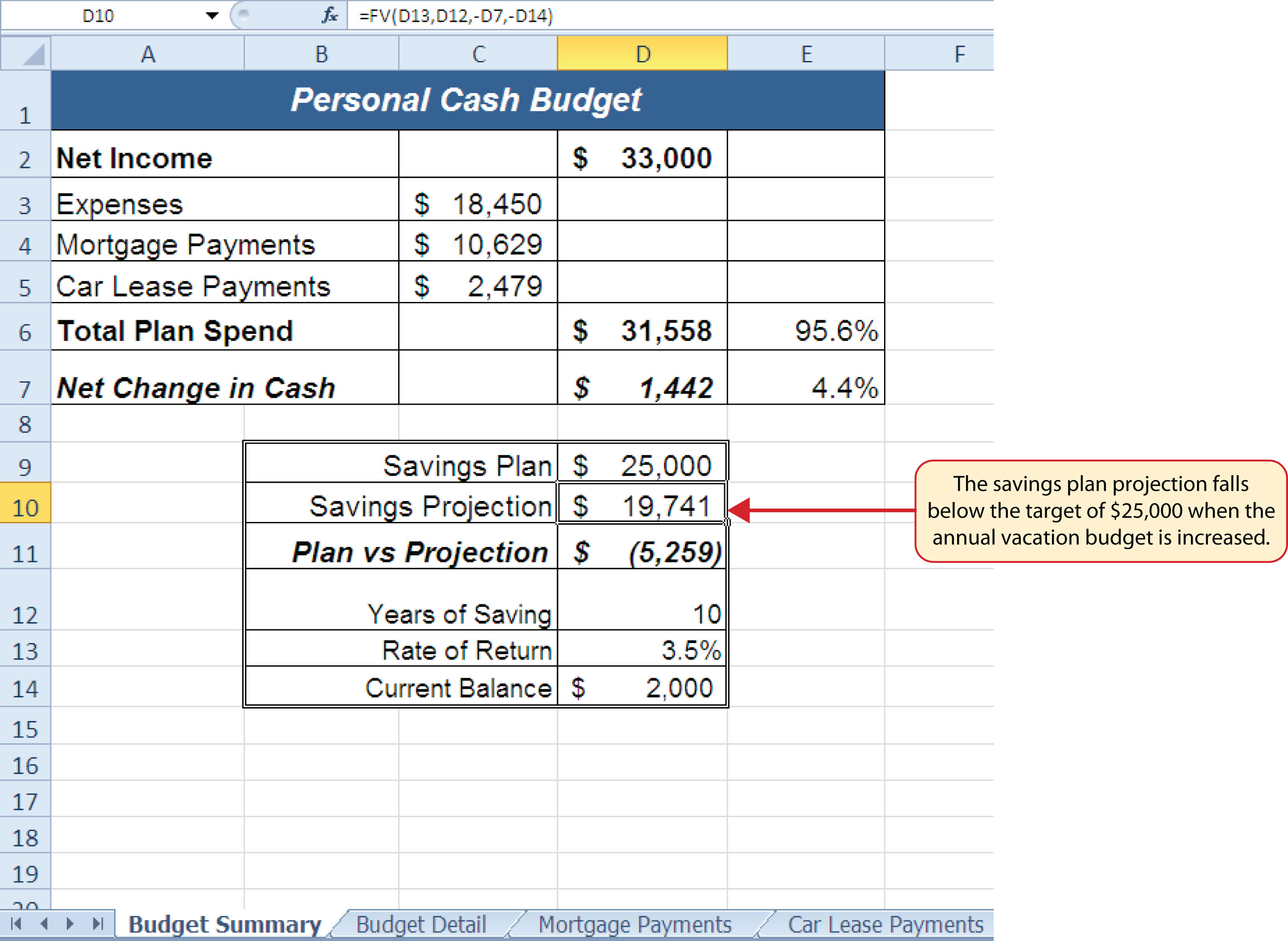In addition to the changes in the Budget Detail worksheet, outputs of formulas and functions on the Budget Summary worksheet also change when the Annual Spend for the Vacation category was increased. To see the changes, compare Figure 2.42 "Results of the Savings Plan Projections" to Figure 2.45 "Budget Summary Worksheet ". There were a total of fourteen changes in the outputs of formulas and functions on the Budget Summary worksheet. In total, there were twenty-one outputs that changed in the Personal Budget workbook as a result of changing just one input.

Figure 2.45 Budget Summary Worksheet after Changing the Annual Vacation Budget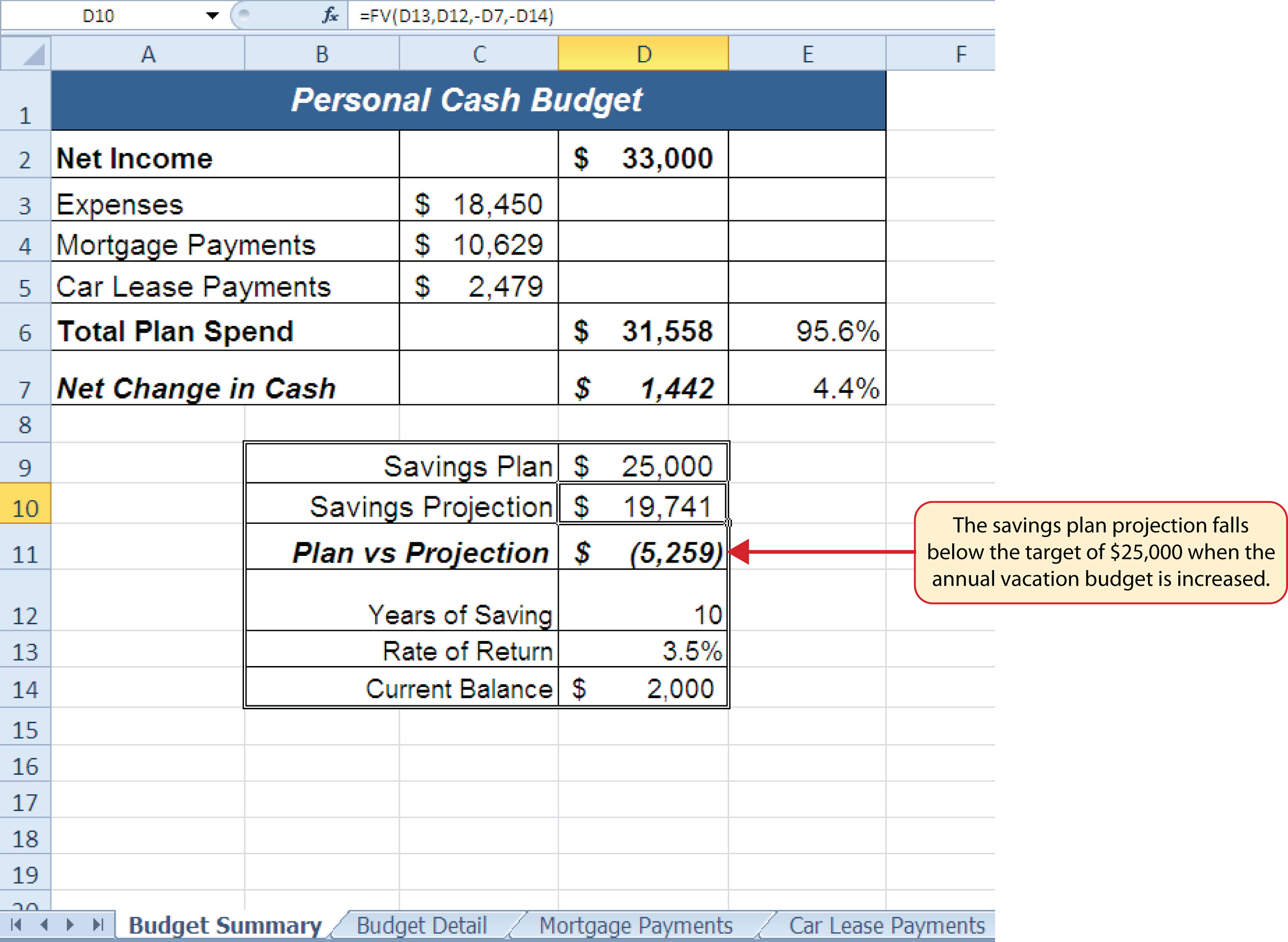One of the most notable changes on the Budget Summary worksheet is the Savings Projection in cell D10. By spending an additional \$500 a year on vacation plans, the projected savings value in 10 years decreases by \$5,865. However, what if the rate of return were to increase? An increase in the rate of return could recover the decrease in the future value of our savings plan. We can use a tool such as Goal Seek to determine exactly how much the rate of return would have to increase to achieve our savings plan target of \$25,000. The following steps explain how to use Goal Seek to accomplish this goal:

1. Click the Budget Summary worksheet tab.
2. Click the Data tab of the Ribbon.
3. Click the What-If Analysis button in the Data Tools group of commands.
4. Click Goal Seek from the list options (see Figure 2.46 "Selecting Goal Seek from the What-If Analysis Options"). This opens the Goal Seek dialog box.

### Mouseless Commands

Goal Seek

• Press the Alt key on your keyboard and then the letters A, W, and G one at a time.

Figure 2.46 Selecting Goal Seek from the What-If Analysis Options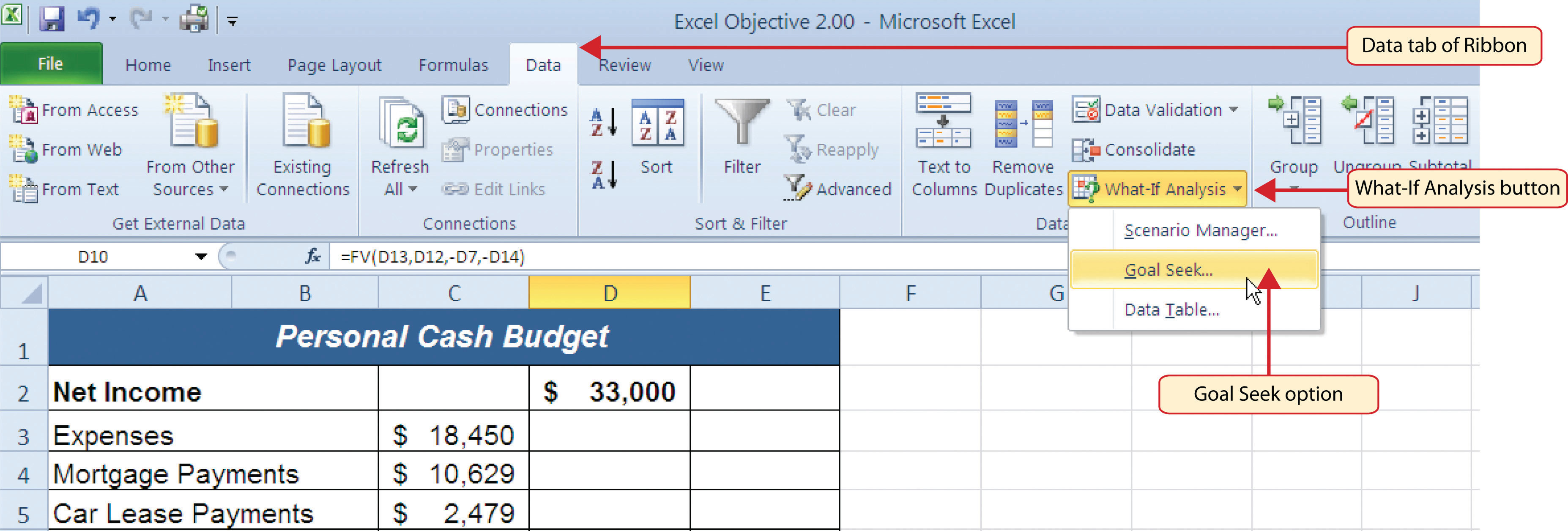5. Click the Collapse Dialog button next to the “Set cell:” input box on the Goal Seek dialog box.
6. Click cell D10 on the Budget Summary worksheet.
7. Press the ENTER key on your keyboard.
8. Place the mouse pointer over the “To value” input box in the Goal Seek dialog box and click.
9. Type the number 25000 in the “To value” input box in the Goal Seek dialog box.
10. Click the Collapse Dialog button next to the “By changing cell” input box in the Goal Seek dialog box.
11. Click cell D13 on the Budget Summary worksheet.
12. Press the ENTER key on your keyboard.
13. Click the OK button on the Goal Seek dialog box.
14. Click the OK button on the Goal Seek Status dialog box (see Figure 2.48 "Solution Calculated by Goal Seek"). The status box is telling you that Excel found a value for cell D13 that produces an output of \$25,000 for the FV function in cell D10.
15. Figure 2.47 "Final Settings for the Goal Seek Dialog Box" shows the final settings for the Goal Seek dialog box before clicking the OK button.

Figure 2.47 Final Settings for the Goal Seek Dialog BoxFigure 2.48 "Solution Calculated by Goal Seek" shows the solution Goal Seek calculated for the rate of return. Notice that in order to achieve the target savings plan of \$25,000, the rate of return must increase to 7.8%. Initially, it appears that we can spend the additional \$500 a year on vacations and still achieve our savings goal of \$25,000. However, achieving a 7.8% annual rate of return will require us to make riskier investments with our savings. Thus, there is a greater possibility that we could lose a substantial amount of our savings. This is the downside of decreasing your overall savings rate. If you save less money, it forces you to take higher risks with the money you have in order to achieve higher rates of return. Unfortunately, many people end up on the losing end of these risks, which severely compromises their ability to reach their savings goals.

Figure 2.48 Solution Calculated by Goal Seek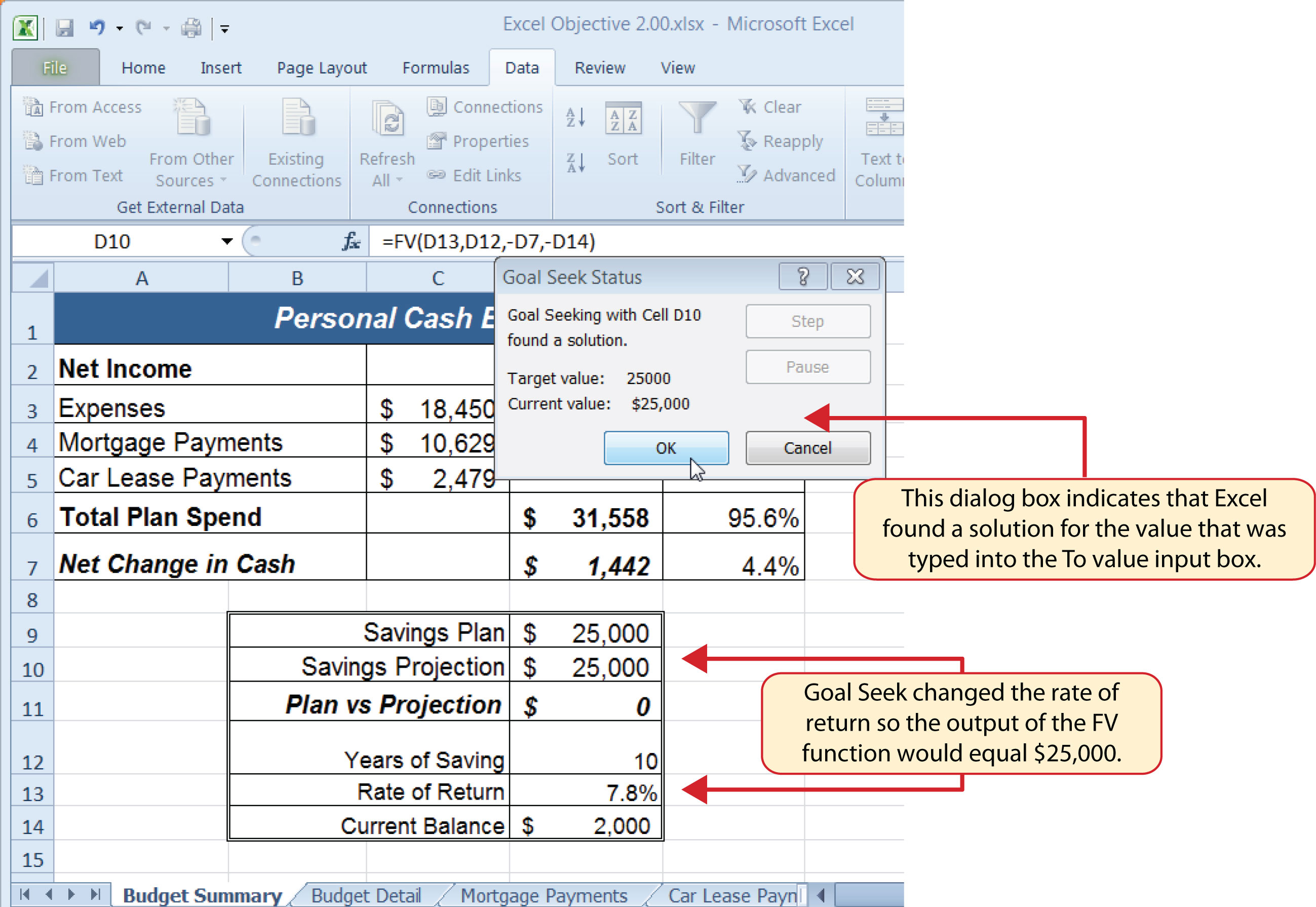### Skill Refresher: Goal Seek

1. Click the What-If Analysis button in the Data tab of the Ribbon.
2. Click the Goal Seek option.
3. Define the “Set cell” input box in the Goal Seek dialog box with a cell location that contains a formula or function.
4. Type a number in the “To value” input box in the Goal Seek dialog box. This is the number you want the formula or function to produce, which you defined for the “Set cell” input box.
5. Define the “By changing cell” input box in the Goal Seek dialog box with a cell location that is referenced in the formula or function used to define the “Set cell” input box.
6. Click the OK button on the Goal Seek dialog box.
7. Click the OK button on the Goal Seek Status dialog box.

### Key Takeaways

• The PMT function can be used to calculate the monthly mortgage payments for a house or the monthly lease payments for a car.
• When using the PMT or FV functions, each argument must be separated by a comma.
• When using the PMT or FV functions, the arguments must be defined in comparable terms. For example, when using the FV function, if the Pmt argument is defined using monthly payments, the Rate and Nper arguments must be defined in terms of months.
• The FV function is used to calculate the value an investment at a future point in time given a constant rate of return.
• The PMT and FV functions produce a negative output if the Pmt or Pv arguments are not preceded by a minus sign.
• Goal Seek is a valuable tool for creating what-if scenarios by changing the value in a cell location referenced in either a formula or a function.

### Exercises

1. Which statement best explains the setup of the following payment function: =PMT(.06,30,−200000,50000,0)? Note that the 6% annual interest rate is expressed in decimal terms as .06.

1. The function is calculating the monthly payments of a \$200,000 loan, 6% interest rate, over 30 years, with a lump-sum payment of \$50,000 at the end of the loan. Payments are due at the end of every month.
2. The function is calculating the annual payments of a \$200,000 loan, 6% interest rate, over 30 years, with a lump-sum payment of \$50,000 at the end of the loan. Payments are due at the end of every year.
3. The function is calculating the monthly payments of a \$200,000 loan, 6% interest rate, over 30 years, with a lump-sum payment of \$50,000 at the end of the loan. Payments are due at the beginning of every month.
4. The function is calculating the annual payments of a \$200,000 loan, 6% interest rate, over 30 years, with a lump-sum payment of \$50,000 at the end of the loan. Payments are due at the beginning of every year.
2. When leasing a car, the residual value will be used to define which of the following?

1. the Pv argument in the FV function
2. the Pv argument in the PMT function
3. the Pmt argument in the FV function
4. the Fv argument in the PMT function
3. The recurring investments in an annuity investment would be used to define which of the following?

1. the Pmt argument in the FV function
2. the Pv argument in the FV function
3. the Fv argument in the PMT function
4. the Pv argument in the PMT function
4. Which of the following PMT functions will accurately calculate the monthly payments on a mortgage if the price of the house is \$300,000, a down payment of \$60,000 is made, the interest rate is 5%, the term of the loan is 30 years, and payments are due at the end of every month?

1. =PMT(.05/12,30*12,−300000,60000,0)
2. =PMT(.05,30*12,−300000,60000,0)
3. =PMT(.05/12,30*12,−240000)
4. =PMT(.05/12,30,−240000,0)

## 2.4 Chapter Assignments and Tests

To assess your understanding of the material covered in the chapter, please complete the following assignments.

### Careers in Practice (Skills Review)

Financial Plan for a Lawn Care Business (Comprehensive Review)

Starter File: Chapter 2 CiP Exercise 1

Difficulty: Level 1 Easy

Running your own lawn care business can be an excellent way to make money over the summer while on break from college. It can also be a way to supplement your existing income for the purpose of saving money for retirement or for a college fund. However, managing the costs of the business will be critical in order for it to be a profitable venture. In this exercise you will create a simple financial plan for a lawn care business by using the skills covered in this chapter. Begin this exercise by opening the file named Chapter 2 CiP Exercise 1.

1. Click cell C5 in the Annual Plan worksheet.
2. Enter a formula that calculates the average price per lawn cut. Type an equal sign (=), then click cell B3. Type the asterisk symbol (*) for multiplication, then click cell B4. Press the ENTER key.
3. Click cell C8 in the Annual Plan worksheet.
4. Enter a formula that calculates the total number of lawns that will be cut during the year. Type an equal sign (=), then click cell B6. Type the asterisk symbol (*) for multiplication, then click cell B7. Press the ENTER key.
5. Click cell D9 in the Annual Plan worksheet.
6. Enter a formula that calculates the total sales for the plan. Type an equal sign (=), then click cell C5. Type the asterisk symbol (*) for multiplication, then click cell C8. Press the ENTER key.
7. Click cell F3 in the Leases worksheet. The PMT function will be used to calculate the monthly lease payment for the first item. For many businesses, leasing (or renting) equipment is a more favorable option than purchasing equipment because it requires far less cash. This enables you to begin a business such as a lawn care business without having to put up a lot of money to buy equipment.
8. Type an equal sign (=) followed by the function name PMT and an open parenthesis ((). Define the arguments of the function as follows:

• Rate: Click cell B3, type a forward slash (/) for division, type the number 12, and type a comma. Since we are calculating monthly payments, the annual interest rate must be converted to a monthly interest rate.
• Nper: Click cell C3, type an asterisk (*) for multiplication, type the number 12, and type a comma. Similar to the Rate argument, the terms of the lease must be converted to months since we are calculating monthly payments.
• Pv: Type a minus sign (), click cell D3, and type a comma. Remember that this argument must always be preceded by a minus sign.
• Fv: Click cell E3 and type a comma.
• Type: Type the number 1, type a closing parenthesis ()), and press the ENTER key. We will assume the lease payments will be made at the beginning of each month, which requires that this argument be defined with a value of 1.
9. Copy the PMT function in cell F3 and paste it into the range F4:F6.
10. Click cell F10 in the Leases worksheet. A SUM function will be added to calculate the total for the monthly lease payments.
11. Type an equal sign (=) followed by the word SUM and an open parenthesis ((). Highlight the range F3:F9, type a closing parenthesis ()), and press the ENTER key. You will notice that blank rows were included in this range for the SUM function. If other items are added to the worksheet, they will be included in the output of the SUM function.
12. Highlight the range A2:F6 on the Leases worksheet. The data in this range will be sorted.
13. Click the Sort button in the Data tab of the Ribbon. In the Sort dialog box, select the Interest Rate option in the “Sort by” drop-down box. Select Largest to Smallest for the sort order. Then, click the Add Level button on the Sort dialog box. Select the Price option in the “Then by” drop-down box. Select Largest to Smallest for the sort order. Click the OK button in the Sort dialog box.
14. Click cell B11 on the Annual Plan worksheet. The monthly lease payments that are calculated in the Lease worksheet will be displayed in this cell.
15. Type an equal sign (=). Click the Leases worksheet tab, click cell F10, and press the ENTER key.
16. Click cell C12 on the Annual Plan worksheet.
17. Type an equal sign (=) and click cell B11. Type an asterisk (*), type the number 12, and press the ENTER key. This formula calculates the annual lease payments.
18. Format the output of the formula in cell C12 so the decimal places are reduced to zero.
19. Click cell C14 on the Annual Plan worksheet.
20. Type an equal sign (=) and click cell B13. Type an asterisk (*), click cell C8, and press the ENTER key.
21. Click cell D16 on the Annual Plan worksheet.
22. Type an equal sign (=) followed by the word SUM and an open parenthesis ((). Highlight the range C11:C15, type a closing parenthesis ()), and press the ENTER key. This SUM function adds the total expenses for the business.
23. Click cell D17 on the Annual Plan worksheet.
24. Type an equal sign (=). Click cell D9, type a minus sign (), click cell D16, and press the ENTER key. This formula calculates the annual profit for the business.
25. Click cell B10 on the Investments worksheet.
26. Type an equal sign (=) followed by the word COUNT and an open parenthesis ((). Highlight the range B3:B8, type a closing parenthesis ()), and press the ENTER key. This function counts the number of investments that currently have a balance. Notice that additional blank rows were included in the range for this function. The function output will automatically change if any new investments are added to the worksheet.
27. Click cell D3 on the Investments worksheet.
28. Type an equal sign (=). Click the Annual Plan worksheet tab. Click cell D17 and type a forward slash (/) for division. Click the Investments worksheet tab. Click cell B10 and press the ENTER key. This formula divides the profit calculated on the Annual Plan worksheet by the number of investments in the Investments worksheet. We will assume that the profits from this business will be invested evenly among the funds listed in Column A of the Investments worksheet.
29. Before copying and pasting the formula created in step 28, absolute references must be added to the cell locations in the formula. Double click cell D3 on the Investments worksheet. Place the mouse pointer in front of D17 in the formula and click. Press the F4 key on your keyboard. Place the mouse pointer in front of cell B10 in the formula and click. Press the F4 key on your keyboard. Press the ENTER key.
30. Copy cell D3 and paste it into cells D4 and D5.
31. Click cell E3 on the Investments worksheet. The future value function will be added to project the total growth of the investments listed in Column A. We will assume that the business will be able to consistently generate the profit, which will be invested evenly in the funds every year.
32. Type an equal sign (=) followed by the function name FV and an open parenthesis ((). Define the arguments of the function as follows:

• Rate: Click cell C3 and type a comma. This is the expected growth rate of the first fund.
• Nper: Type the number 10 and then type a comma. We will project the growth of these investments in 10 years.
• Pmt: Type a minus sign (), click cell D3, and type a comma. Remember that this argument must always be preceded by a minus sign. We are assuming that the business will consistently generate the profits calculated in the Annual Plan worksheet and that these profits will be invested evenly into each fund.
• Pv: Type a minus sign () and click cell B3. Since each fund currently has a balance, we need to add this to the Pv argument of the function. Similar to the Pmt argument, remember that this argument must also be preceded by a minus sign.
• Type: Type a closing parenthesis ()) and press the ENTER key. We will assume the investments will be made at the end of each year. Therefore, it is not necessary to define this argument since Excel will assume zero, or end of the period, if it is not defined.
33. Copy the FV function in cell E3 and paste it into cells E4 and E5.
34. Click cell B9 on the Investments worksheet.
35. Type an equal sign (=) followed by the word SUM and an open parenthesis ((). Highlight the range B3:B8, type a closing parenthesis ()), and press the ENTER key. This SUM function adds the current balance for all investments. Blank rows are added to the range for the function so additional investments will automatically be included in the function output.
36. Copy the SUM function in cell B9 and paste it into cells D9 and E9.
37. We will use Goal Seek to determine how many customers we need to service in order to reach a savings goal of \$250,000. Click cell E9 on the Investments worksheet. Click the What-If Analysis button in the Data tab of the Ribbon and select Goal Seek. Click in the “To value” input box on the Goal Seek dialog box. Type the number 250000. Click the Collapse Dialog button next to the “By changing cell” input box on the Goal Seek dialog box. Click the Annual Plan worksheet tab and click cell B6. Press the ENTER key, and click the OK button on the Goal Seek dialog box. Click the OK button on the Goal Seek Status dialog box. View the number of customers showing in cell B6 in the Annual Plan worksheet.
38. Save the workbook by adding your name in front of the current workbook name (i.e., “your name Chapter 2 CiP Exercise 1”).
39. Close the workbook and Excel.

Figure 2.49 Completed CiP Exercise 1 Annual Plan Worksheet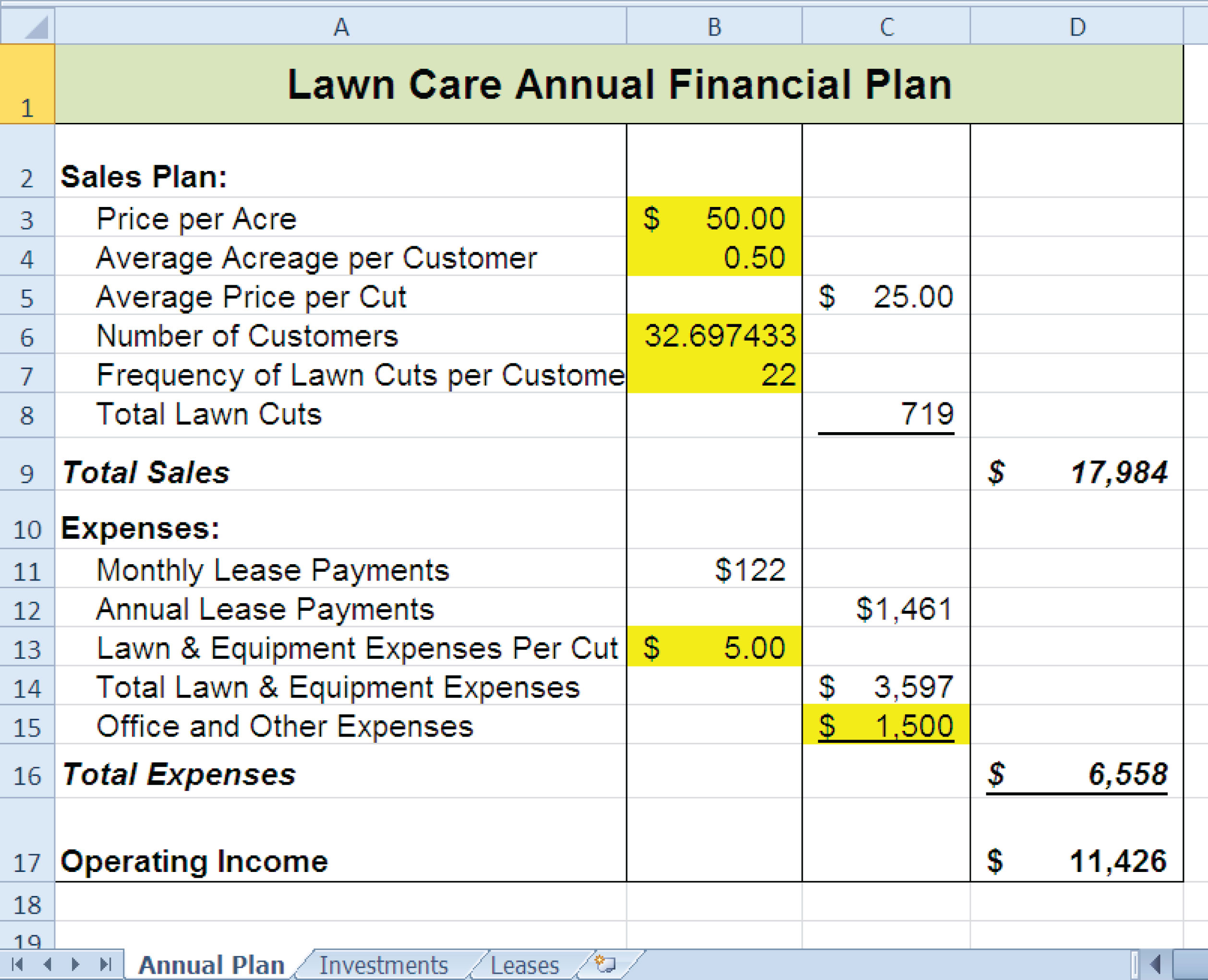Figure 2.50 Completed CiP Exercise 1 Investments Worksheet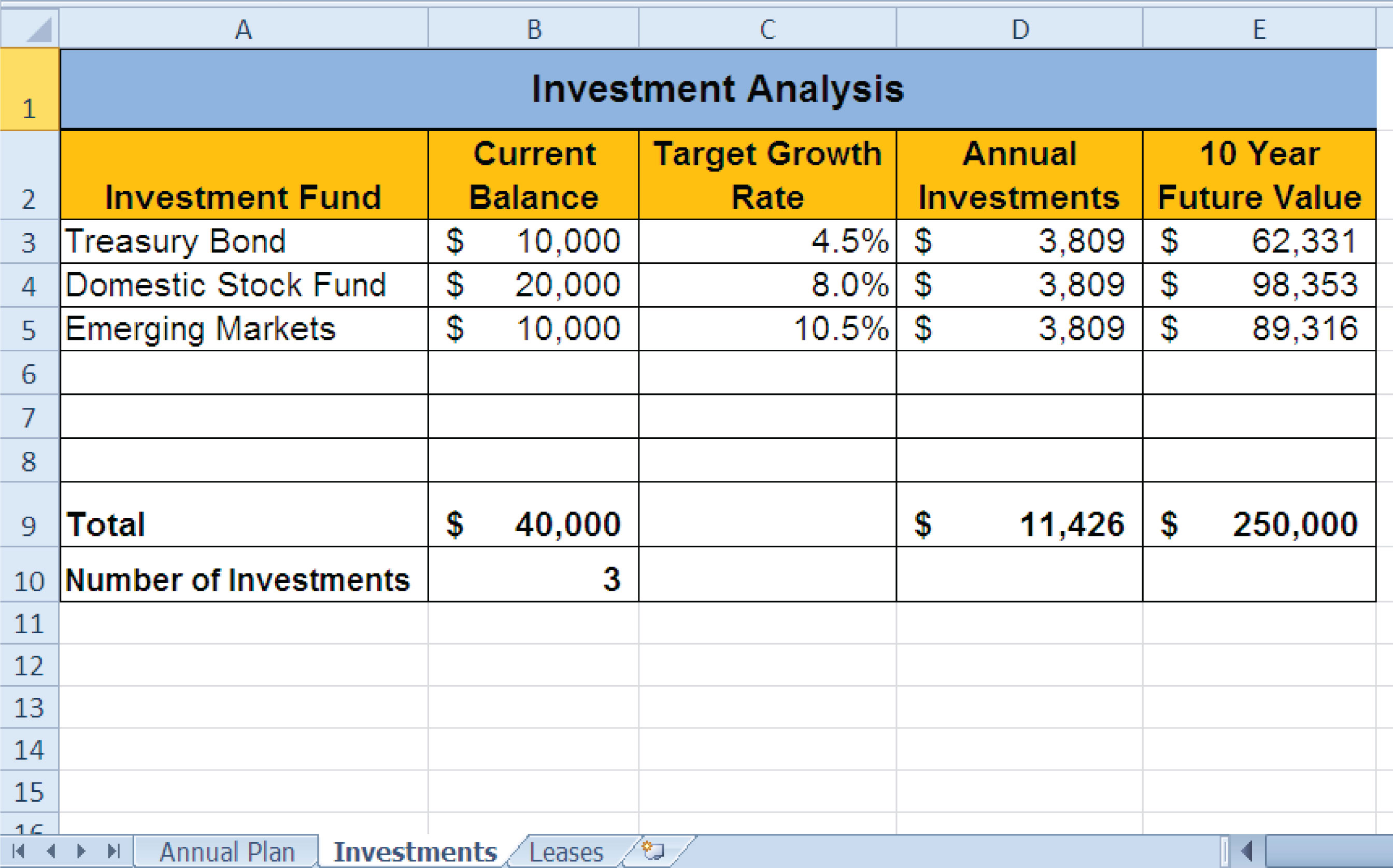Figure 2.51 Completed CiP Exercise 1 Leases Worksheet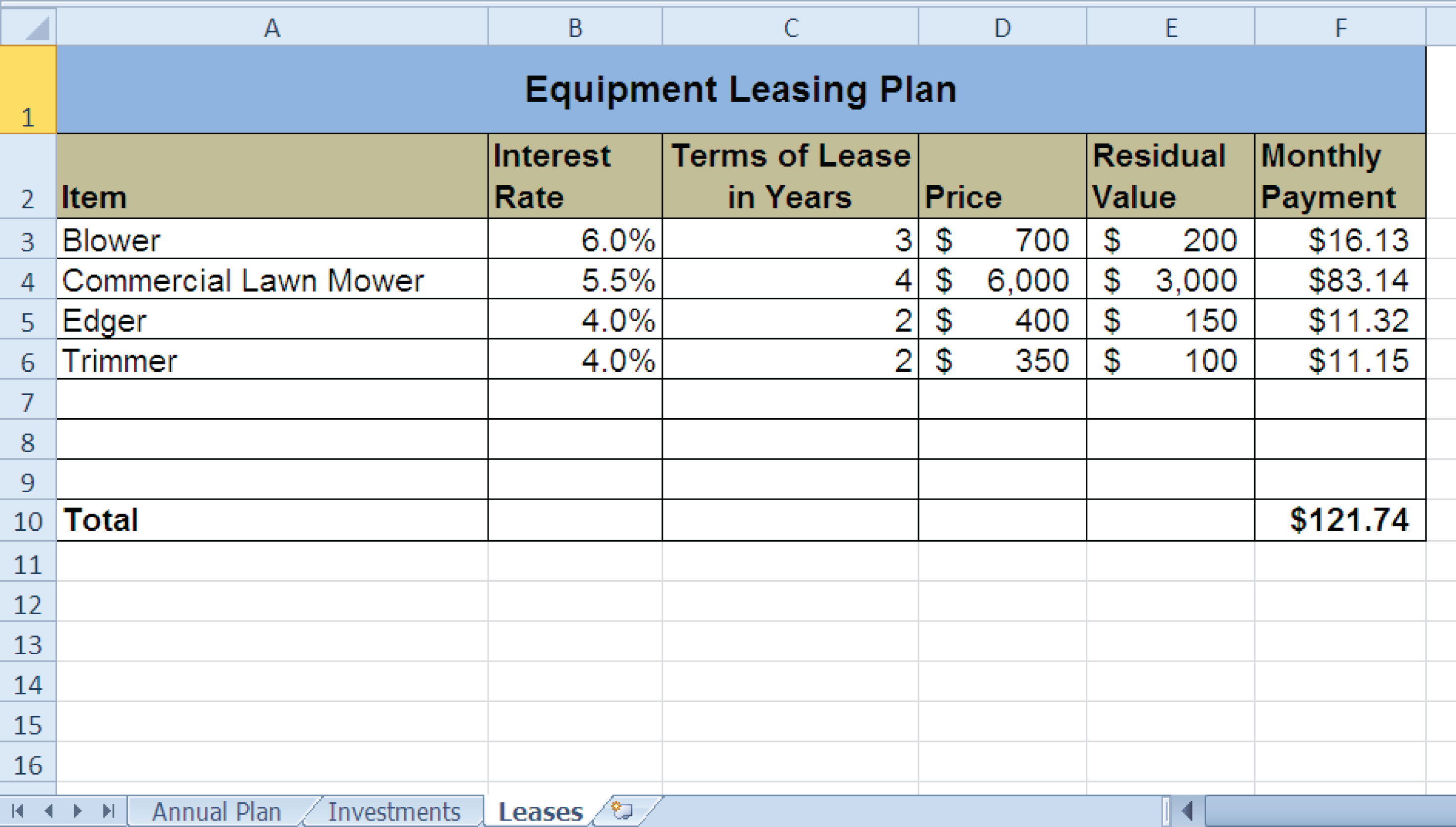Hotel Management Cost Analysis

Starter File: Chapter 2 CiP Exercise 2

Difficulty: Level 2 Moderate

The hotel management industry presents a wide variety of career opportunities. These range from running your own bed and breakfast to a management position at a large hotel corporation. No matter what hotel management career you choose to pursue, understanding the costs for any hotel operation is critical to running a successful operation. This exercise examines the relationship between cleaning expenses and the occupancy rate of a small hotel. Cleaning expenses are obviously influenced by the occupancy rate of the hotel. As more rooms need to be cleaned, the amount of overall cleaning expenses increases. However, to accurately estimate these expenses, you need to know whether there is a baseline, or fixed portion, of these expenses that does not change no matter how many rooms need to be cleaned. In other words, if you pay a cleaning staff a fixed salary, it does not matter if they clean 1 room or 100 rooms; their salary will remain the same. However, you may need more cleaning supplies as the number of rooms that need to be cleaned increases. In addition, the replacement of guest necessities such as soap, shampoo, lotions, and so on will also increase as the number of rooms to be cleaned increases. This exercise will demonstrate how these costs can be estimated through a technique called the high-low method. Begin this exercise by opening the file named Chapter 2 CiP Exercise 2.

1. Enter a formula in cell C5 on the Historical Costs worksheet to calculate the January capacity for the hotel. The capacity is calculated by multiplying the occupants per room (cell C3) by the number of rooms in the hotel (cell C2). This result is then multiplied by the number of days in the month (cell C5). Construct this formula so that relative referencing does not change cells C3 and C2 when the formula is pasted into other cell locations in Column C.
2. Copy the formula in cell C5 and paste it into the range C6:C16. Use a paste method that does not remove the border at the bottom of cell C16.
3. Enter a formula in cell E5 on the Historical Costs worksheet to calculate the occupancy capacity of the hotel. Your formula should divide the Hotel Capacity into the Actual Capacity. Format your result to a percentage with two decimal places. Then copy and paste the formula into the range E6:E16. Use a paste method that does not remove the border at the bottom of cell E16.
4. Enter a function in cell C17 on the Historical Costs worksheet that sums the values in the range C5:C16. Copy the function and paste it into cells D17 and F17. Use a paste method that does not change the border on the right side of cell F17.
5. Copy the formula in cell E16 and paste it into cell E17. Use a paste method that does not change the border at the bottom of cell E17.
6. Sort the data in the Historical Costs worksheet based on the values in the Actual Occupancy column in descending order (largest to smallest). For any duplicate values in the Actual Occupancy column, sort using the values in the Cleaning Expenses column in descending order.
7. On the Cost Analysis worksheet, enter a function into cell B3 that shows the highest value in the range D5:D16 in the Actual Occupancy column on the Historical Costs worksheet.
8. On the Cost Analysis worksheet, enter a function into cell B4 that shows the lowest value in the range D5:D16 in the Actual Occupancy column on the Historical Costs worksheet.
9. On the Cost Analysis worksheet, enter a function into cell C3 that shows the highest value in the range F5:F16 in the Actual Occupancy column on the Historical Costs worksheet.
10. On the Cost Analysis worksheet, enter a function into cell C4 that shows the lowest value in the range F5:F16 in the Actual Occupancy column on the Historical Costs worksheet.
11. On the Cost Analysis worksheet, format cells B3 and B4 with a comma and zero decimal places. Format cells C3 and C4 with US dollars with zero decimal places.
12. On the Cost Analysis worksheet, enter a formula in cell B5 that subtracts the lowest actual occupancy value from the highest actual occupancy value. Copy this formula and paste it into cell C5.
13. Enter a formula in cell C6 on the Cost Analysis worksheet that calculates that variable cost portion for the cleaning expenses per month. As mentioned in the introduction to this exercise, the cleaning expense contains costs that increase with each room that is cleaned. This is known as a variable expense and can be estimated by dividing the Actual Occupancy High Low Difference (cell B5) into the Cleaning Expenses High Low Difference (cell C5). Format the output of this formula to US dollars with two decimal places.
14. Enter a formula in cell C7 on the Cost Analysis worksheet that calculates the fixed cost portion for the cleaning expenses per month. This is the amount of money that will be spent on cleaning expenses no matter how many rooms are cleaned. Since we have calculated the variable cost portion of the cleaning expense, we can now use it to calculate the fixed expense. To do this, subtract from the High Cleaning Expense (cell C3) the result of multiplying the variable expense (cell C6) by the High Actual Occupancy (cell B3). Format the result of the formula to US dollars with zero decimal places.
15. Enter the number 3500 in cell C2 on the Cleaning Cost Estimates worksheet. Format the number with commas and zero decimal places.
16. Apply a yellow fill color to cell C2 on the Cleaning Cost Estimates worksheet. This is being formatted to indicate to the user of this worksheet that a number is to be entered into the cell.
17. On the Cleaning Cost Estimates worksheet, enter a formula in cell C3 that calculates the estimated cleaning expenses given the number that was entered into cell C2. Now that we have calculated the variable and fixed expenses on the Cost Analysis worksheet, we can use the results to estimate the cleaning expenses. The formula is a + bX, where a is the fixed cost, b is the variable cost, and X is the activity level that is typed into cell C2. The fixed cost is added to the result of multiplying the variable cost by the activity level in cell C2. Format the output of the formula to US dollars with zero decimal places.
18. Save the workbook by adding your name in front of the current workbook name (i.e., “your name Chapter 2 CiP Exercise 2”).
19. Close the workbook and Excel.

Figure 2.52 Completed CiP Exercise 2 Historical Costs Worksheet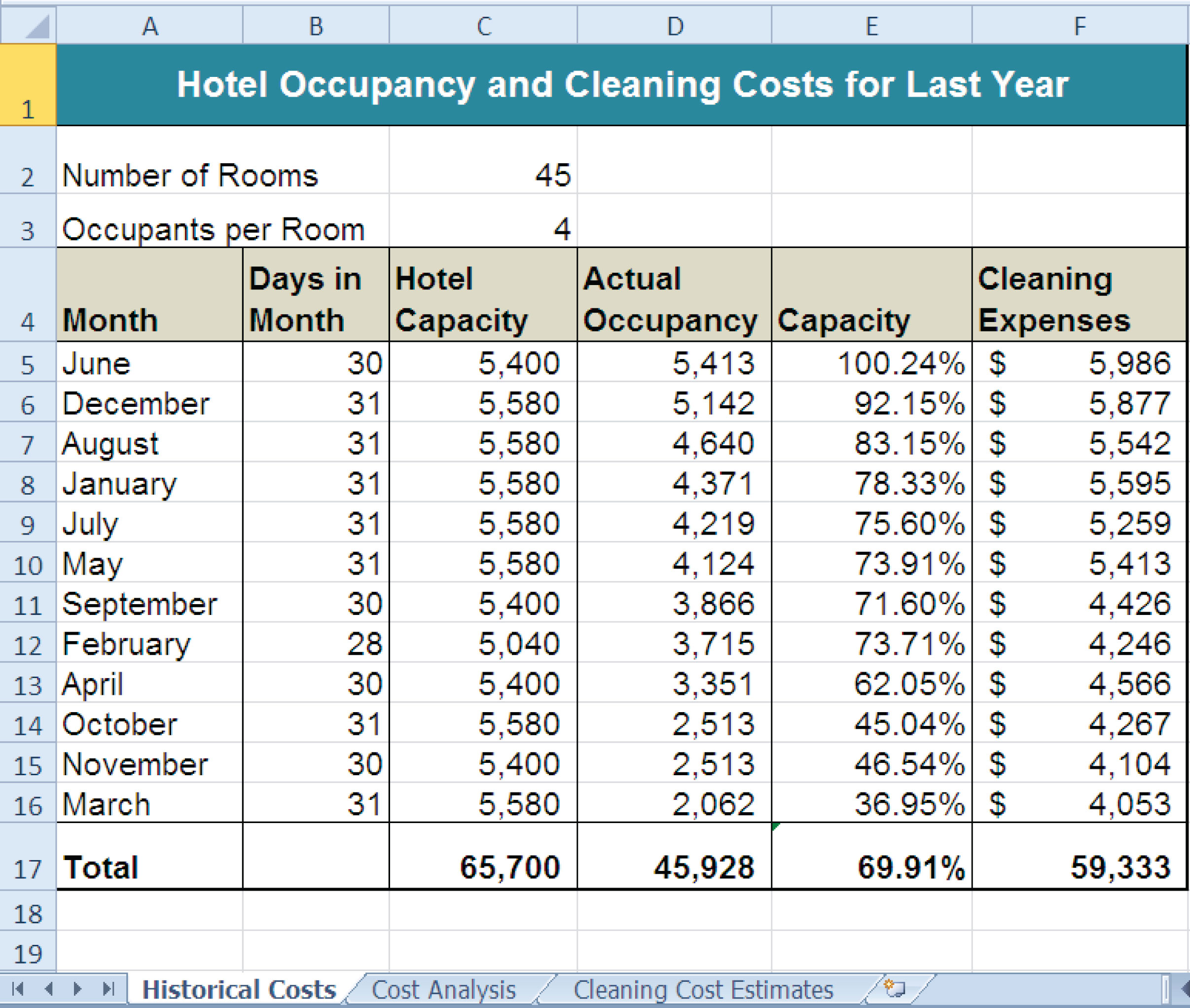Figure 2.53 Completed CiP Exercise 2 Cost Analysis Worksheet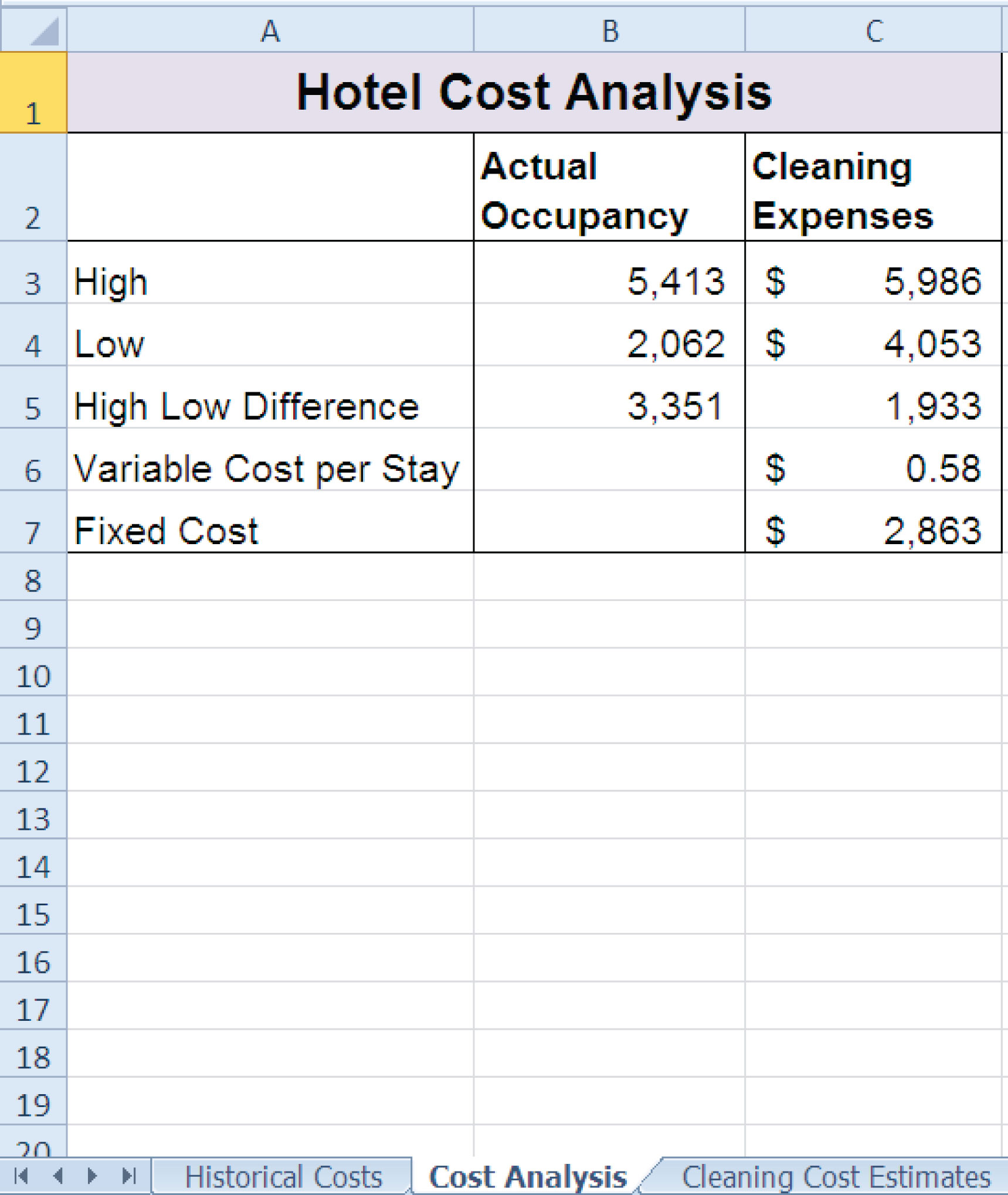### Integrity Check

Starter File: Chapter 2 IC Exercise 3

Difficulty: Level 3 Difficult

The purpose of this exercise is to analyze a worksheet to determine whether there are any integrity flaws. Read the scenario below, then open the Excel workbook related to this exercise. You will find a worksheet in the workbook named AnswerSheet. This worksheet is to be used for any written responses required for this exercise.

Scenario

You are the manager of a large do-it-yourself hardware store that is part of a national retail chain. Your assistant manager has constructed a sales and profit budget for the upcoming year. The Budget worksheet contains several formulas used to calculate the expected sales and profit dollars for the store by product category. The following is a list of key elements and calculations used on this worksheet:

• Cells shaded in yellow are intended for data entry values. For example, last year sales results in Column B are typed into the cells. Also, the expected growth rates in Column D and profit percentages in Column E are also typed into the cells. These values fluctuate from year to year, and the assistant manager intends to create a few scenarios for the budget by changing the growth rates and expected profit percentages for each product category.
• Table 2.9 "Formulas Used on the Budget Worksheet" contains a list of the formulas that are used to produce the outputs on the Budget worksheet.

Table 2.9 Formulas Used on the Budget Worksheet

Purpose Formula Location
Budgeted Profit Dollars Budgeted Sales × Profit Percent F4:F7
Budgeted Sales Sales Last Year × (1 + Sales Growth) C4:C7
Total Profit Growth (Total Budgeted Profit Dollars ÷ Total Budgeted Sales) E8
Total Sales Growth (Total Budgeted Sales − Total Sales Last Year) ÷ Total Sales Last Year D8

Assignment

1. As noted in Table 2.9 "Formulas Used on the Budget Worksheet", the Sales Last Year is used in the formula calculating the Budgeted Sales dollars. Use the Trace Dependents command to locate the formula referencing any value in the Sales Last Year column on the Budget worksheet. Document your observation in the AnswerSheet worksheet.
2. The assistant manager intends to use the Budget worksheet to create a few scenarios for the budgeted sales and profit dollars. Change a few values in the Profit Percent column and document your observations in the AnswerSheet worksheet.
3. Look at each value in the Totals row (row 8) on the Budget worksheet. Are there any values that do not make sense? Type your answer on the AnswerSheet worksheet.
4. Using Table 2.9 "Formulas Used on the Budget Worksheet" as a guide, evaluate all formulas that were entered into the Budget worksheet. Make any necessary corrections to the worksheet so when any value is changed in Columns B, D, and E, new outputs are created.
5. Save the workbook by adding your name in front of the current workbook name.

Starter File: Chapter 2 IC Exercise 4

Difficulty: Level 3 Difficult

The purpose of this exercise is to analyze a worksheet to determine whether there are any integrity flaws. Read the scenario below, then open the Excel workbook related to this exercise. You will find a worksheet in the workbook named AnswerSheet. This worksheet is to be used for any written responses required for this exercise.

Scenario

Your friend is working on a few financial calculations in Excel and is asking for your assistance. The workbook that was given to you contains calculations for estimating the future value of investments and monthly mortgage calculations for purchasing a home. Your friend explained the following in an e-mail that was sent with the workbook:

• You will see in the Investment Plan worksheet that I have estimated the value of my investments in 5 years. My company is taking money out of my paycheck at the end of every month and investing it in the funds I have listed in Column A. I am pretty sure I did this right, but all my results in Column E are negative. I am not sure why this is happening.
• In the Mortgage Payments worksheet, I am trying to calculate the monthly payments for a house I am thinking about buying. However, the output of the function in cell B6 seems really high. There is no way I would be paying over \$9,000 a month in mortgage payments. Something must be wrong.
• I don’t want to spend more than \$775 a month for a mortgage. I thought I would be able to use Excel to determine what my target price for the house should be. My agent said that the current owners were probably willing to negotiate on the asking price for the house.

Assignment

1. Look at the FV function that was entered into cell E3 on the Investment Plan worksheet. Why is the output for this function negative?
2. Assume that the output of the FV function in cell E3 was a positive \$17,385 instead of negative. Does it make sense that given a 4.5% annual rate of return, starting balance of \$10,000, and an ongoing investment of \$900 per month that the value of the investment would be \$17,385 after 5 years?
3. Look at the PMT function in cell B6 on the Mortgage Payments worksheet. Is the function set up to calculate monthly payments?
4. You friend states that the target monthly mortgage payment is \$775. What Excel tool could you use to change the price in cell B2 on the Mortgage Payments worksheet so the mortgage payment is equal to \$775?
5. Based on your friend’s comments, make any necessary corrections to all the functions in the Investment Plan and Mortgage Payments worksheets. Set the price of the home in cell B2 on the Mortgage Payments worksheet so the monthly payment equals \$775.
6. Save the workbook by adding your name in front of the current workbook name.

### Applying Excel Skills

Starter File: None

Difficulty: Level 2 Moderate

You are in the process of getting a new car but are not sure if you should buy or lease. The price of the car you want is \$18,000, but you do not want to spend more than \$250 a month on car payments. If you lease the car, the terms of the lease will be 48 months at an annual interest rate of 5%. The residual value of the car will be set at \$9,000. If you buy the car, your bank will offer you a 7-year loan at an annual interest rate of 6%. You are not required to make a down payment with either the lease or loan options, and payments are made at the end of the month for both options.

Should you lease or buy the car given your budget limit of \$250 a month? Create a new workbook and design a worksheet that shows the difference between leasing and buying the car in terms of monthly payments. Use proper formatting so your worksheet is easy to read. Remember to use column and row headings, add a title to your worksheet, and rename the worksheet tab with an appropriate label. Include your name in the file name of the workbook.

Amortization Table for a Home Loan

Starter File: None

Difficulty: Level 3 Difficult

You are considering the purchase of a new home offered at a price of \$225,000. Create an amortization table in a new workbook that shows how much interest and principal you will pay each month for the duration of the loan. The following is a list of assumptions and requirements you need to consider for this assignment:

1. You will be making a down payment of 20% on the home (refer to Table 2.5 "Key Terms for Loans and Leases" for loan and lease terms).
2. The bank will offer you a loan at an annual interest rate of 5.5% for 30 years.
3. Your mortgage payments will be made at the end of each month.
4. You must construct the amortization table so that any change in the loan variables, down payment percent, length of loan, interest rate, and so on will automatically produce new outputs for each month of the amortization table.
5. The amortization table must show the interest payment, principal payment, and balance remaining to be paid on the loan for every month of the loan duration. The beginning balance for the last month of the loan should be equal to the principal payment in the last month. Refer to Figure 2.29 "Example of an Amortization Table" for establishing the format for the table.
6. Remember to use column and/or row headings, add a title to your worksheet, and rename the worksheet tab with an appropriate label.
7. Include your name in the file name of the workbook.

### Chapter Skills Test

Starter File: Chapter 2 Skills Test

Difficulty: Level 2 Moderate

Answer the following questions by executing the skills on the starter file required for this test. Answer each question in the order in which it appears. If you do not know the answer, skip to the next question. Open the starter file listed above before you begin this test.

1. Enter a function in cell B9 on the Investments worksheet that calculates the total of the values in the range B3:B8.
2. Copy the function in cell B9 and paste it into cells C9 and G9.
3. Enter a formula in cell E3 on the Investments worksheet that calculates the growth rate for the investments. Your formula should first subtract the value in the Invested Principal column from the value in the Current Balance column. Then, divide this result by the value in the Invested Principal column.
4. Copy the formula in cell E3 and paste it into the range E4:E8.
5. Copy the formula in cell E3 and paste it into cell E9 using the Paste Formulas option.
6. Enter a formula in cell D3 on the Investments worksheet that divides the Current Balance by the total in cell C9. Add an absolute reference to C9 in this formula.
7. Copy the formula in cell D3 and paste it into the range D4:D8.
8. In cell G3 on the Investments worksheet, use the Future Value function to calculate the future value of the investment in 2 years. Use the Target Growth Rate to define the Rate argument. This is not an annuity so there are no periodic investments. Use the Current Balance to define the Pv argument. Assume that the investment is made at the beginning of the period.
9. Copy the function in cell G3 and paste it into the range G4:G8.
10. Enter a function in cell B10 on the Investments worksheet that calculates the average of the values in the range B3:B8.
11. Copy the function in cell B10 and paste it into cells C10 and G10.
12. On the Mortgage worksheet, use the data provided to enter a formula in cell B6 to calculate the principal of the loan that will be required to purchase the house.
13. On the Mortgage worksheet, use the PMT function in cell B7 to calculate the monthly payments of the mortgage. Use cell locations from this worksheet to define each argument of the function. Assume that payments are made at the end of each month.
14. On the Auto Lease worksheet, use the PMT function in cell B6 to calculate the monthly lease payments. Use cell locations from this worksheet to define each argument of the function. Assume that the lease payments are due at the beginning of each month.
15. On the Auto Lease worksheet, use Goal Seek to change the Annual Interest rate in cell B2 so the monthly payments are exactly \$200.
16. In cell E2 on the Summary worksheet, use a cell reference to display the value in cell B9 in the Investments worksheet.
17. In cell E3 on the Summary worksheet, use a cell reference to display the value in cell G9 in the Investments worksheet.
18. Enter a formula in cell F4 on the Summary worksheet that subtracts the Principal of Investments from the 2 Year Future Value of Investments.
19. Enter a formula in cell F5 on the Summary worksheet that calculates the amount of mortgage payments that will be made over 2 years. Your formula should multiply the value in B7 on the Mortgage worksheet by 24.
20. Enter a formula in cell F6 on the Summary worksheet that calculates the amount of lease payments that will be made over 2 years. Your formula should multiply the value in B6 on the Auto Lease worksheet by 24.
21. Enter a formula in cell F7 on the Summary worksheet that subtracts the sum of the values in the range F5:F6 from the value in cell F4.
22. Sort the data in the range A2:G8 on the Investments worksheet. Sort the data based on the values in the Invested Principal column in ascending order (smallest to largest). For duplicate values in this column, sort using the values in the Target Growth Rate column in descending order (largest to smallest).
23. Save the workbook by adding your name in front of the current workbook name (i.e., “your name Chapter 2 Skills Test”).
24. Close the workbook and Excel.